# How to create a rim of an Audi R8 ?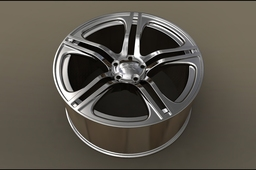.

1. ### Step 1:

I put a plan of a rim and I put on the front and center, see how to add the plans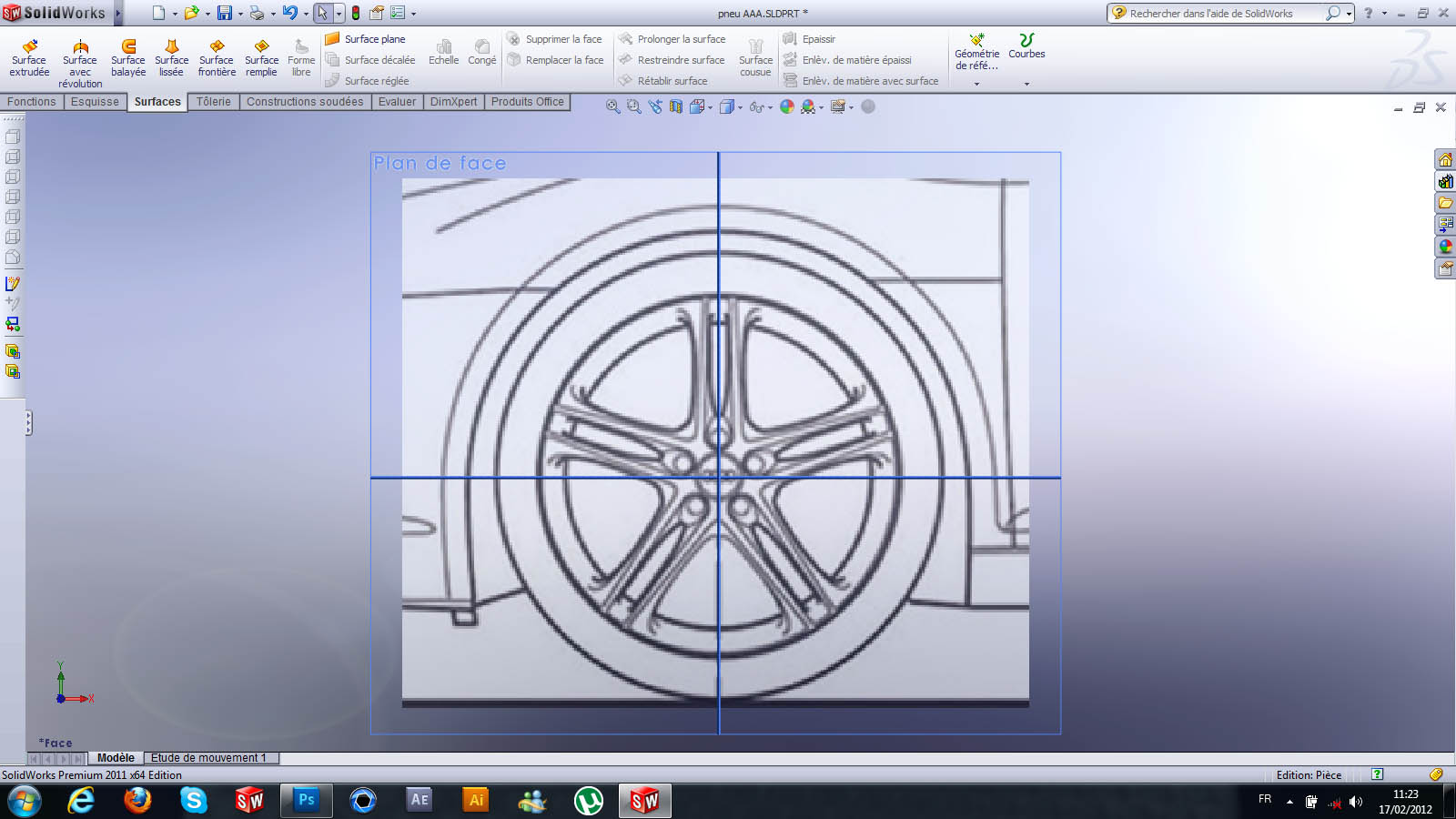2. ### Step 2:

you draw the various sketches that I need and I am an extrusion part cerculaire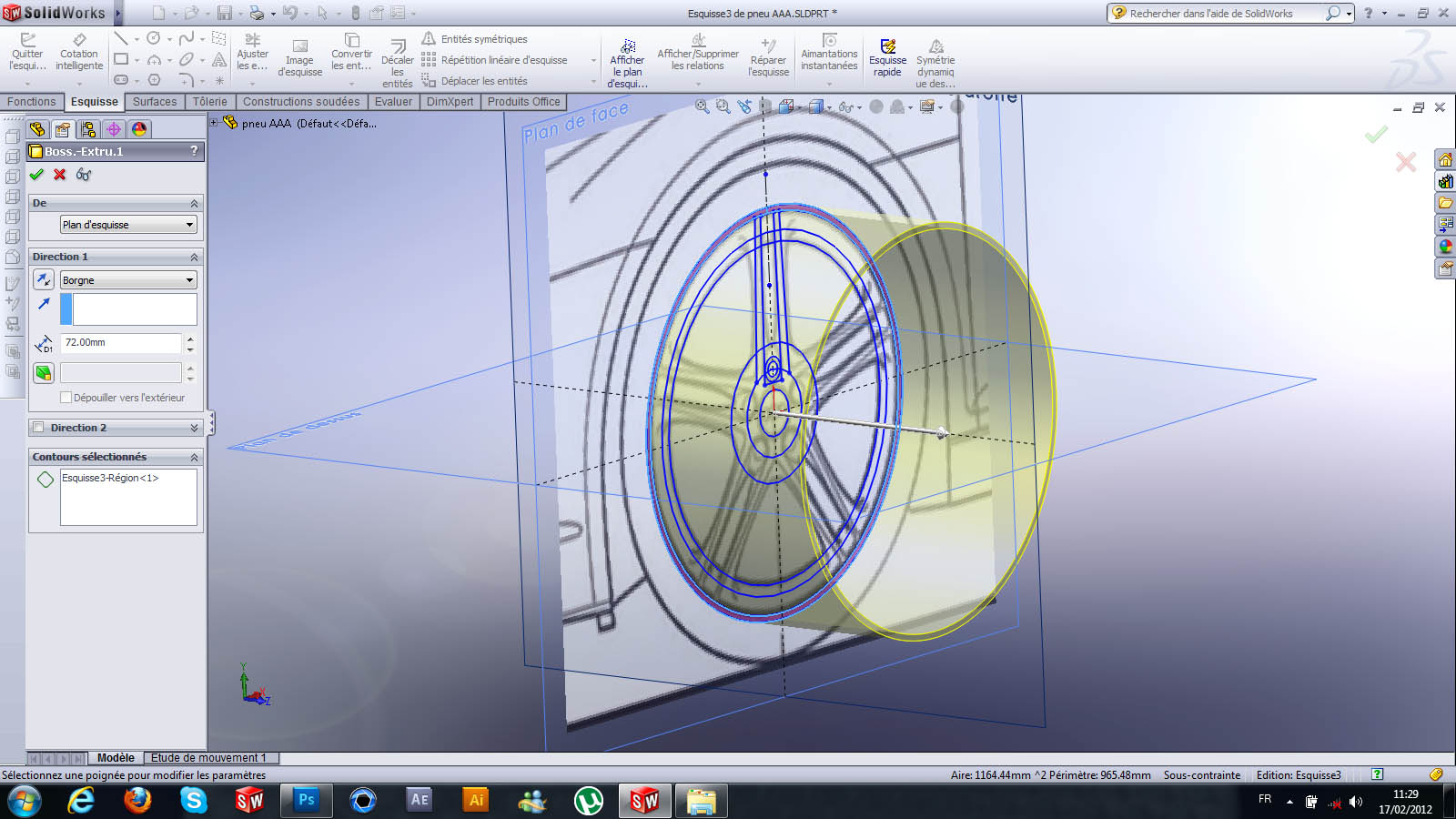3. ### Step 3:

here is the result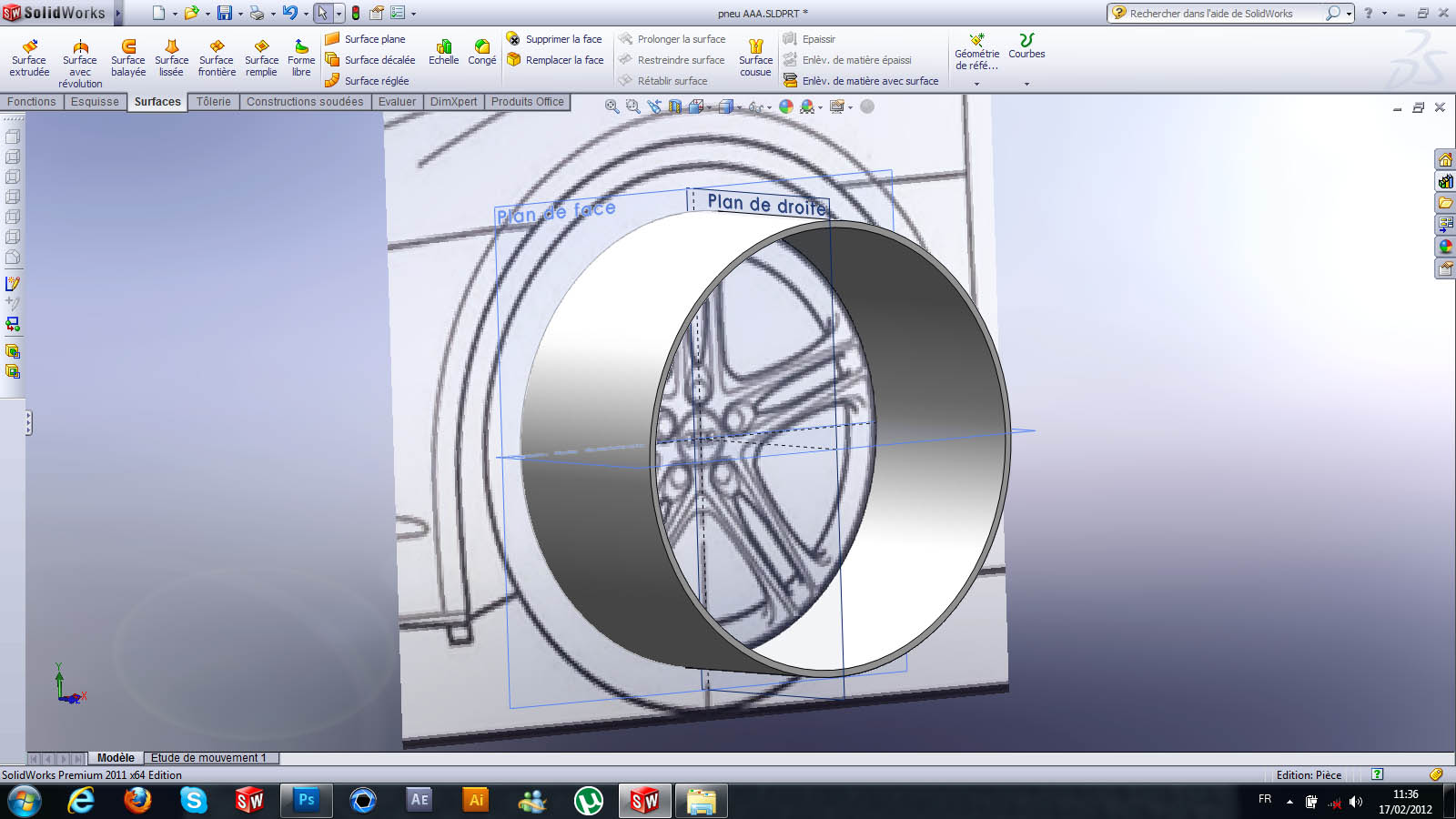4. ### Step 4:

you draw a sketch on the right plan with a construction line ;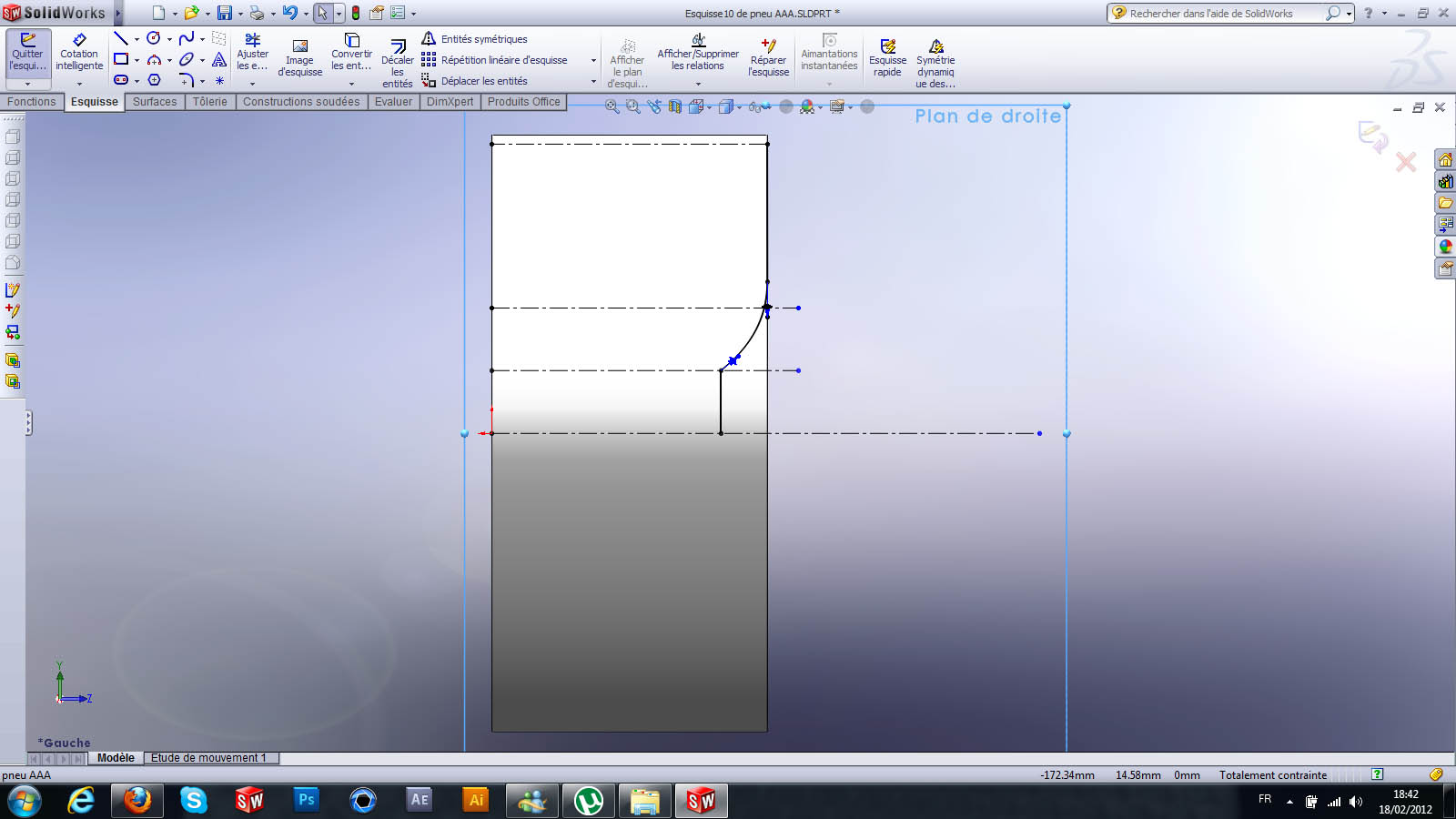5. ### Step 5:

I make a circular surface along the line construction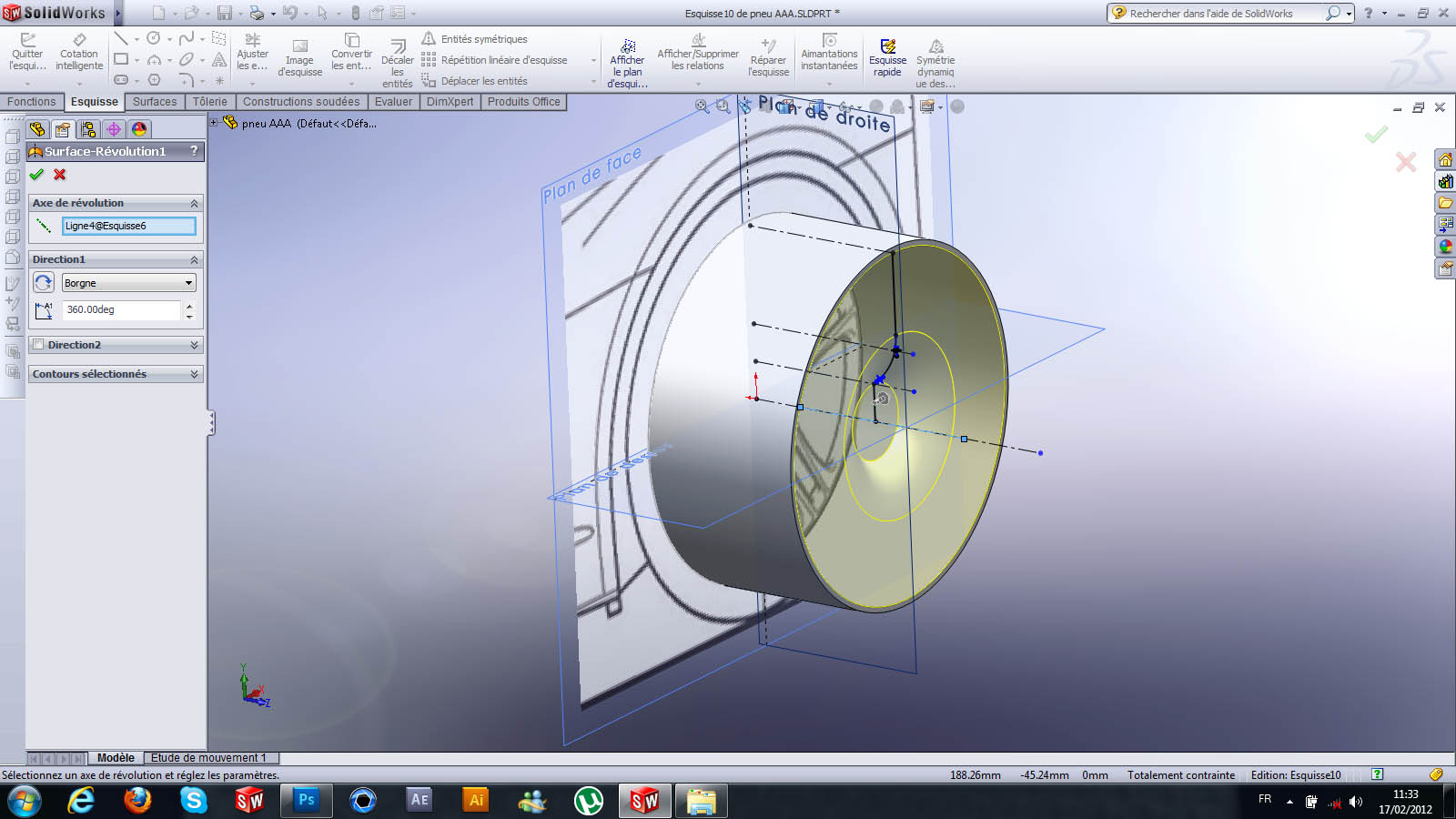6. ### Step 6:

here is the result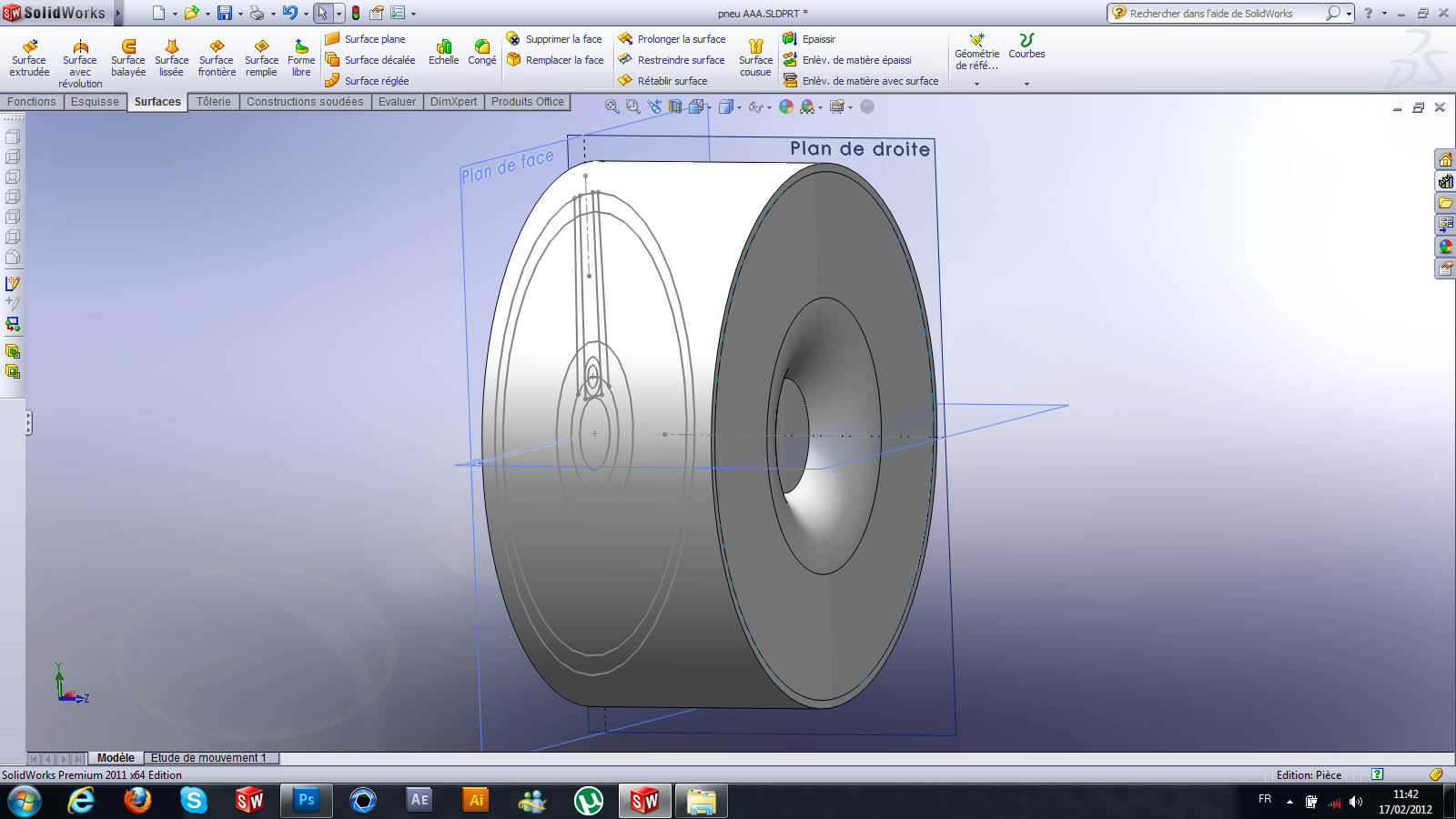7. ### Step 7:

you create a sketch on the right plan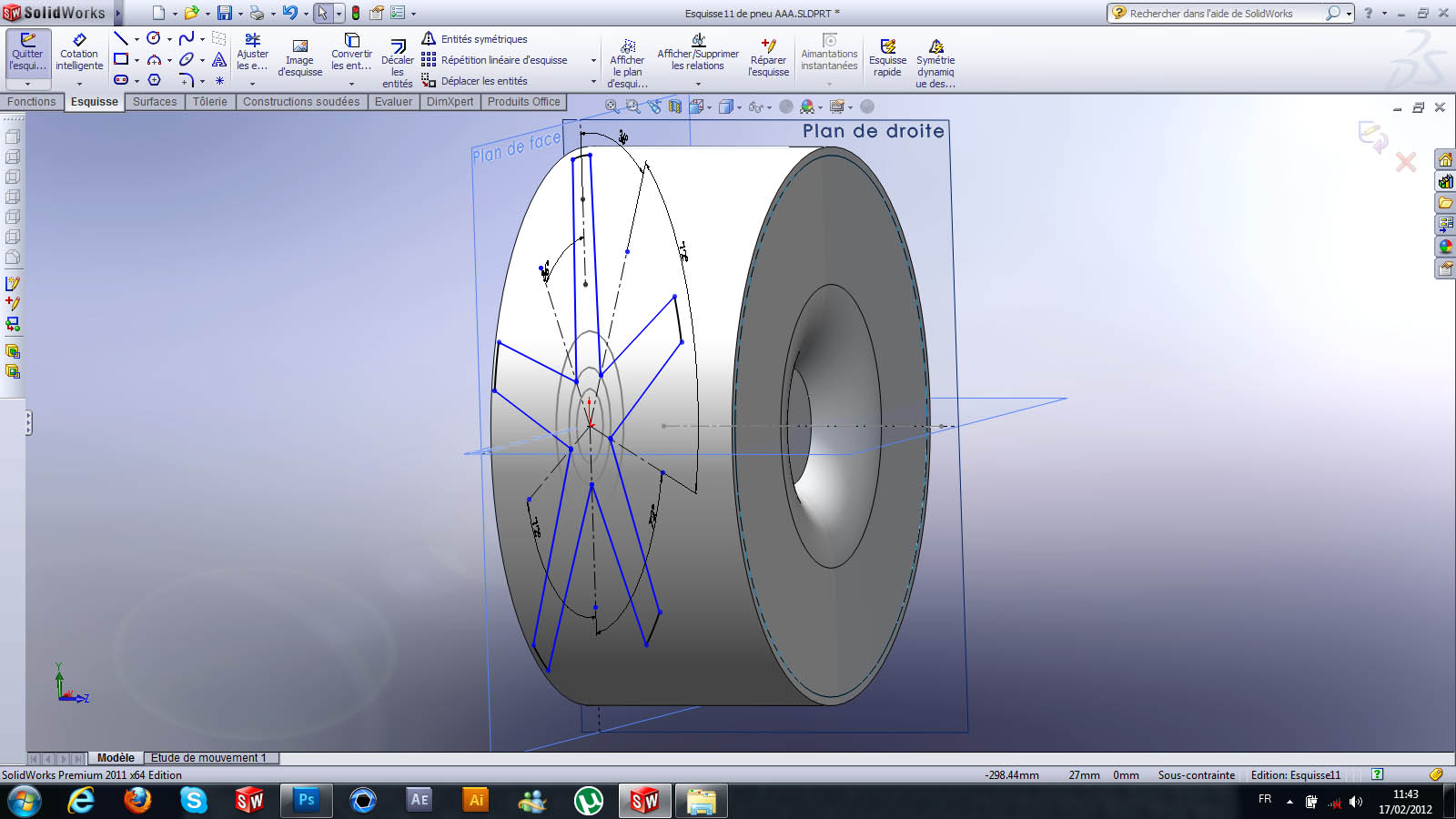8. ### Step 8:

you create an extrusion to the surface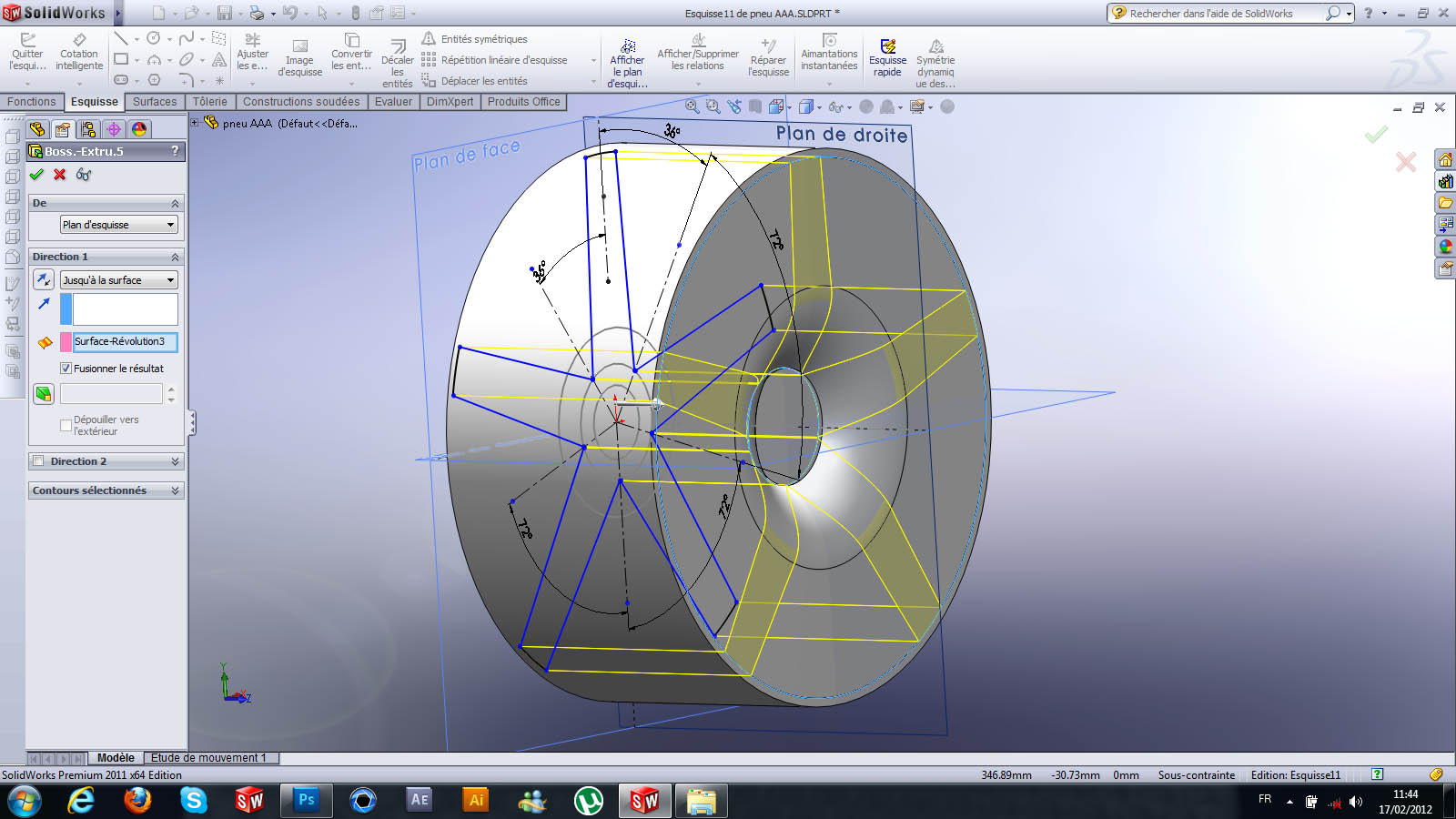9. ### Step 9:

You can hide the face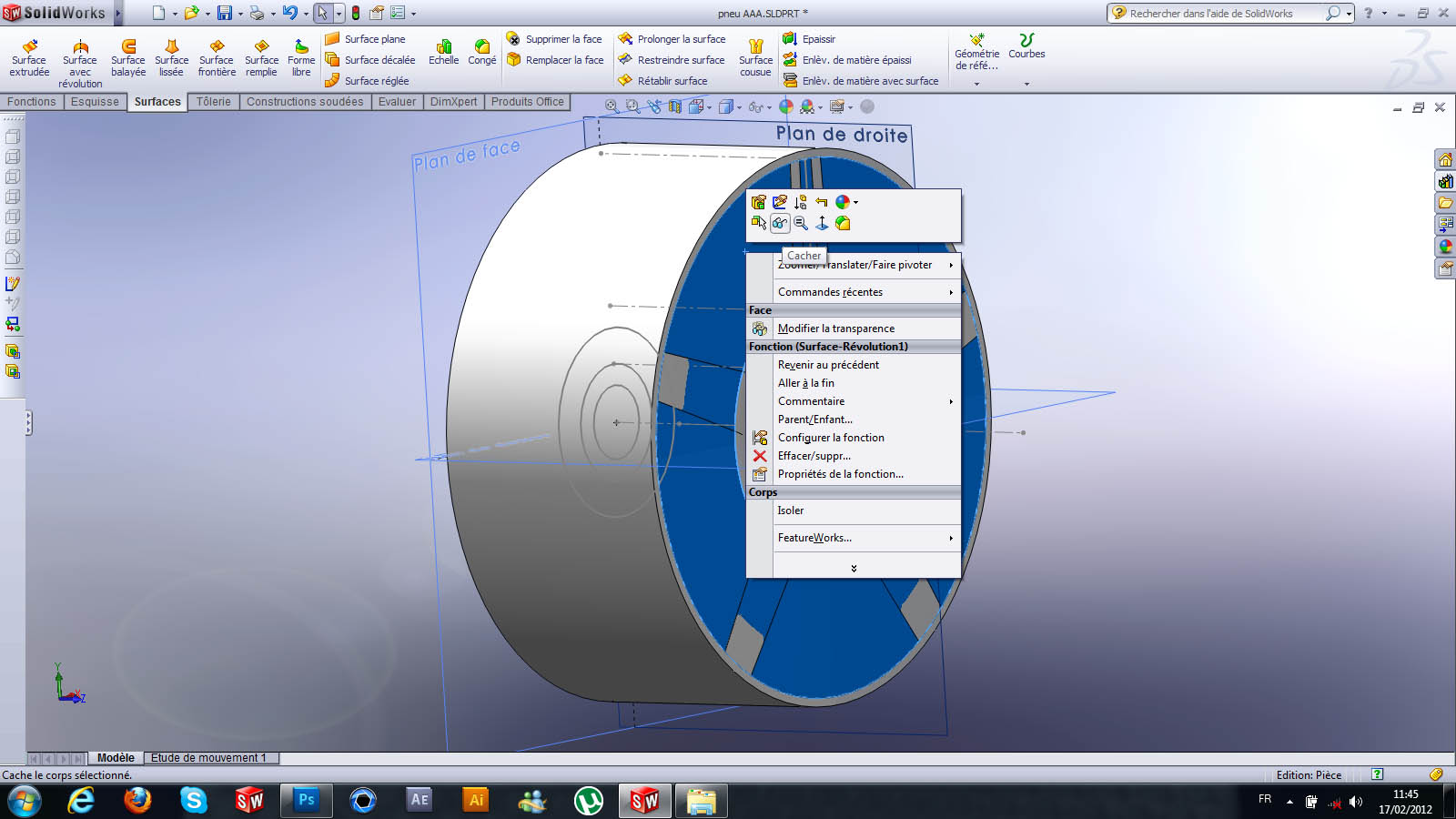10. ### Step 10:

here is the result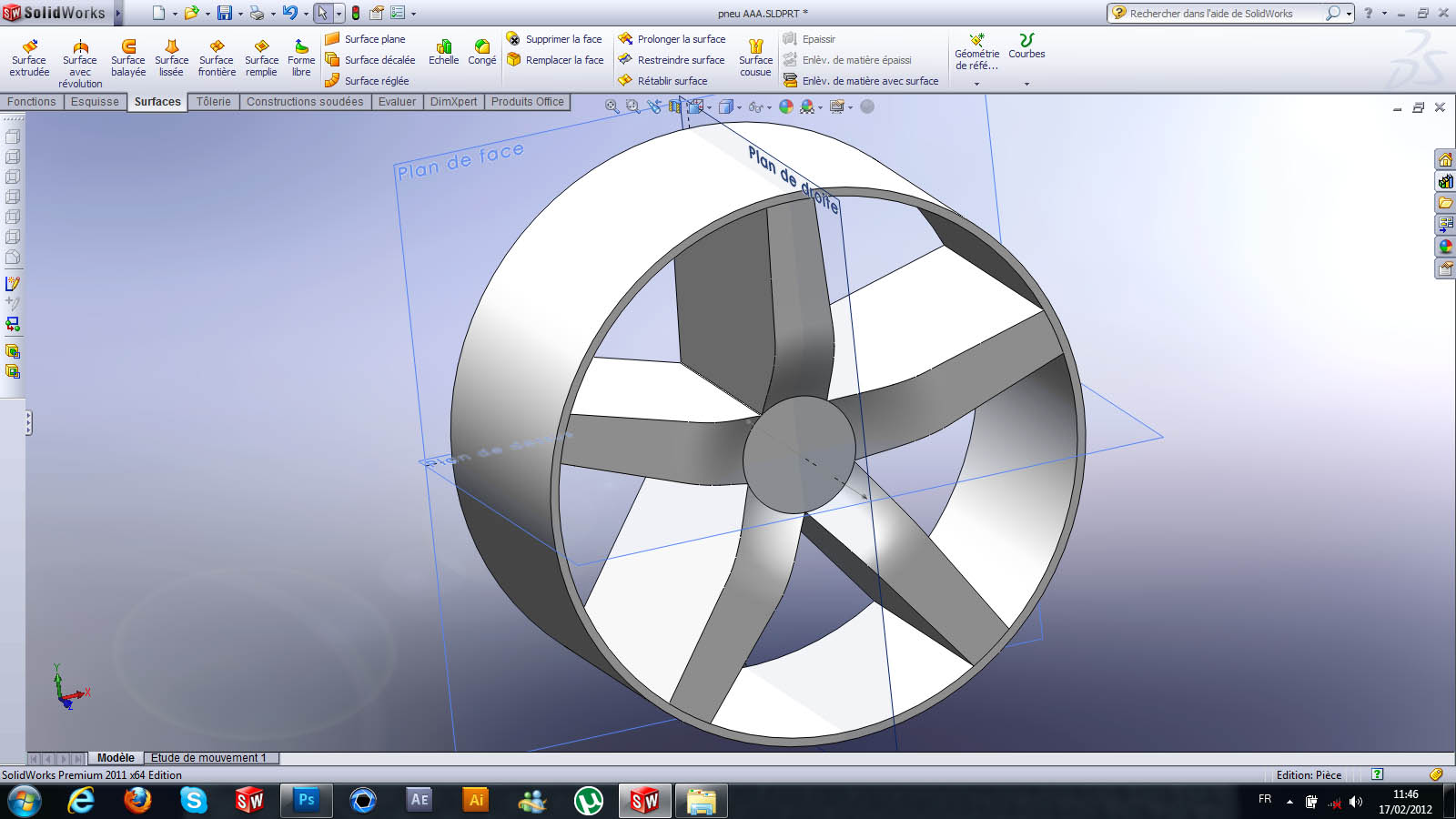11. ### Step 11:

you create a sketch on the front like in the picture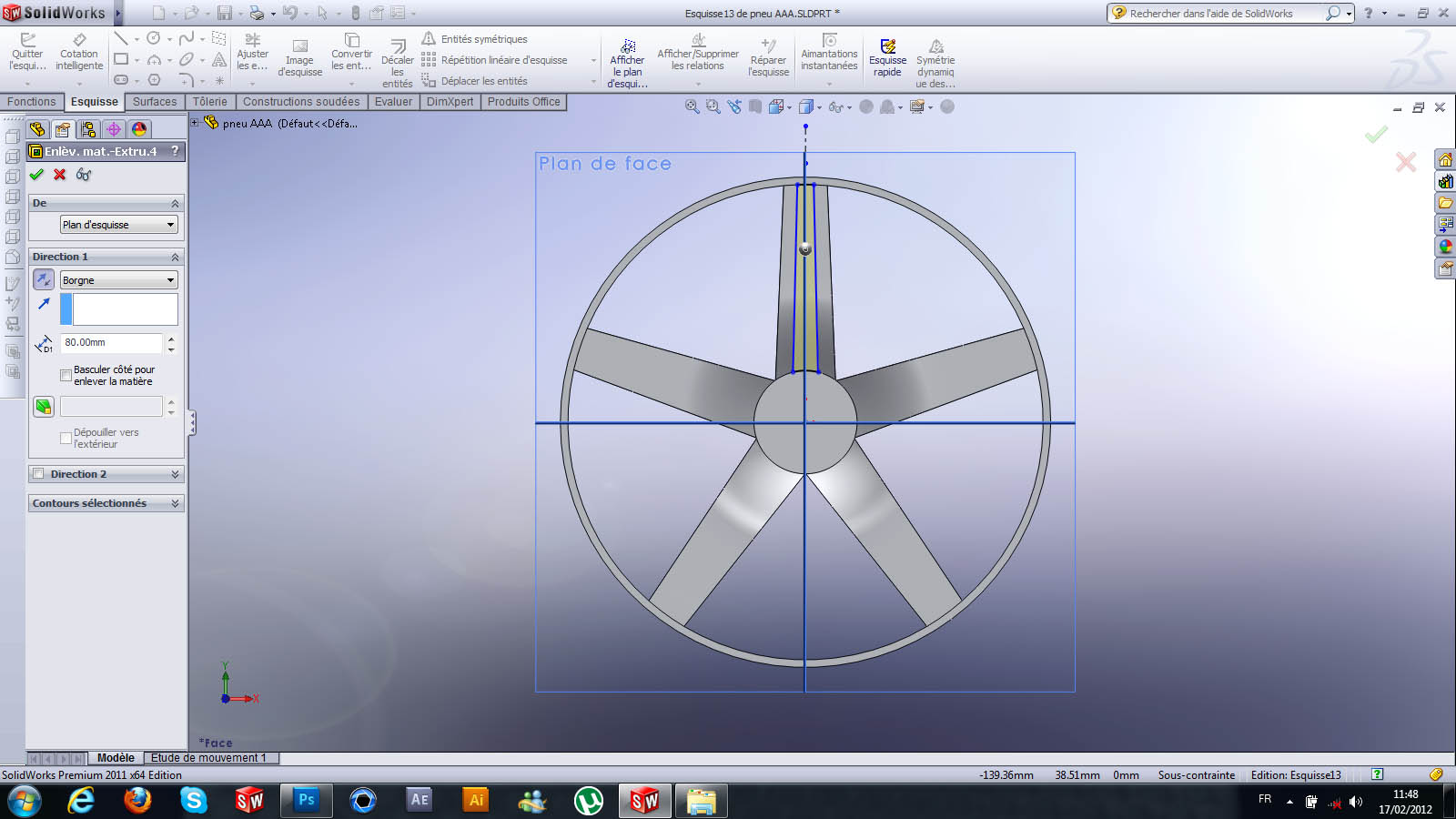12. ### Step 12:

here is the result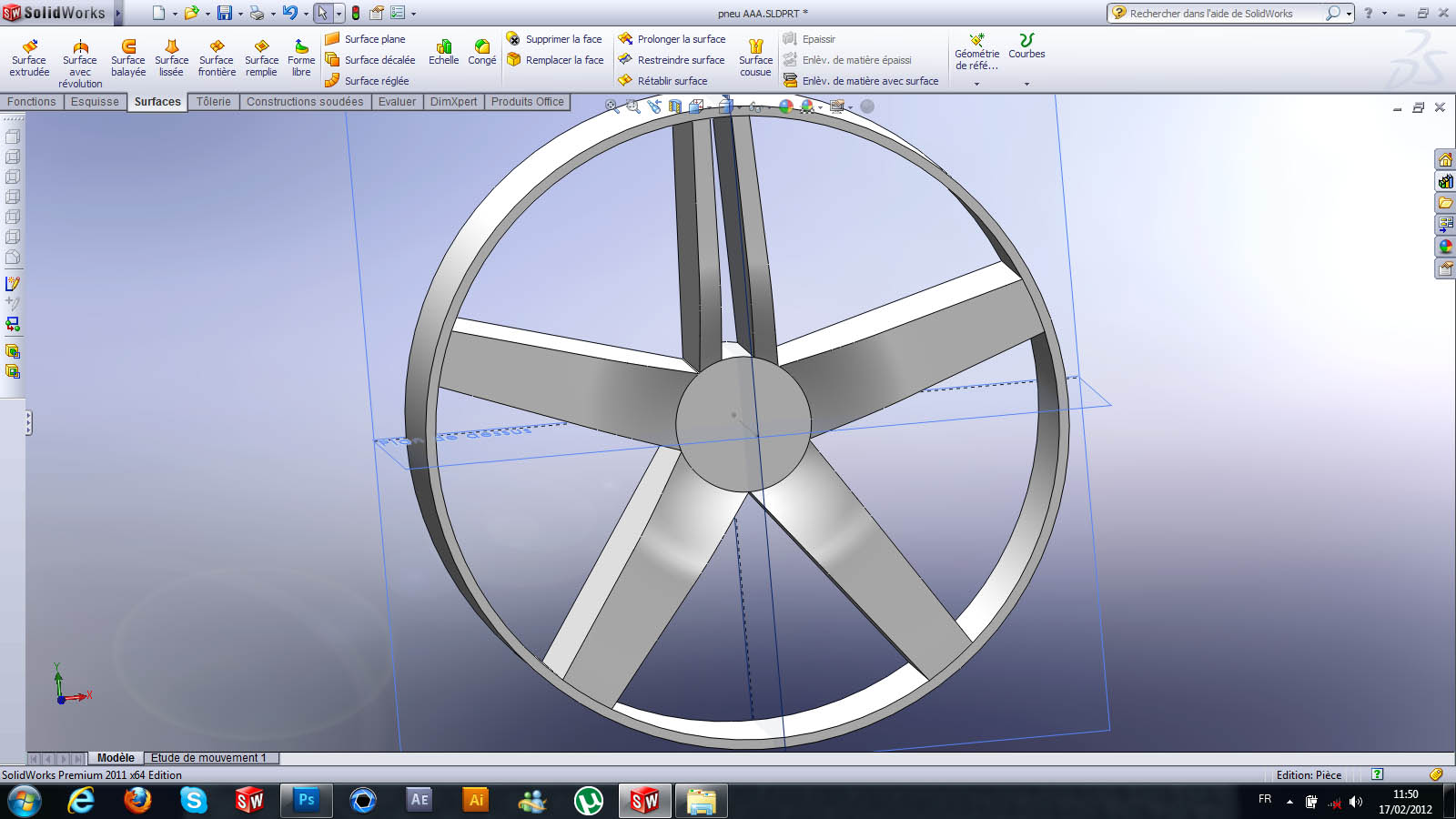13. ### Step 13:

and you make a circular repetition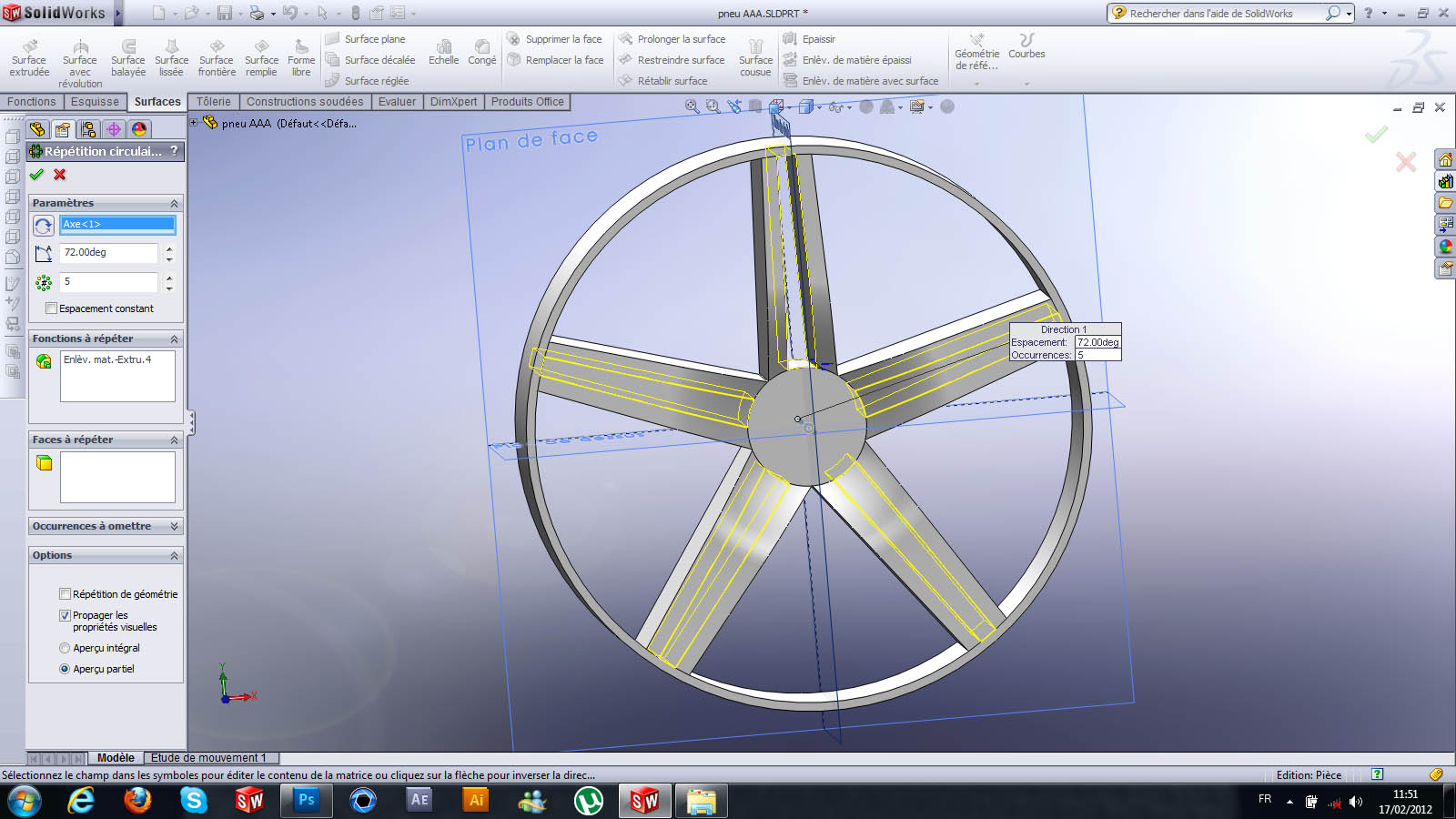14. ### Step 14:

here is the result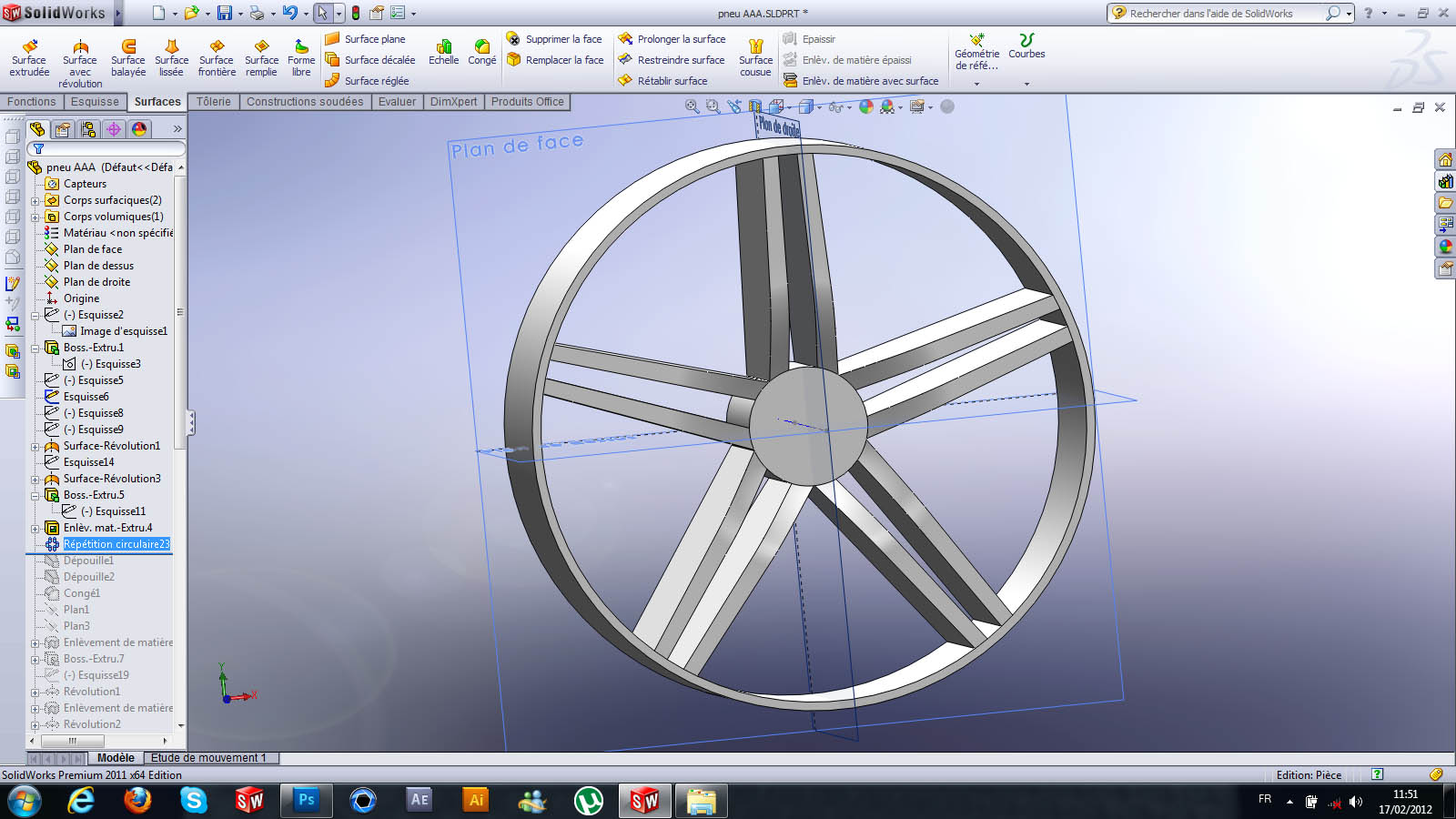15. ### Step 15:

you make a body on the exterior surface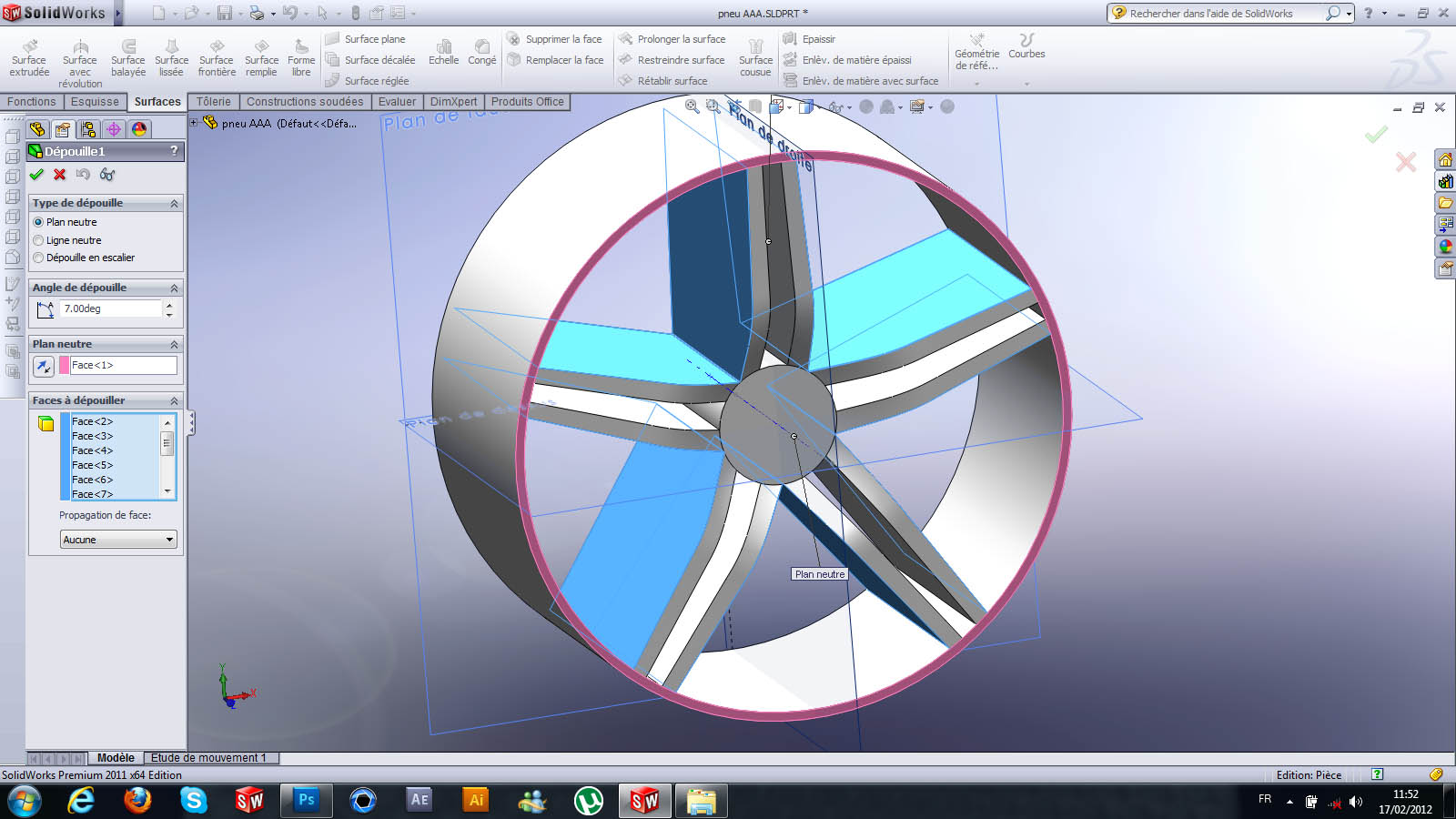16. ### Step 16:

here is the result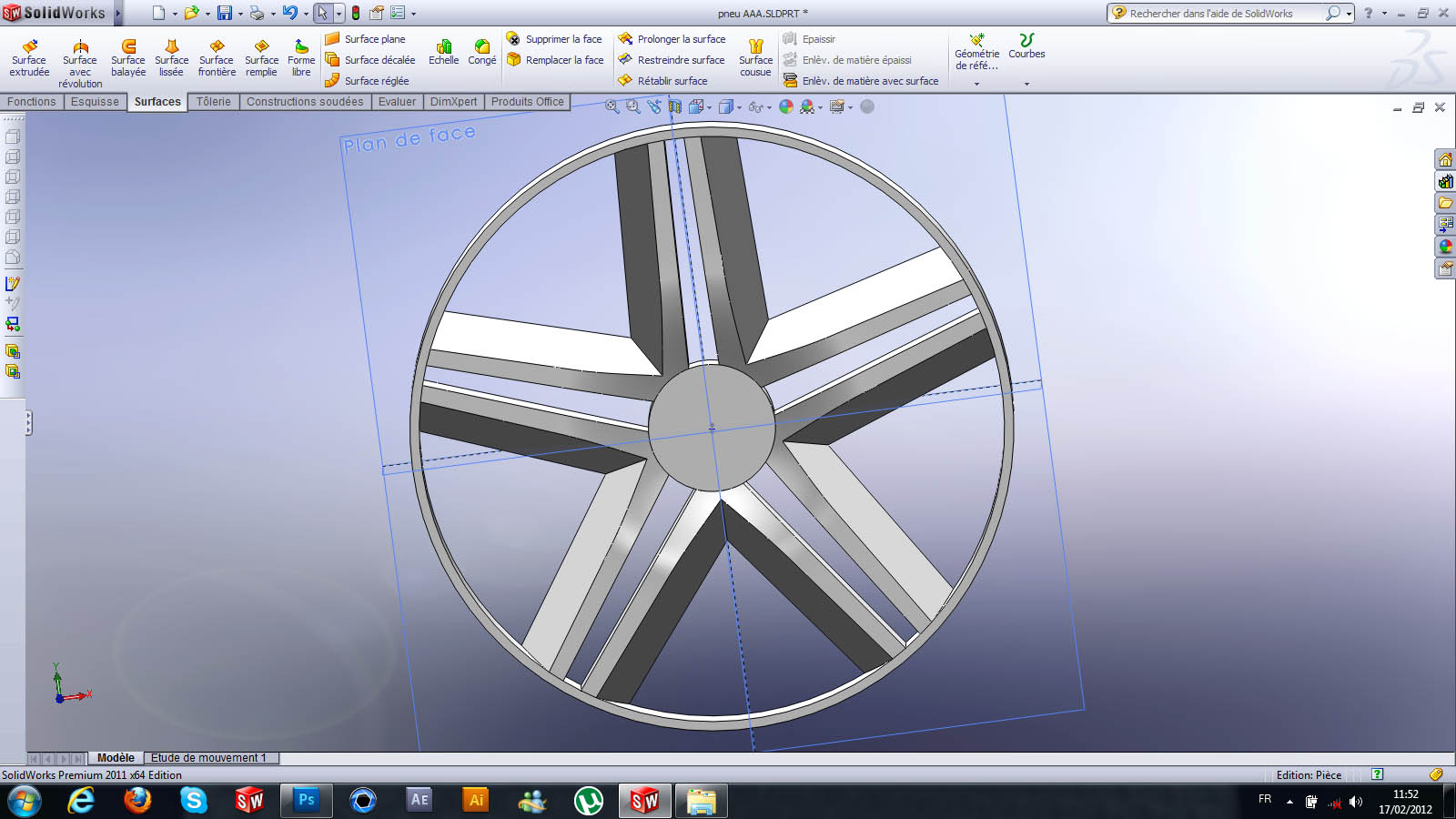17. ### Step 17:

you do the same for the interior face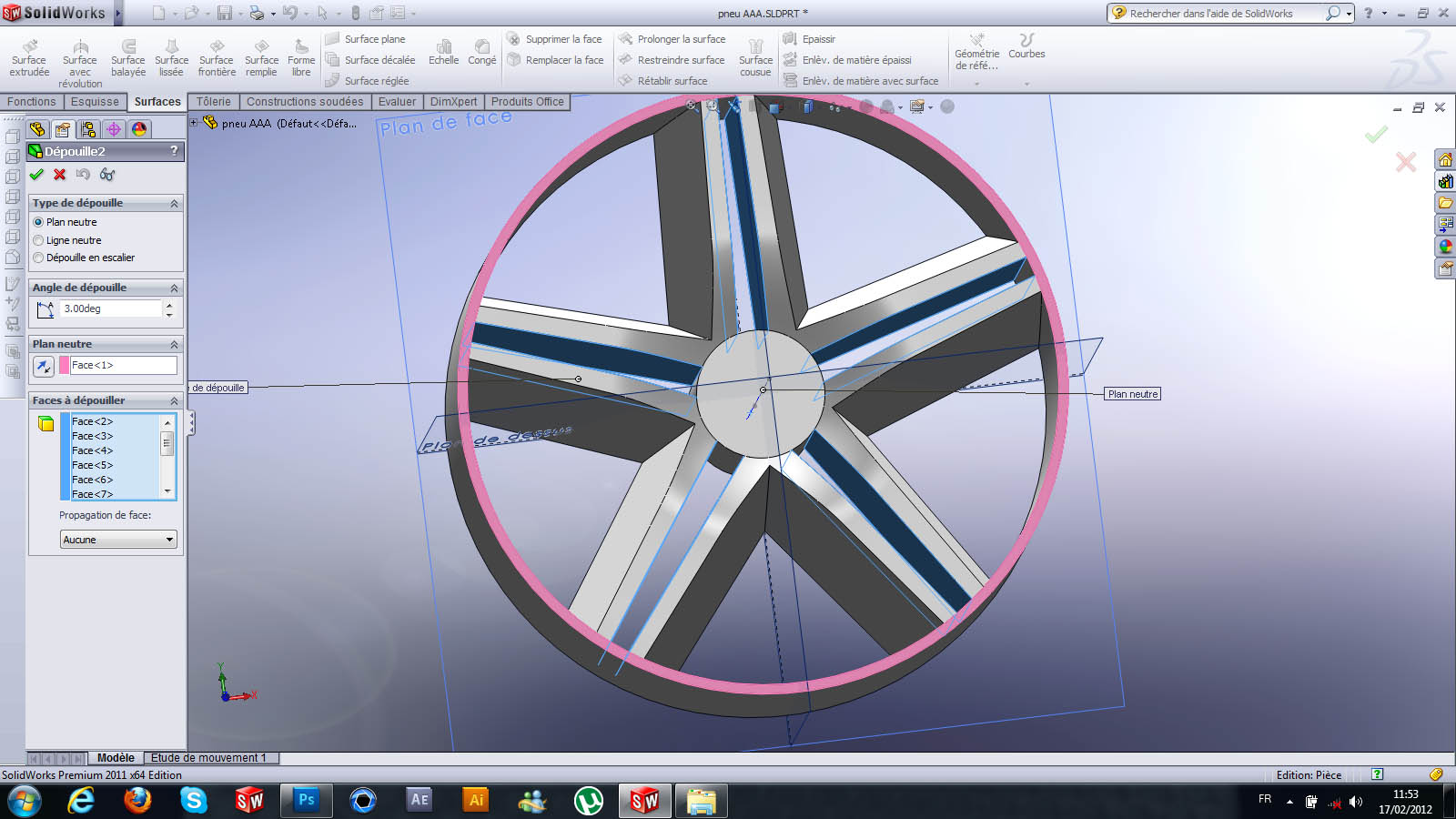18. ### Step 18:

here is the result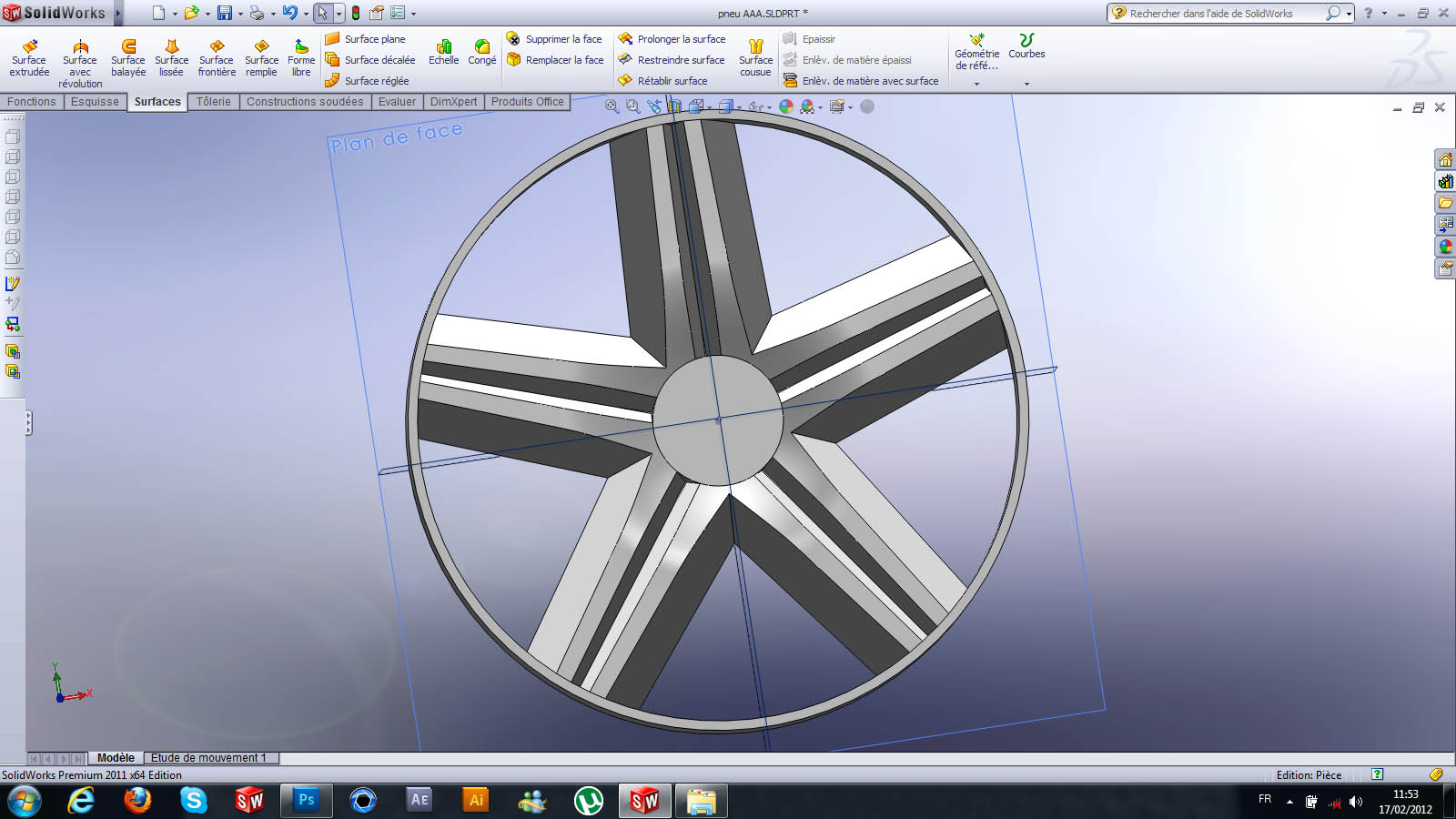19. ### Step 19:

after you leave the edges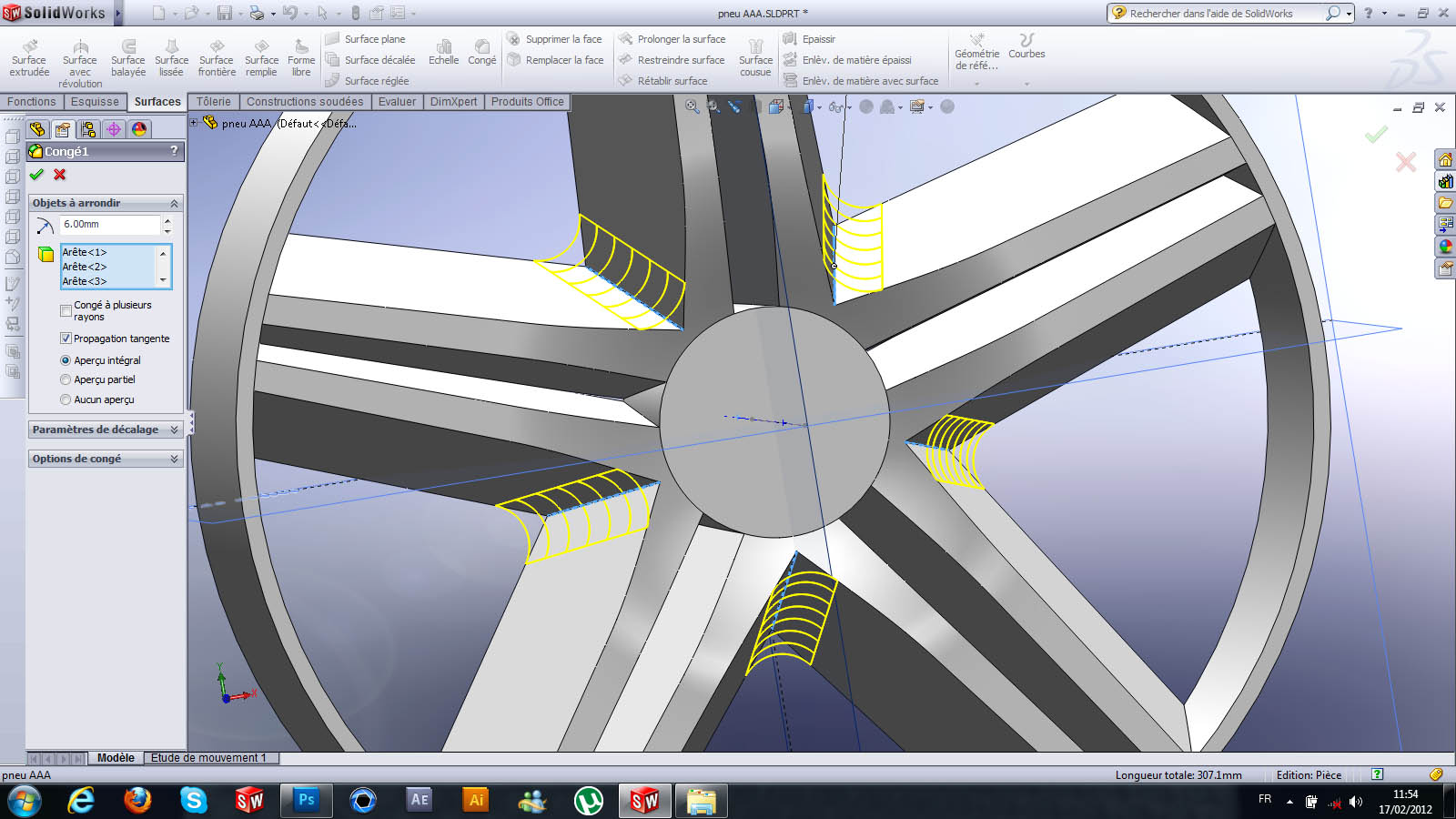20. ### Step 20:

you draw a sketch on the right plan with a construction line to the removal of the material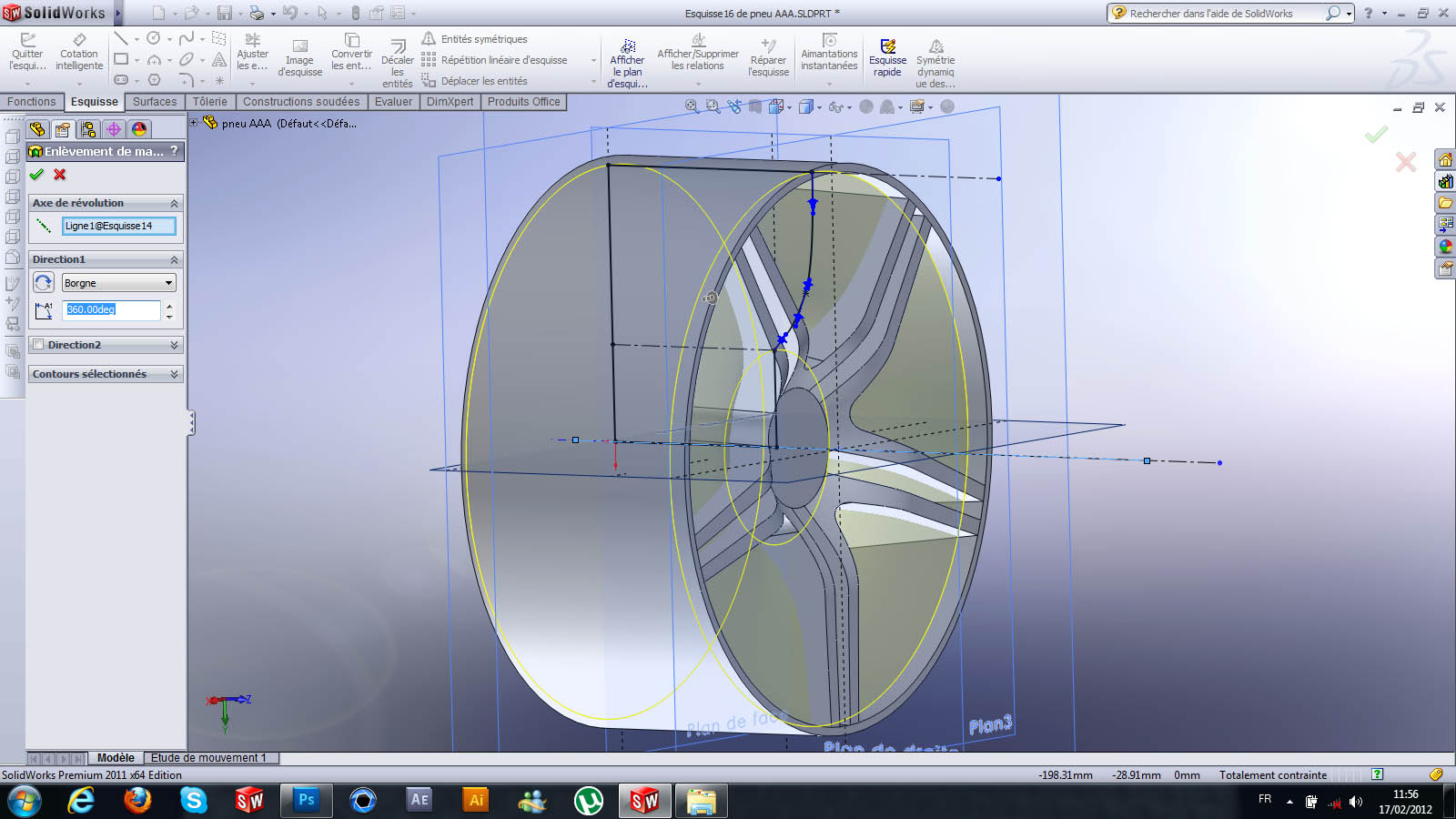21. ### Step 21:

here is the result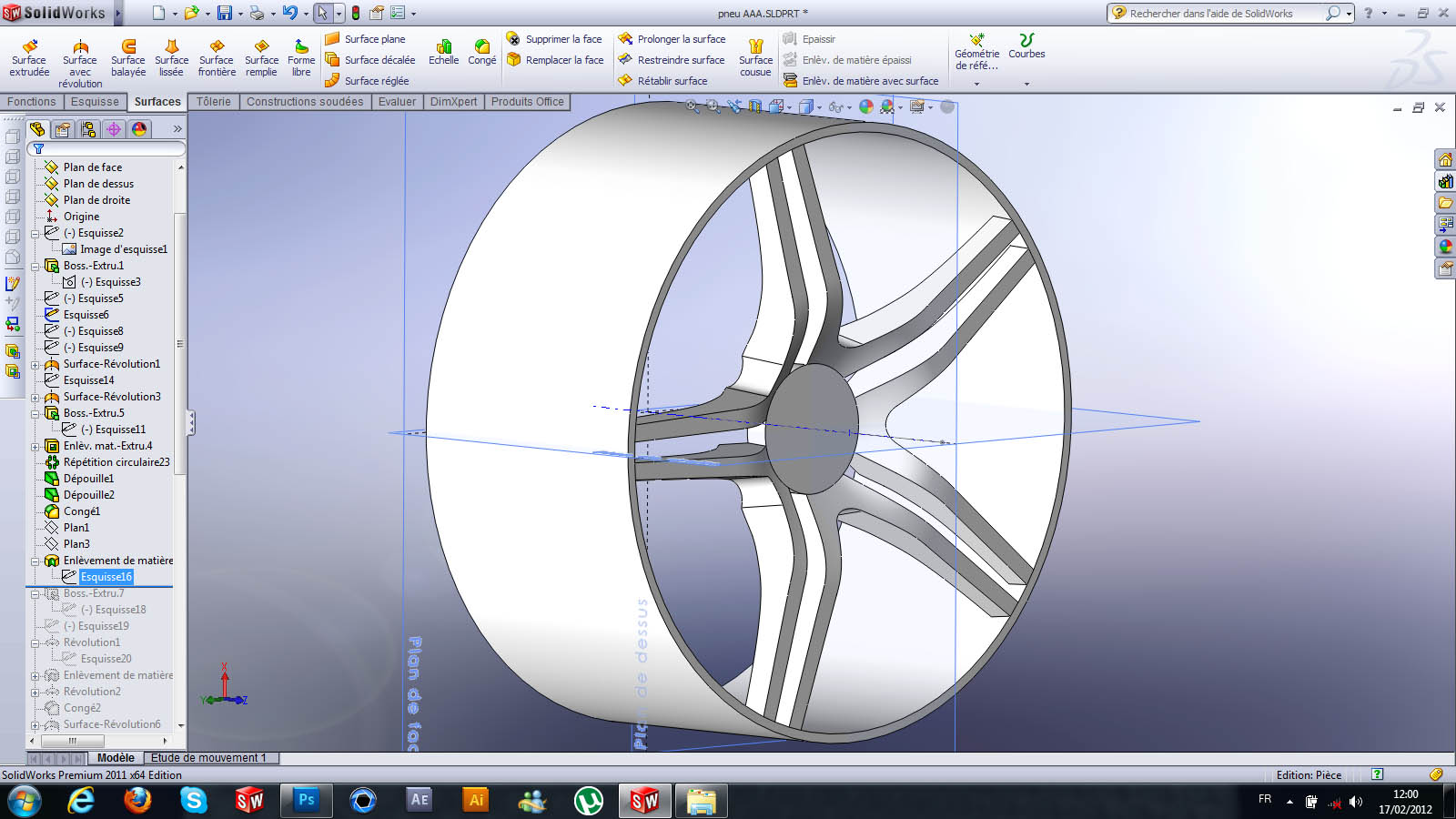22. ### Step 22:

you draw a sketch on the front plan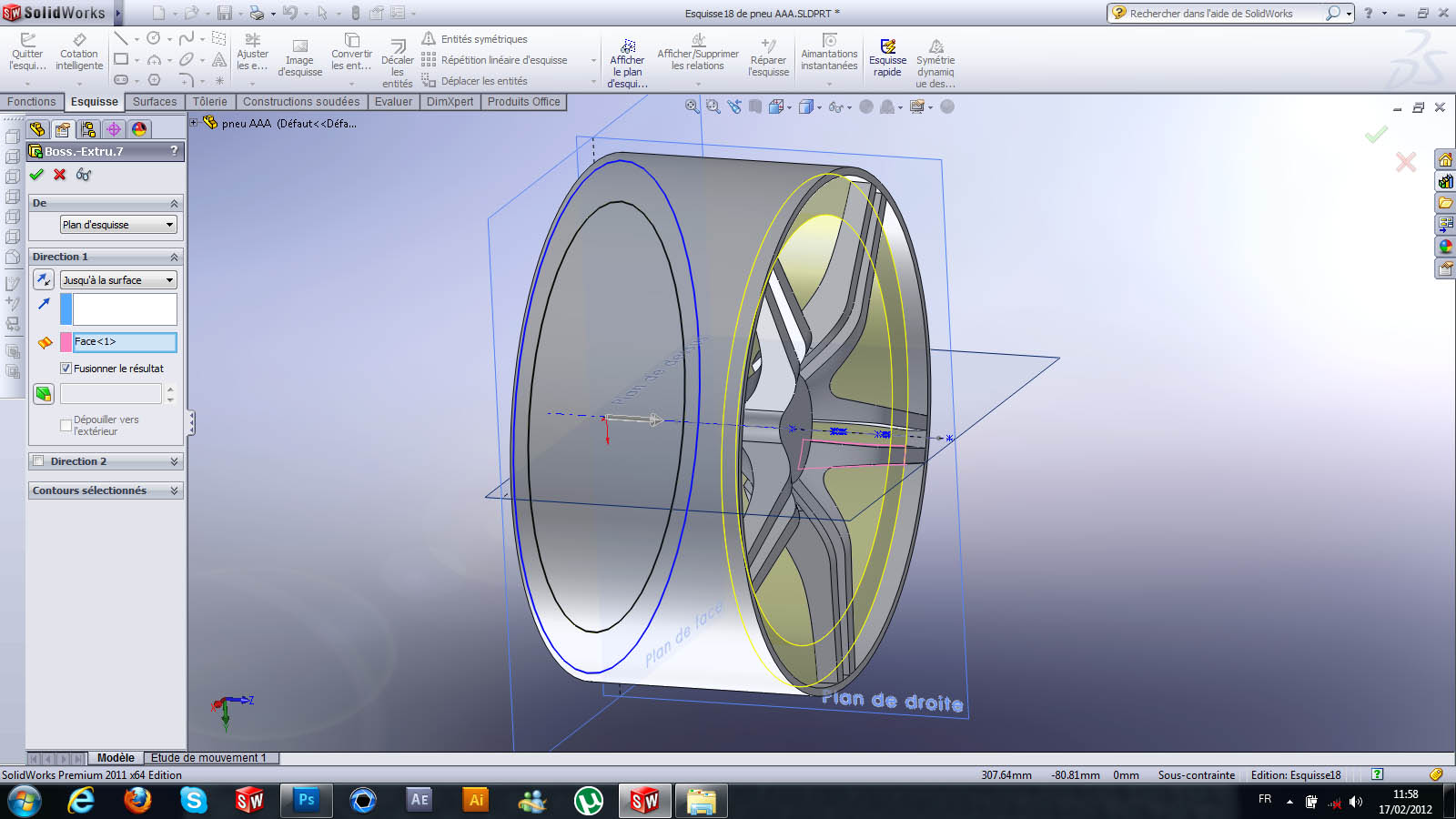23. ### Step 23:

you draw a sketch on the front to have a spherical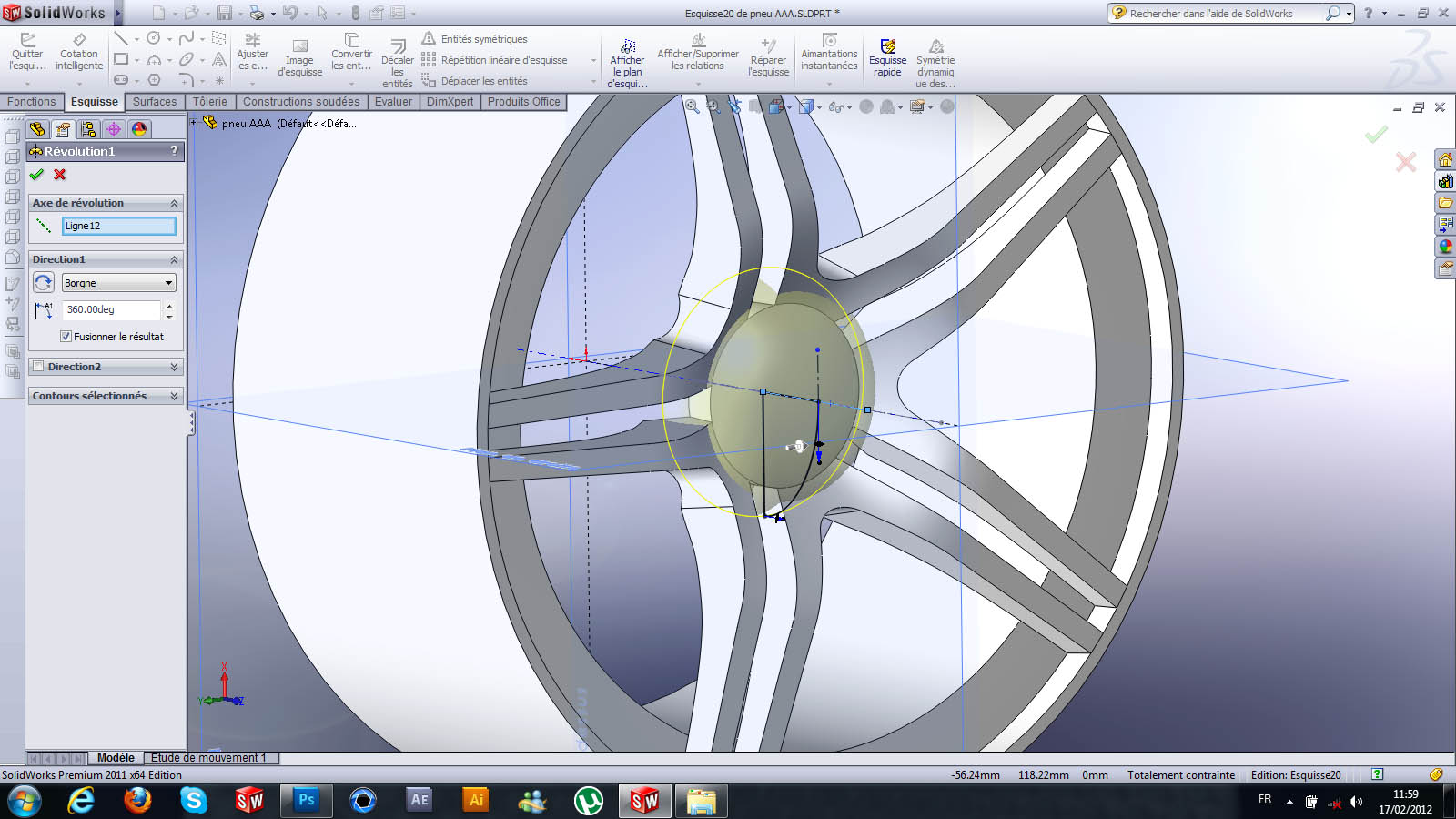24. ### Step 24:

.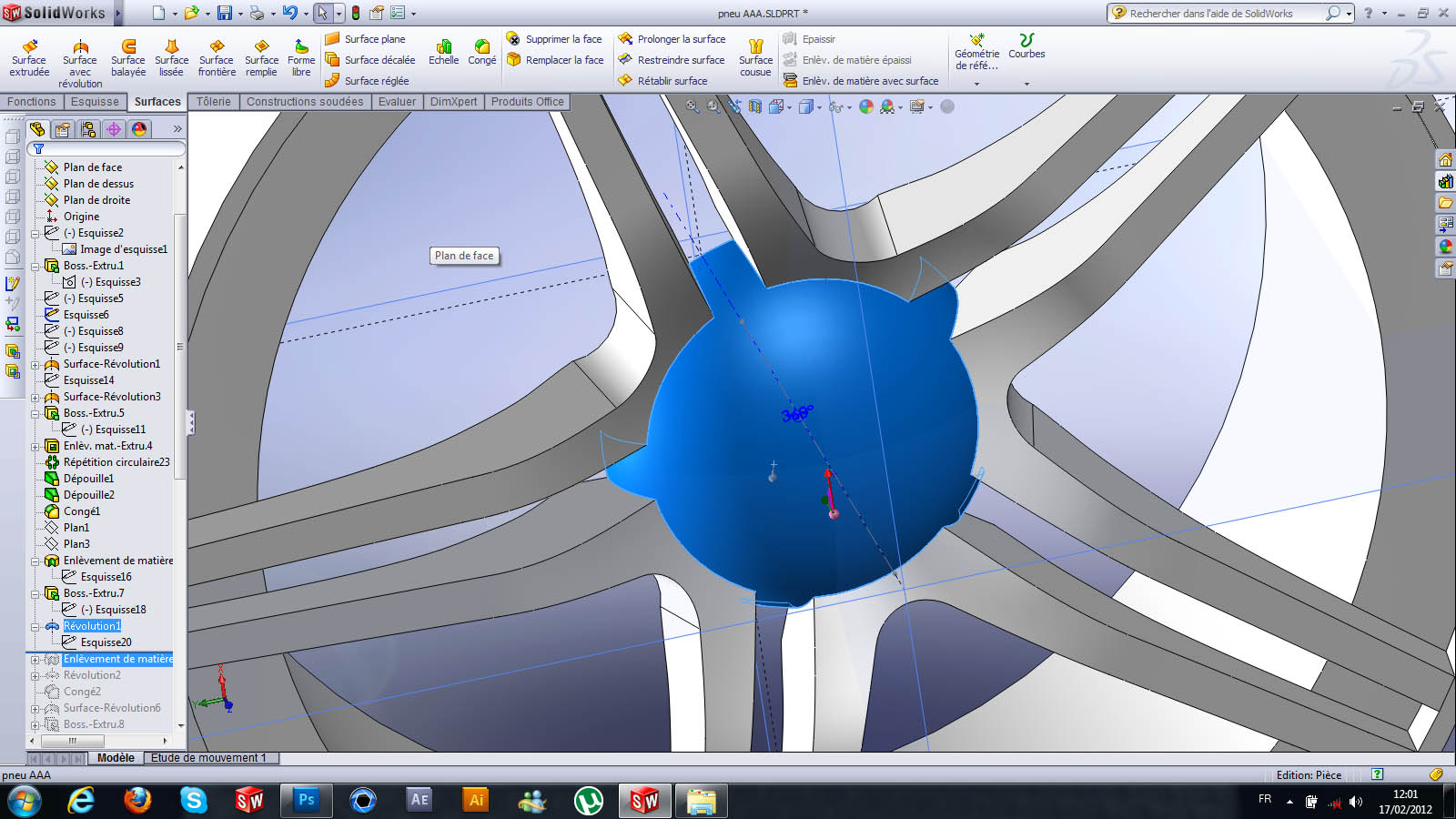25. ### Step 25:

.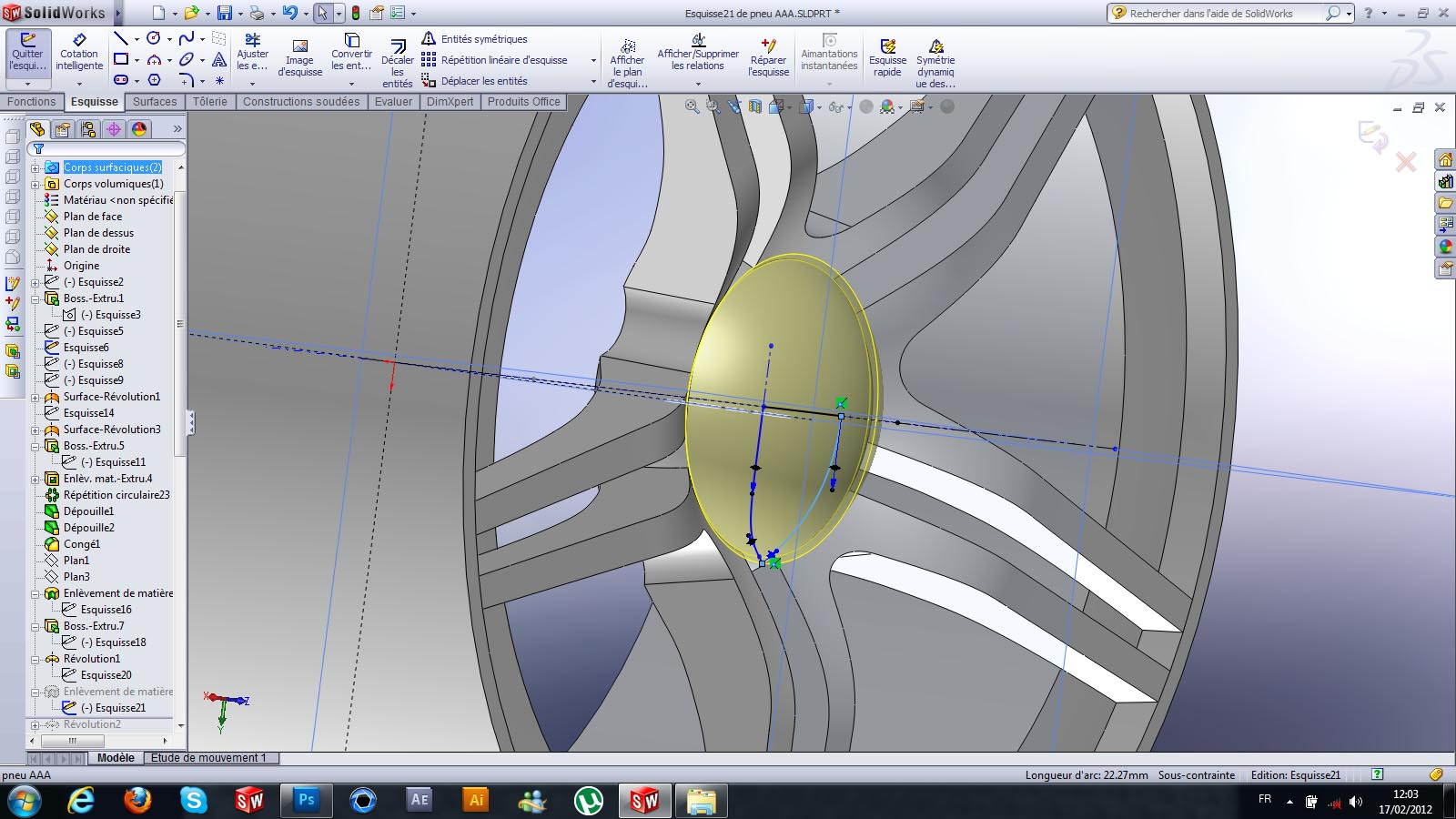26. ### Step 26:

.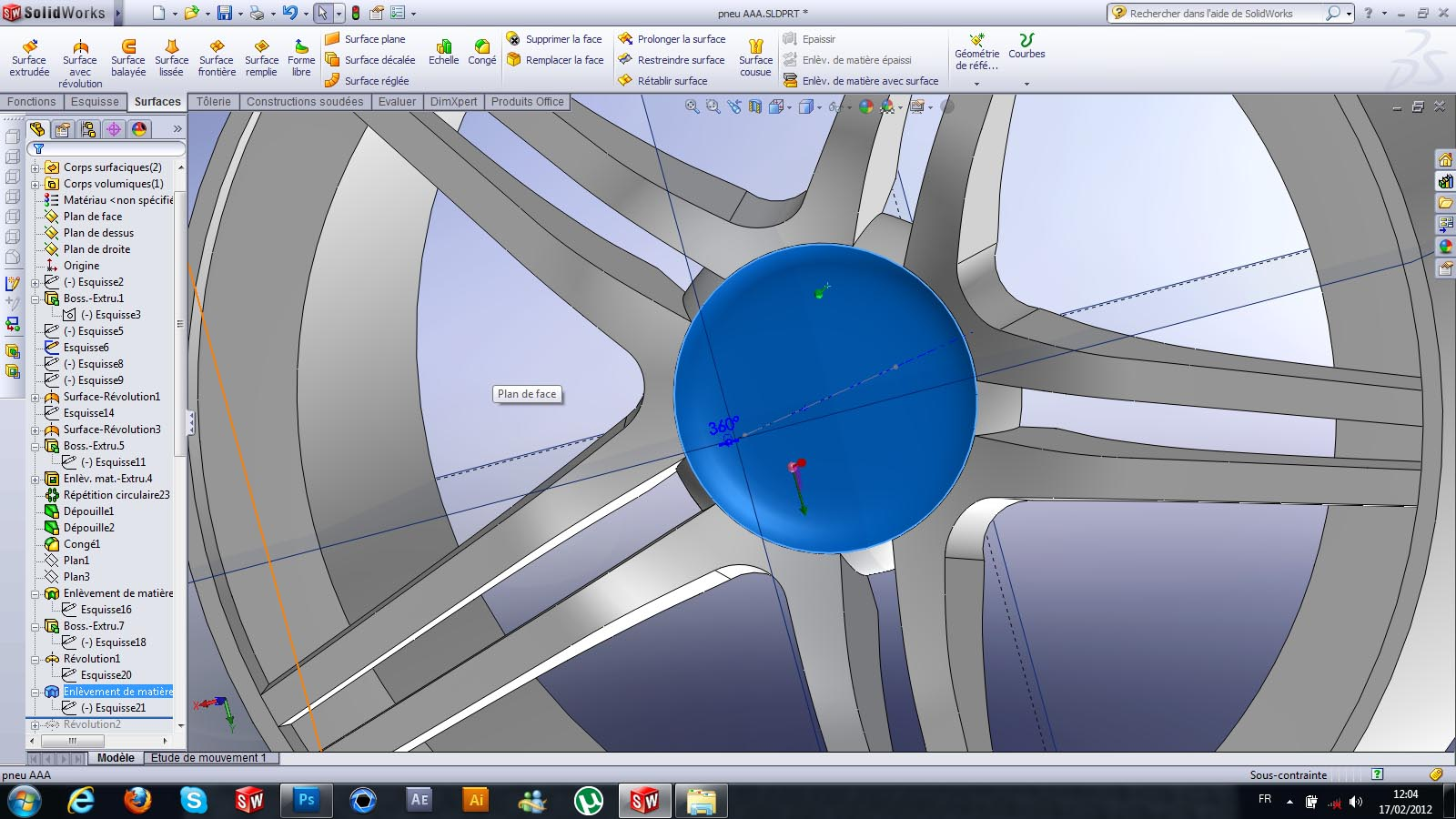27. ### Step 27:

.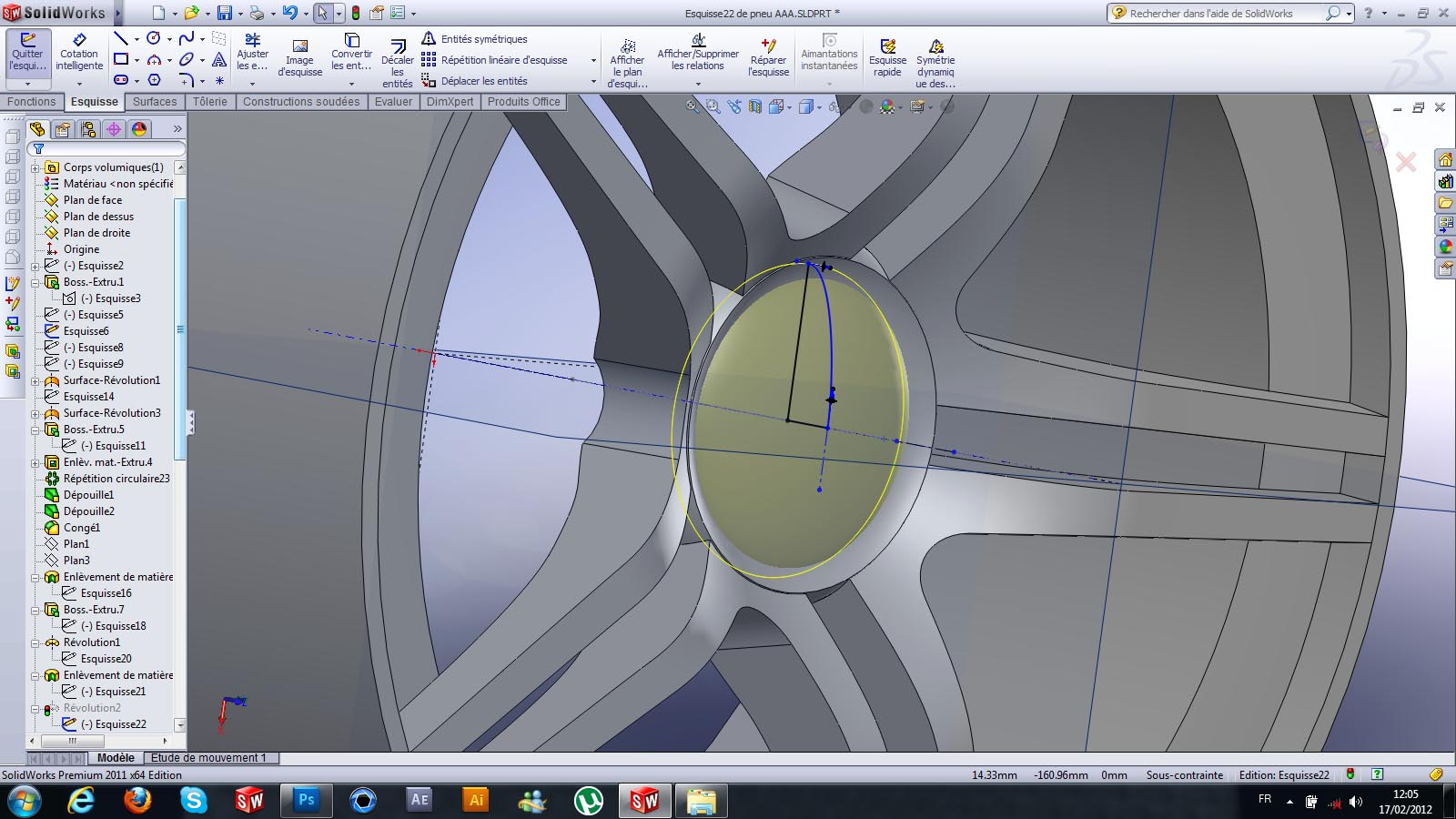28. ### Step 28:

..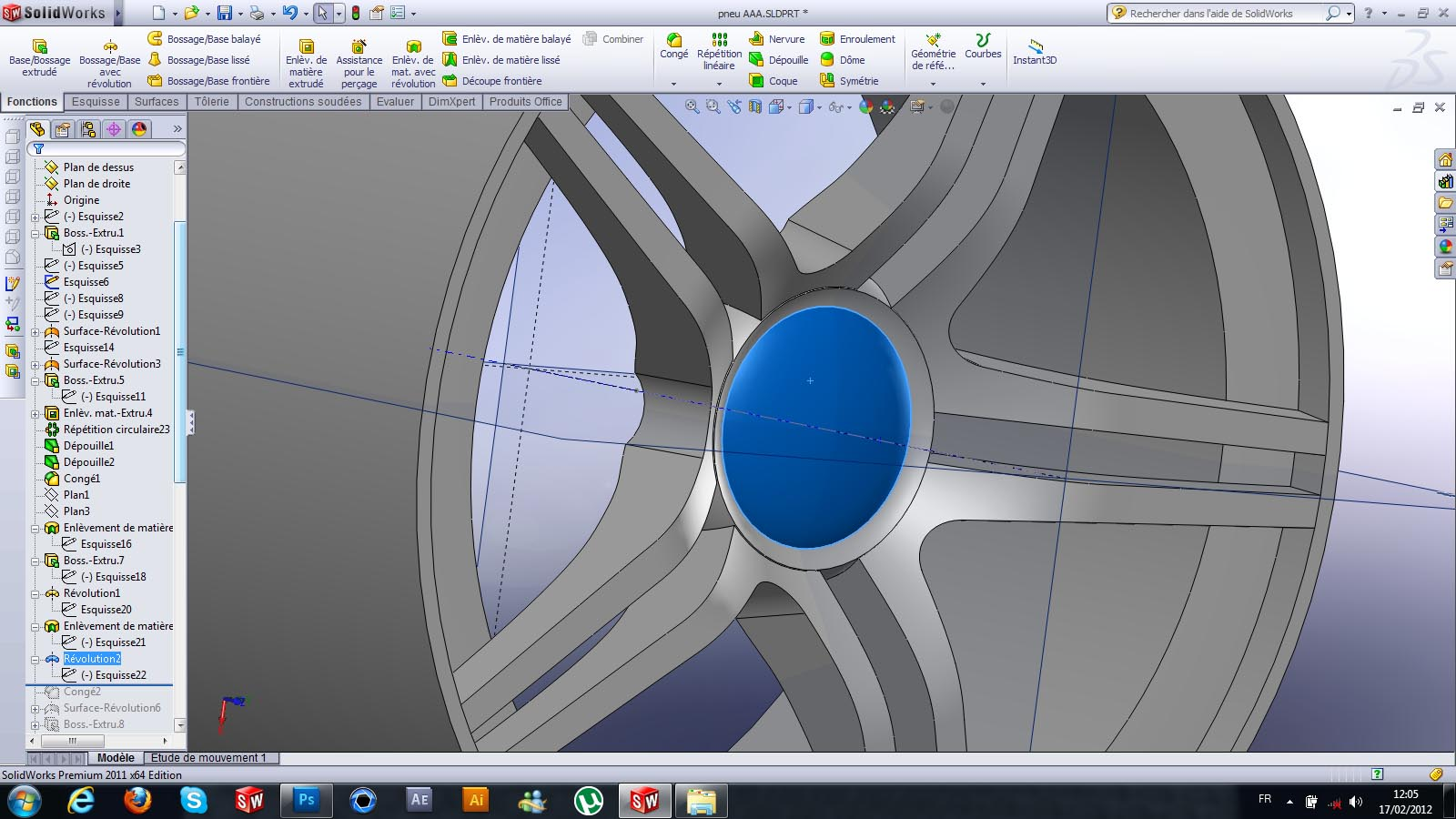29. ### Step 29:

.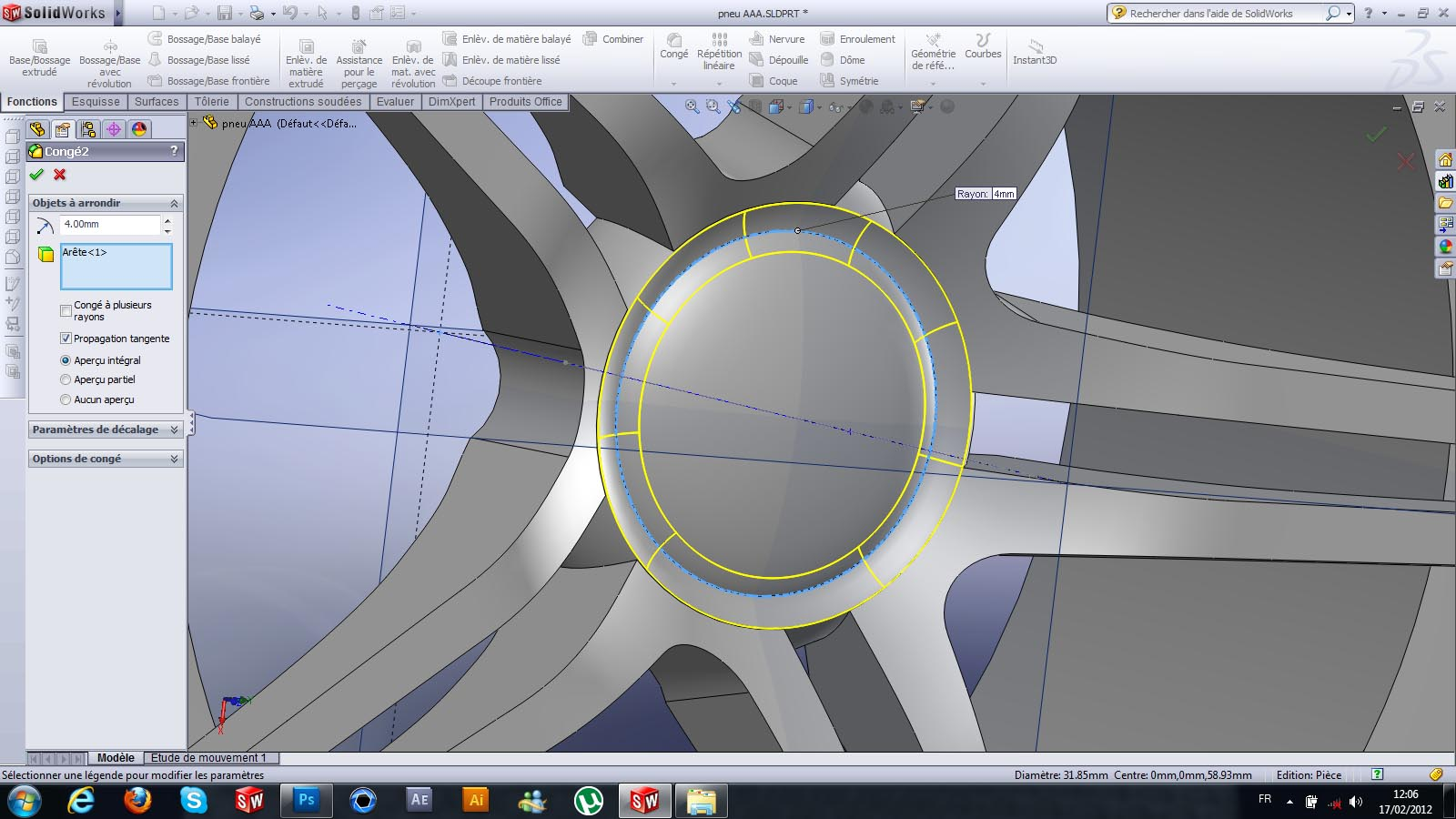30. ### Step 30:

.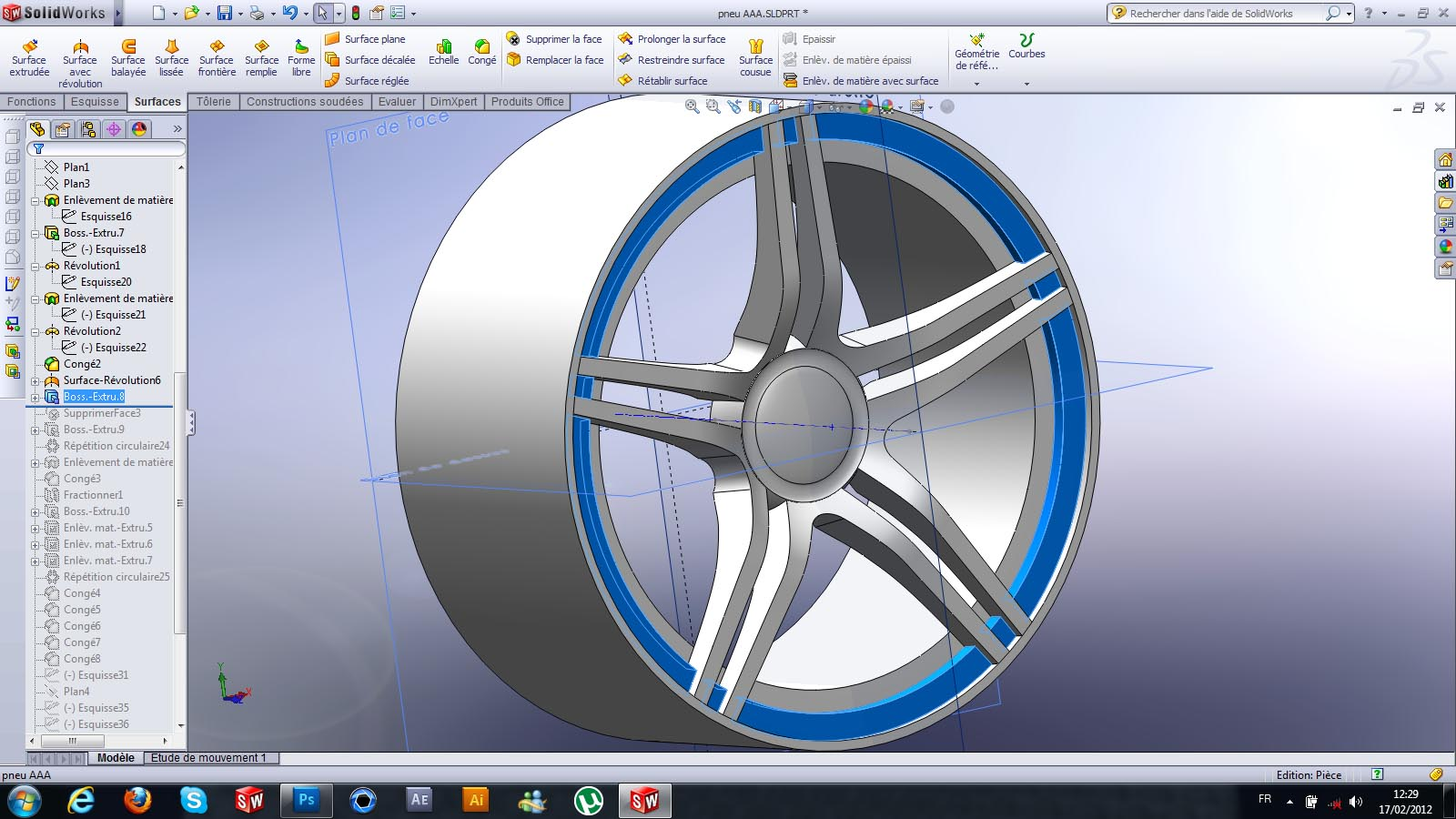31. ### Step 31:

.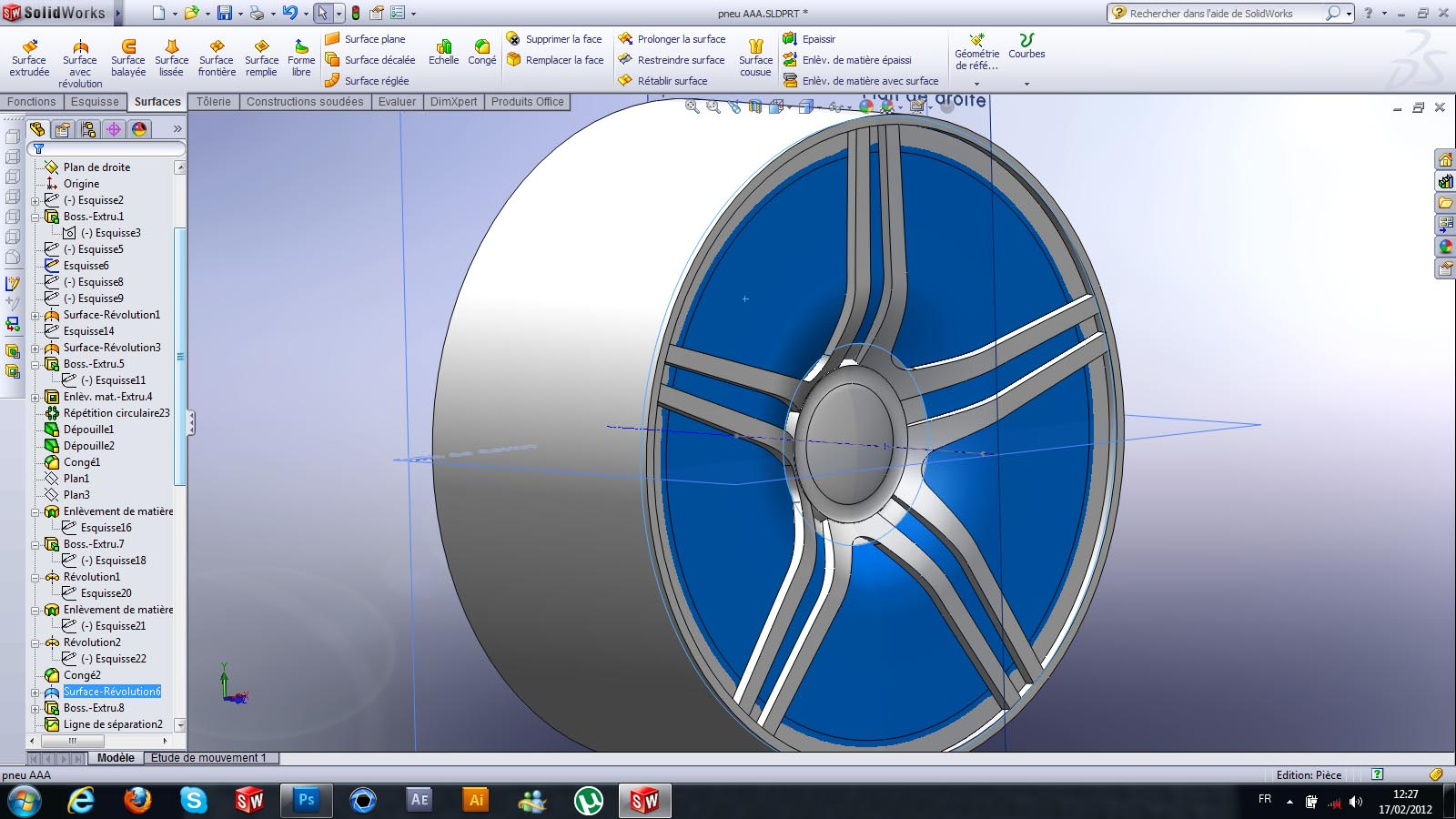32. ### Step 32:

.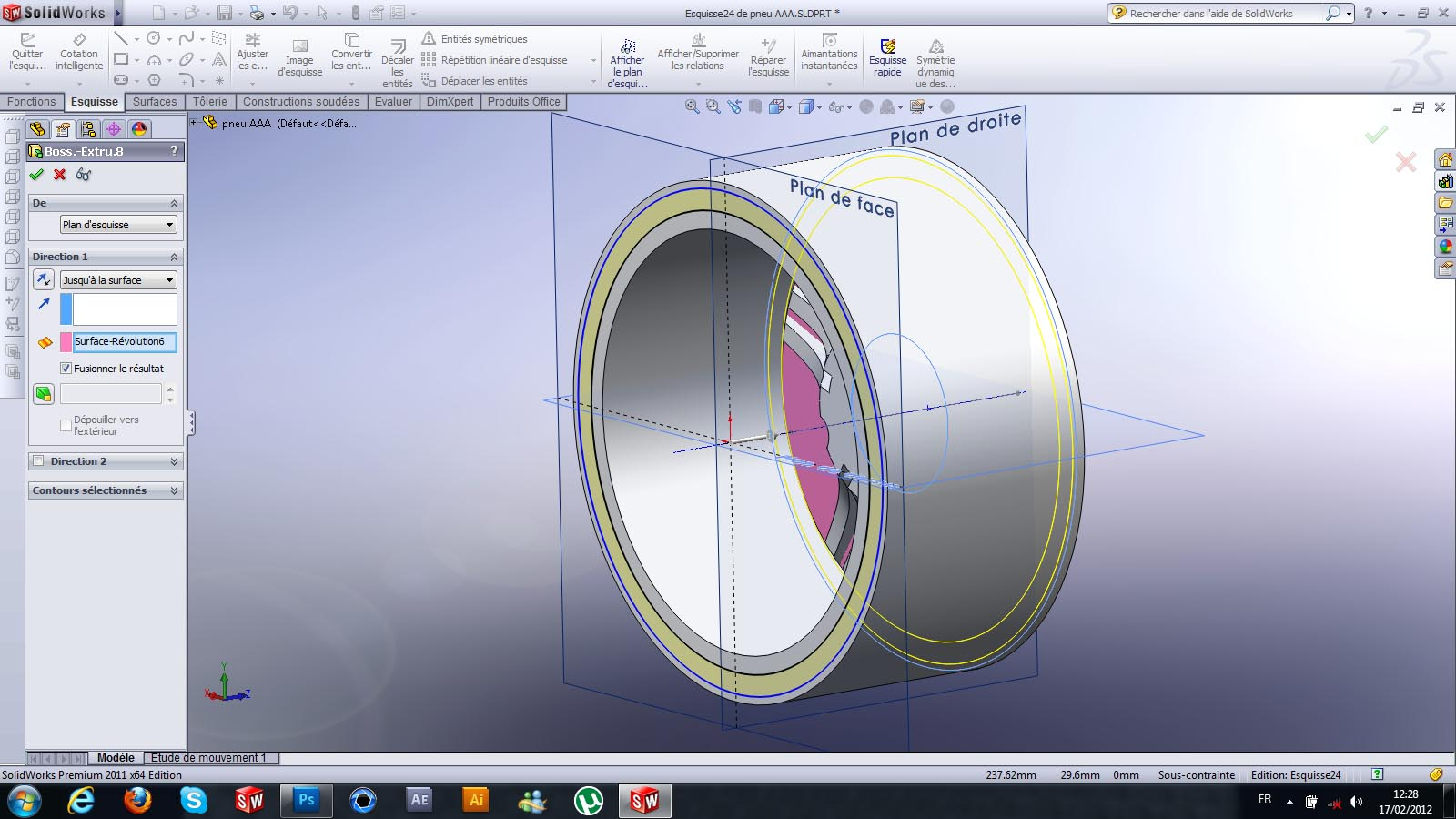33. ### Step 33:

.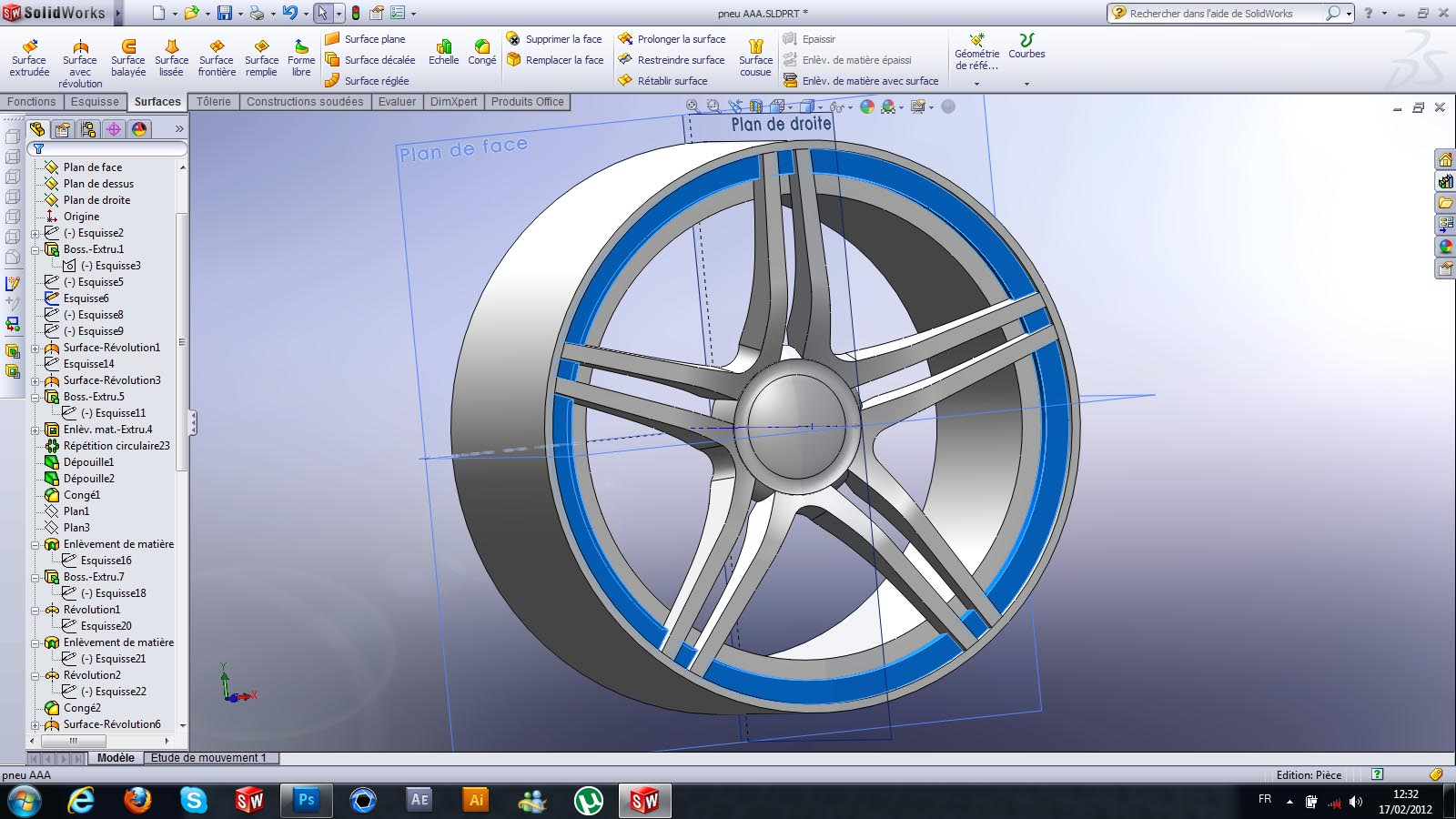34. ### Step 34:

.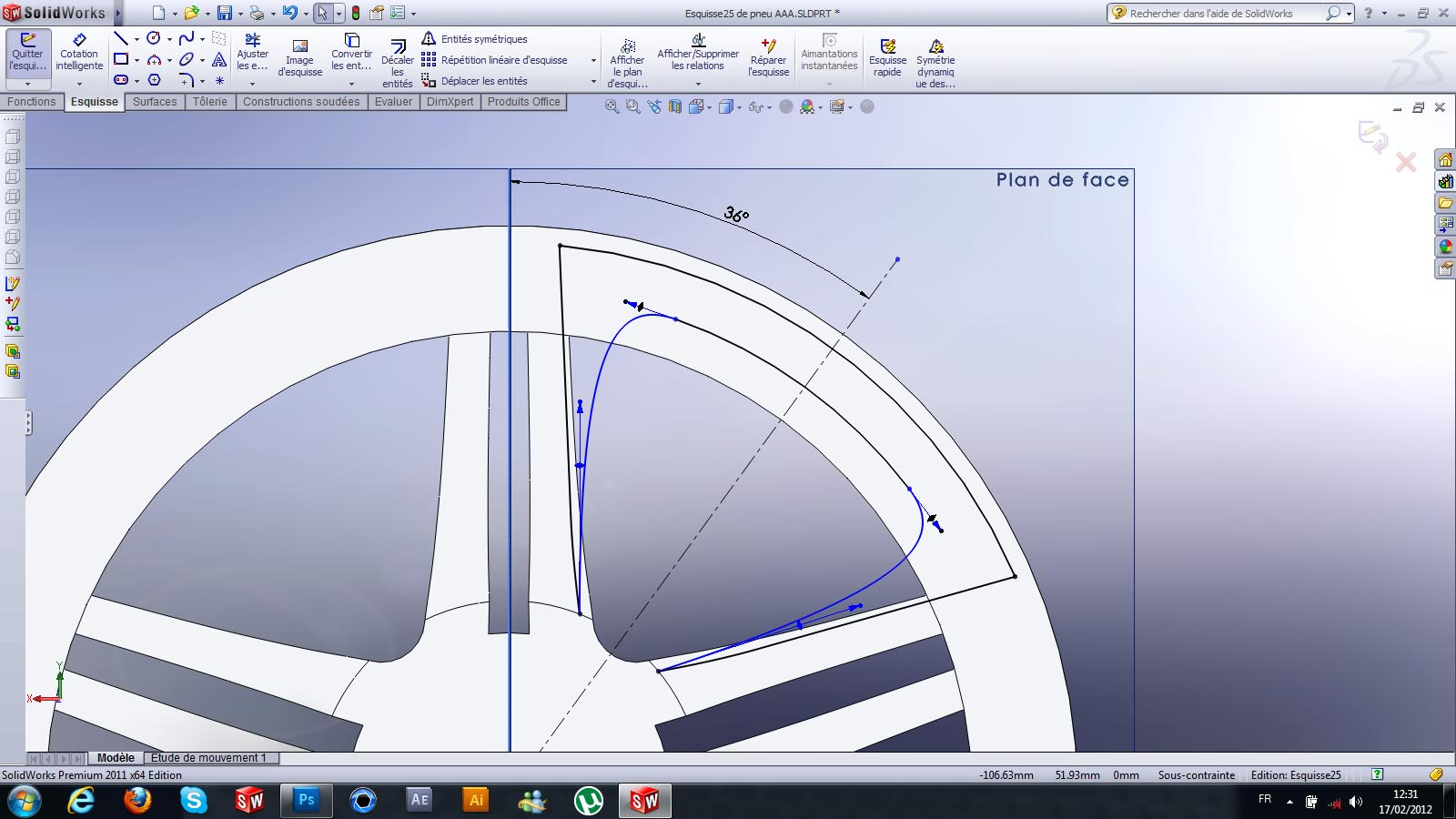35. ### Step 35:

.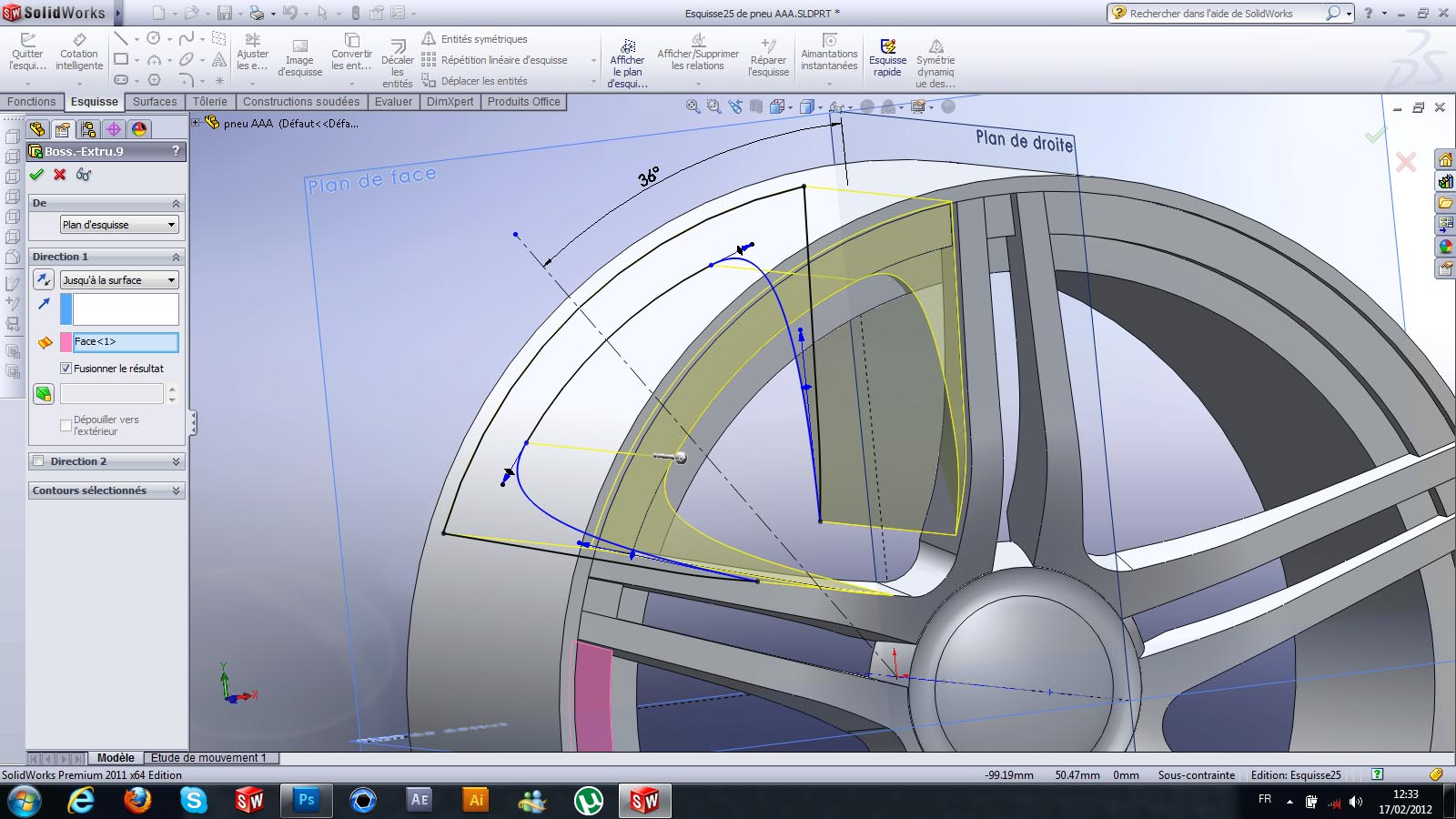36. ### Step 36:

.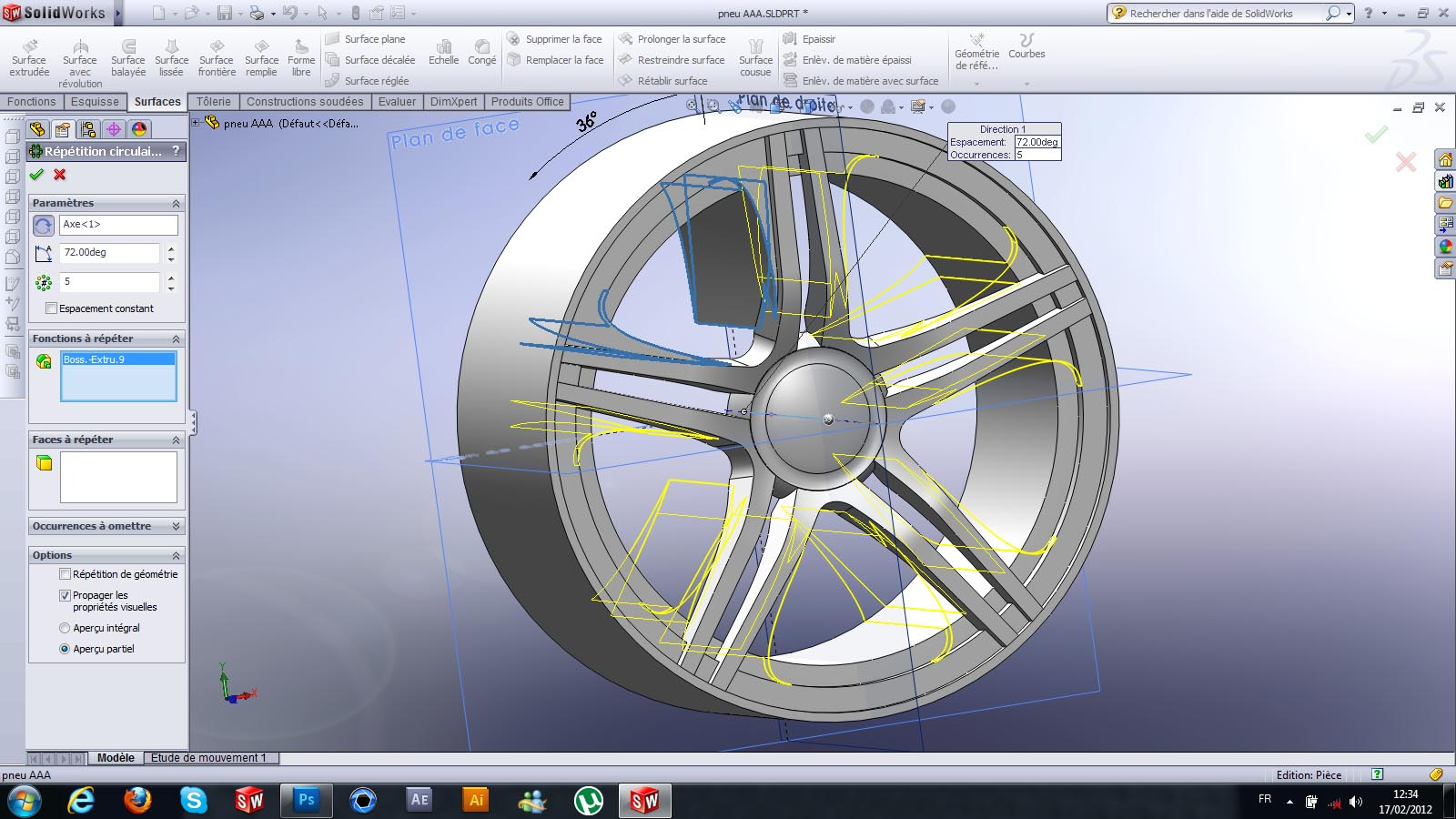37. ### Step 37:

.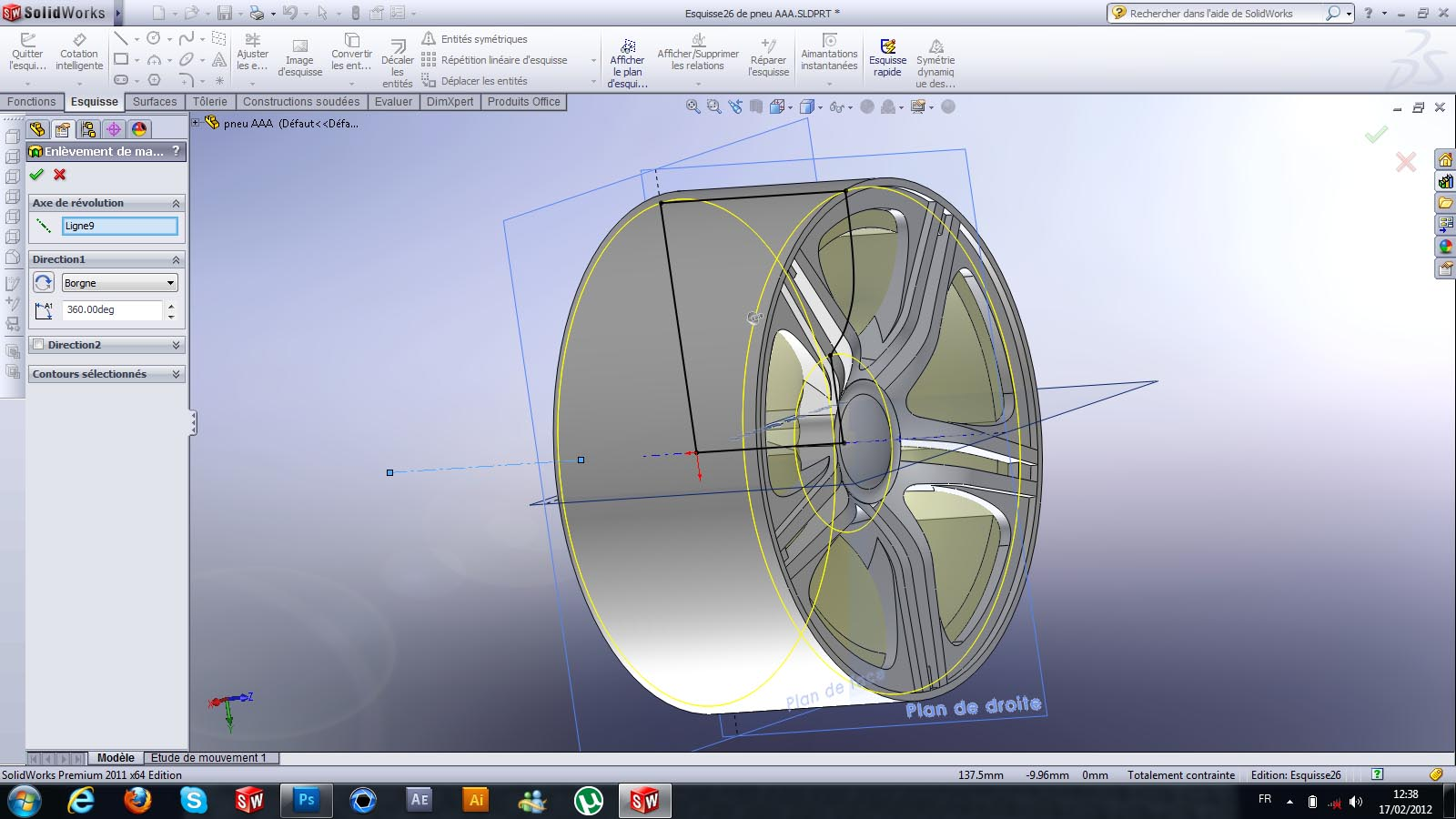38. ### Step 38:

.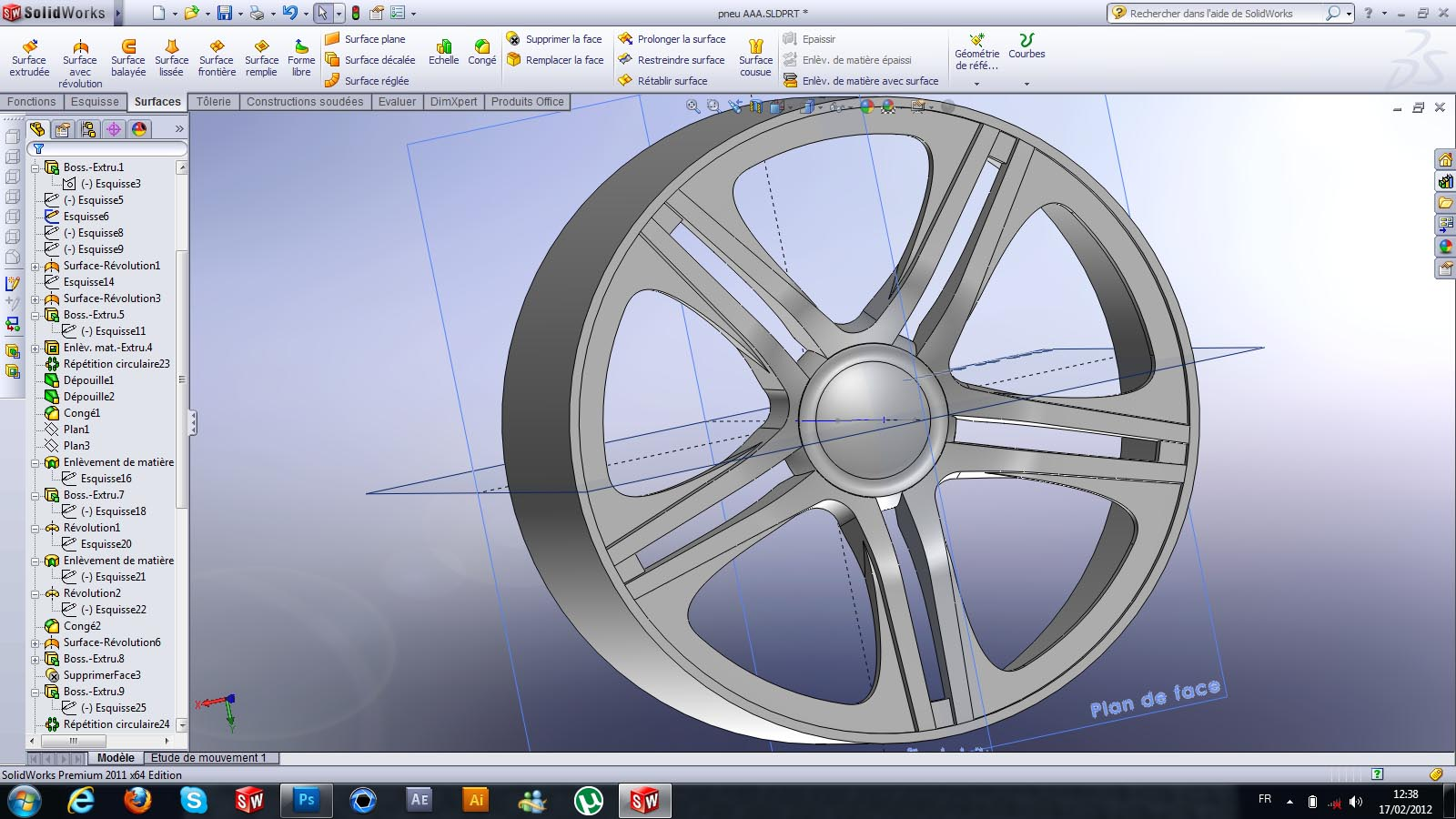39. ### Step 39:

.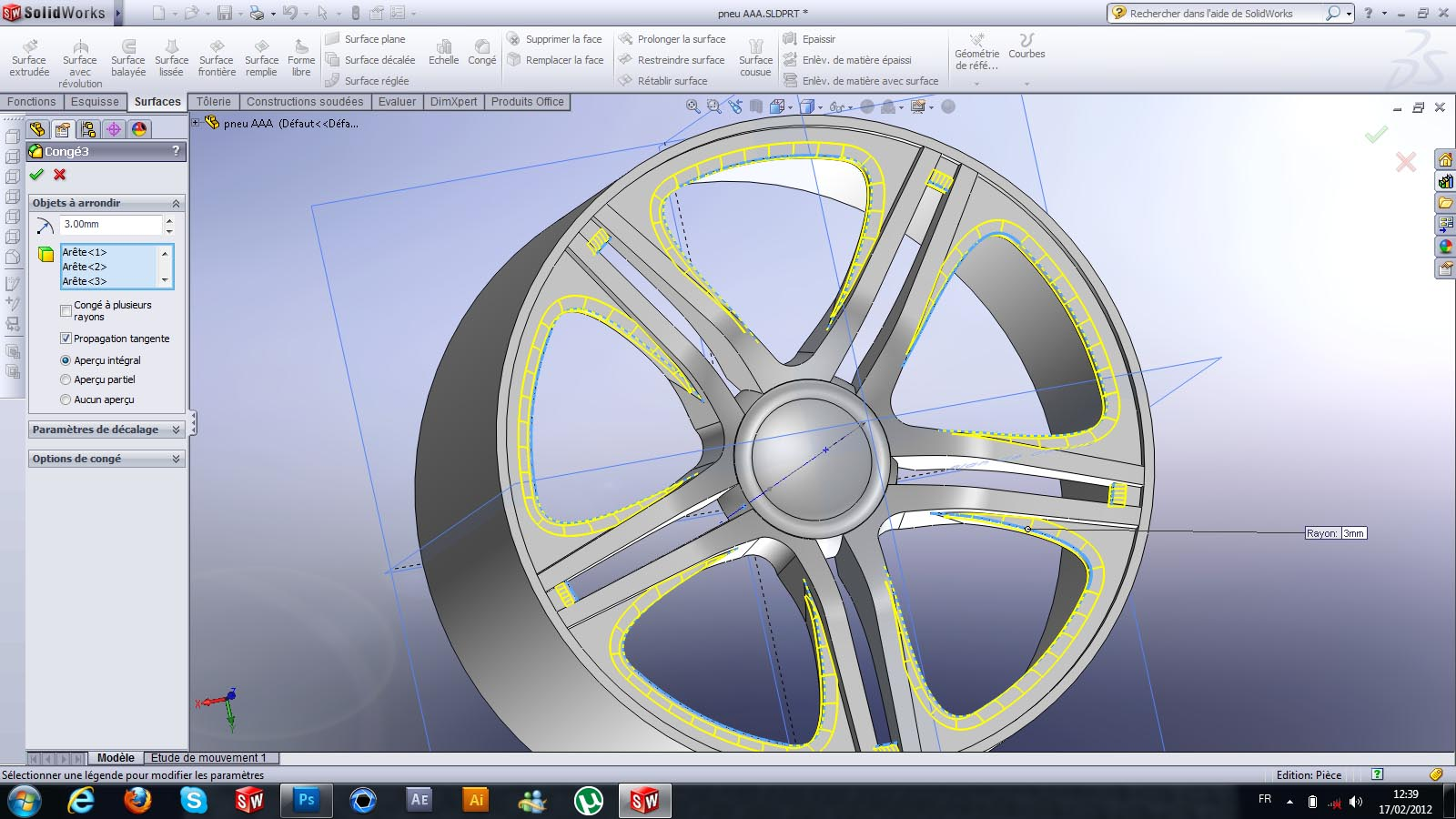40. ### Step 40:

.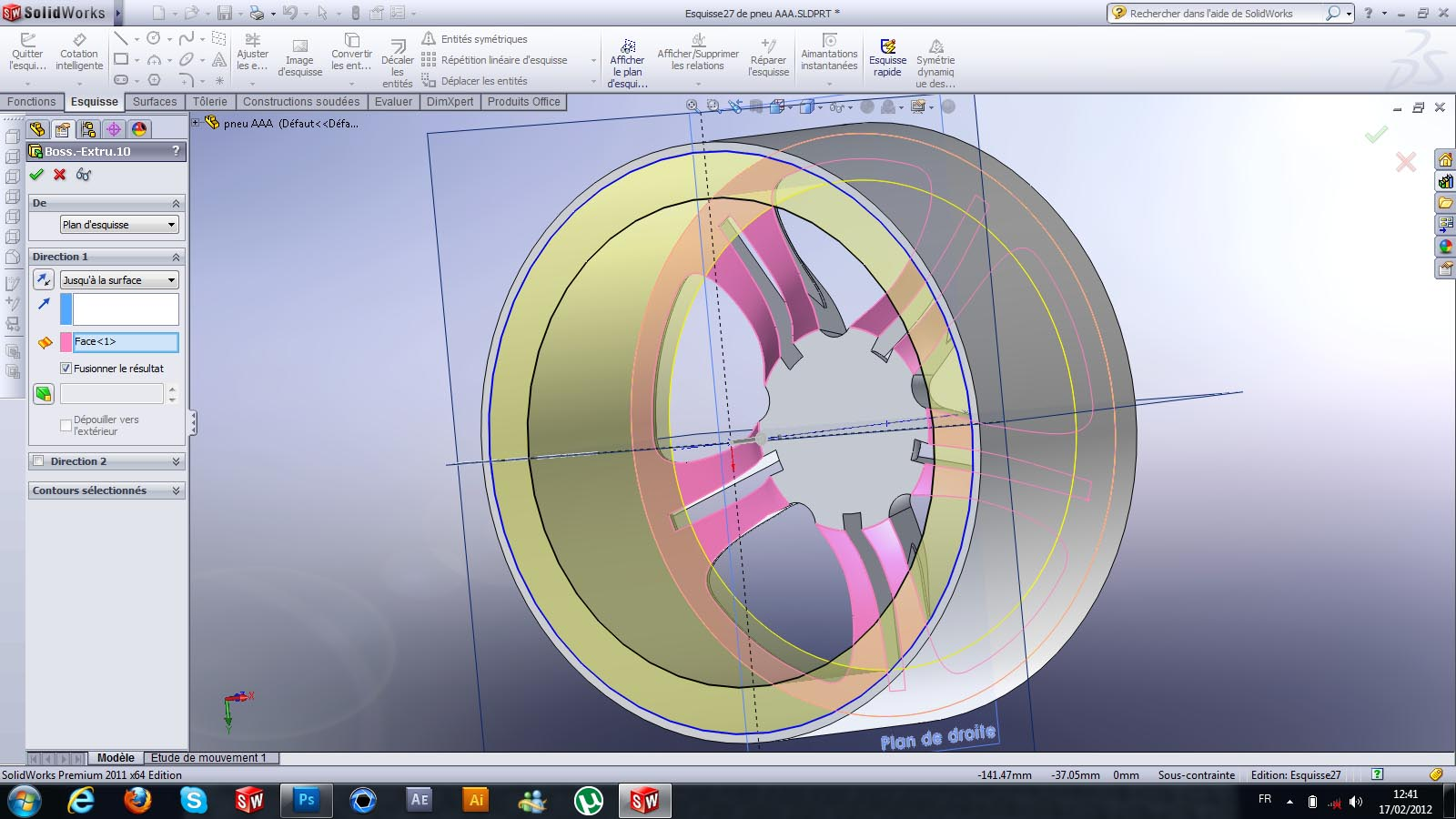41. ### Step 41:

.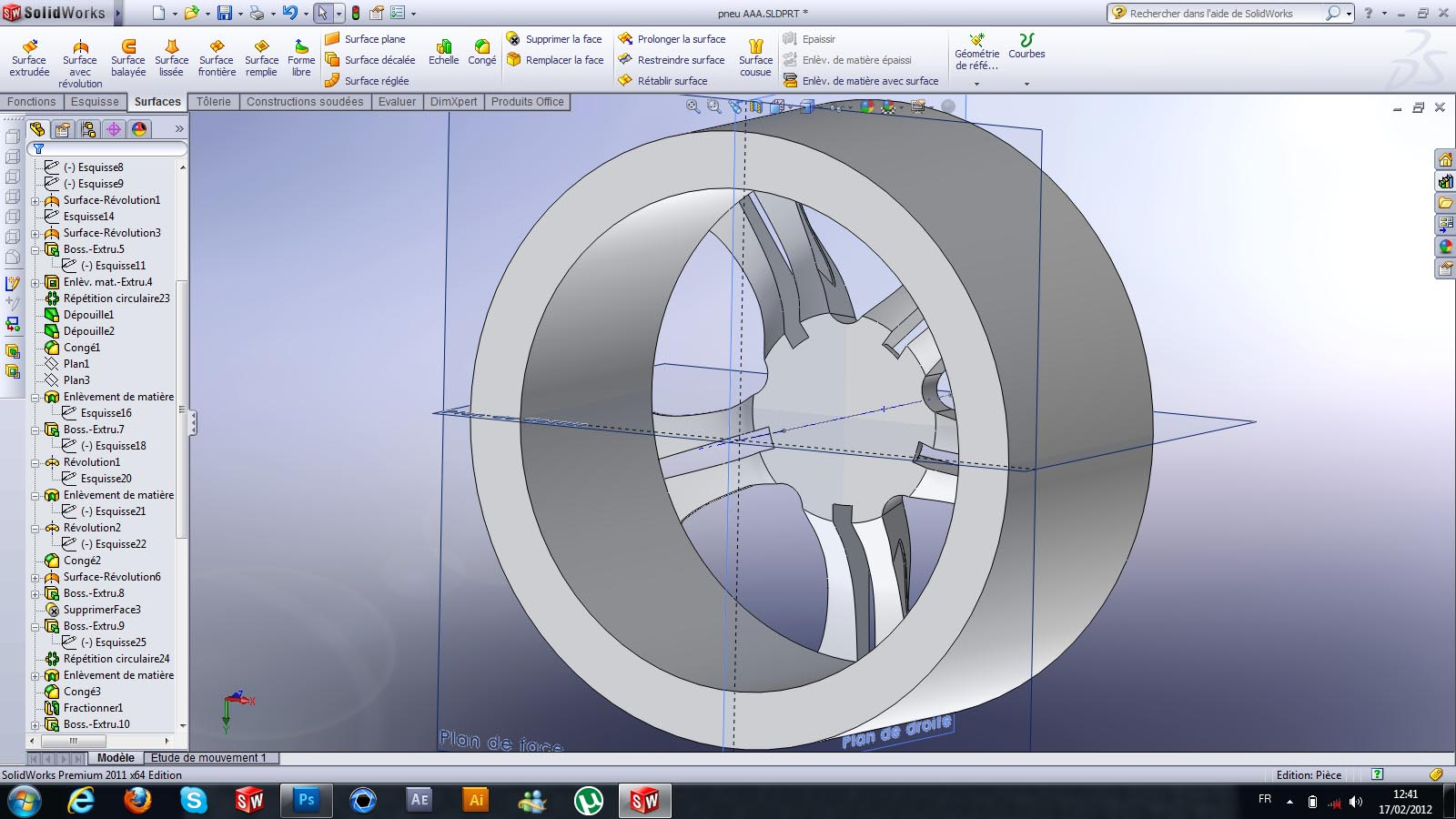42. ### Step 42:

.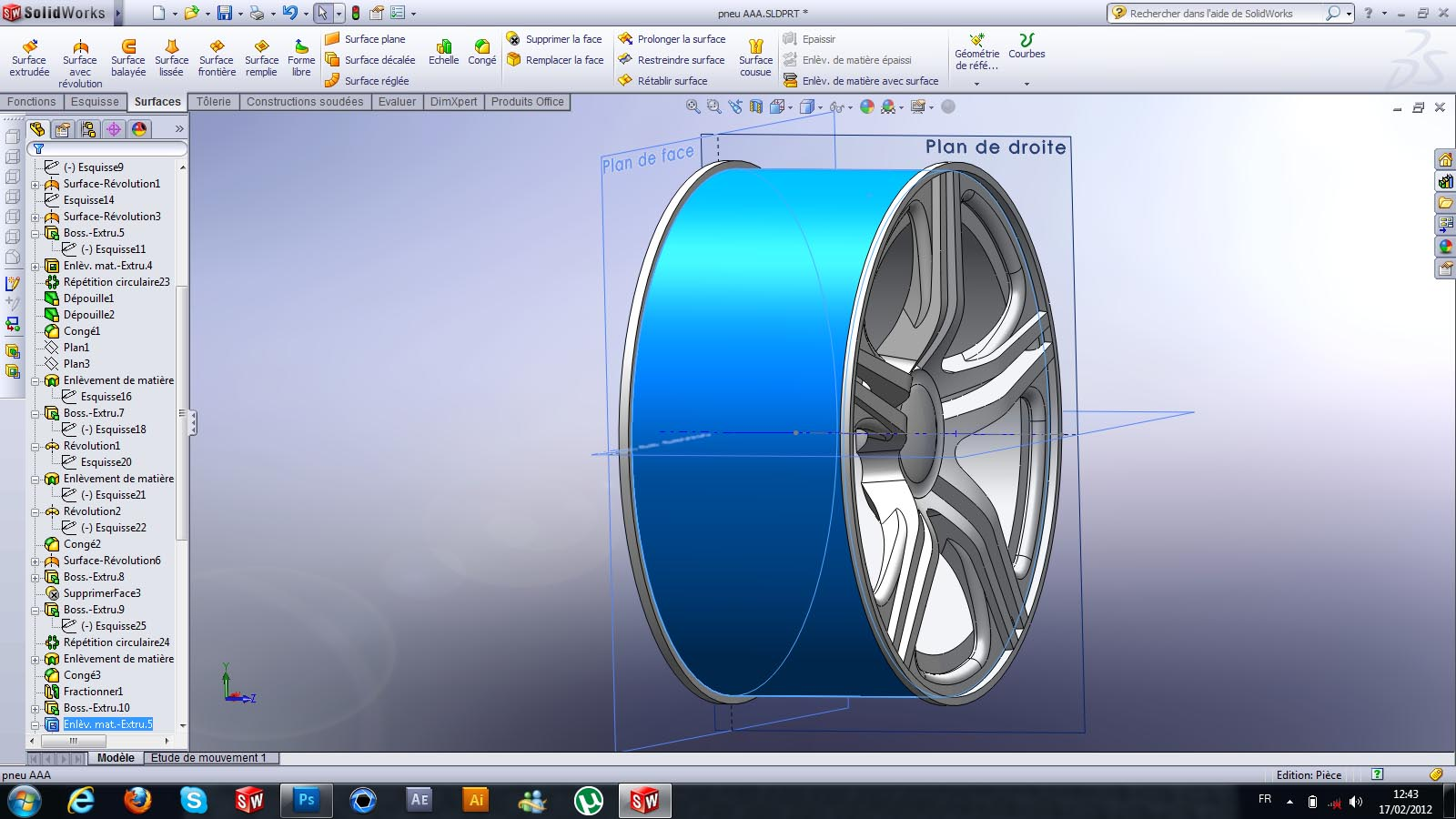43. ### Step 43:

.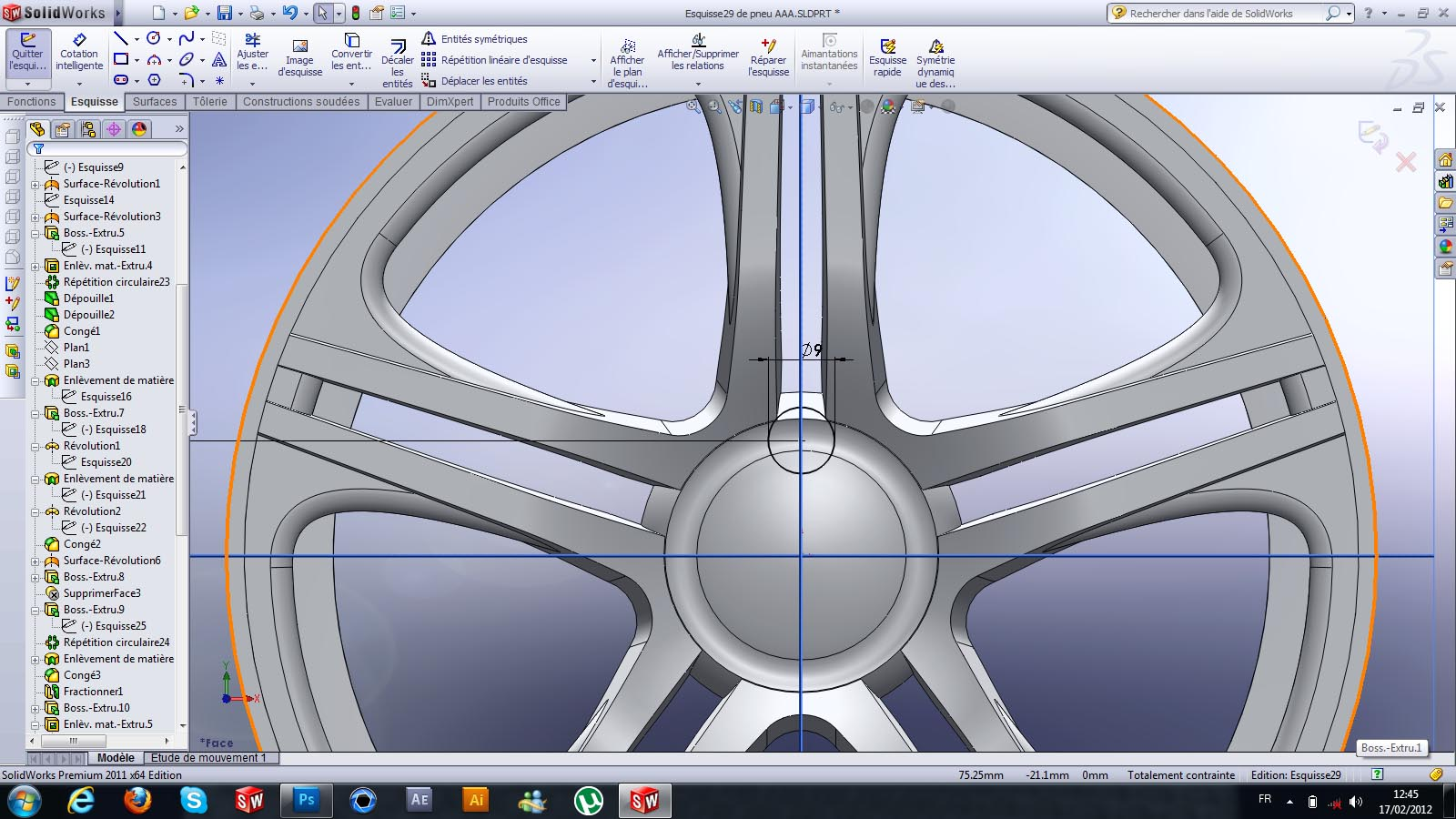44. ### Step 44:

.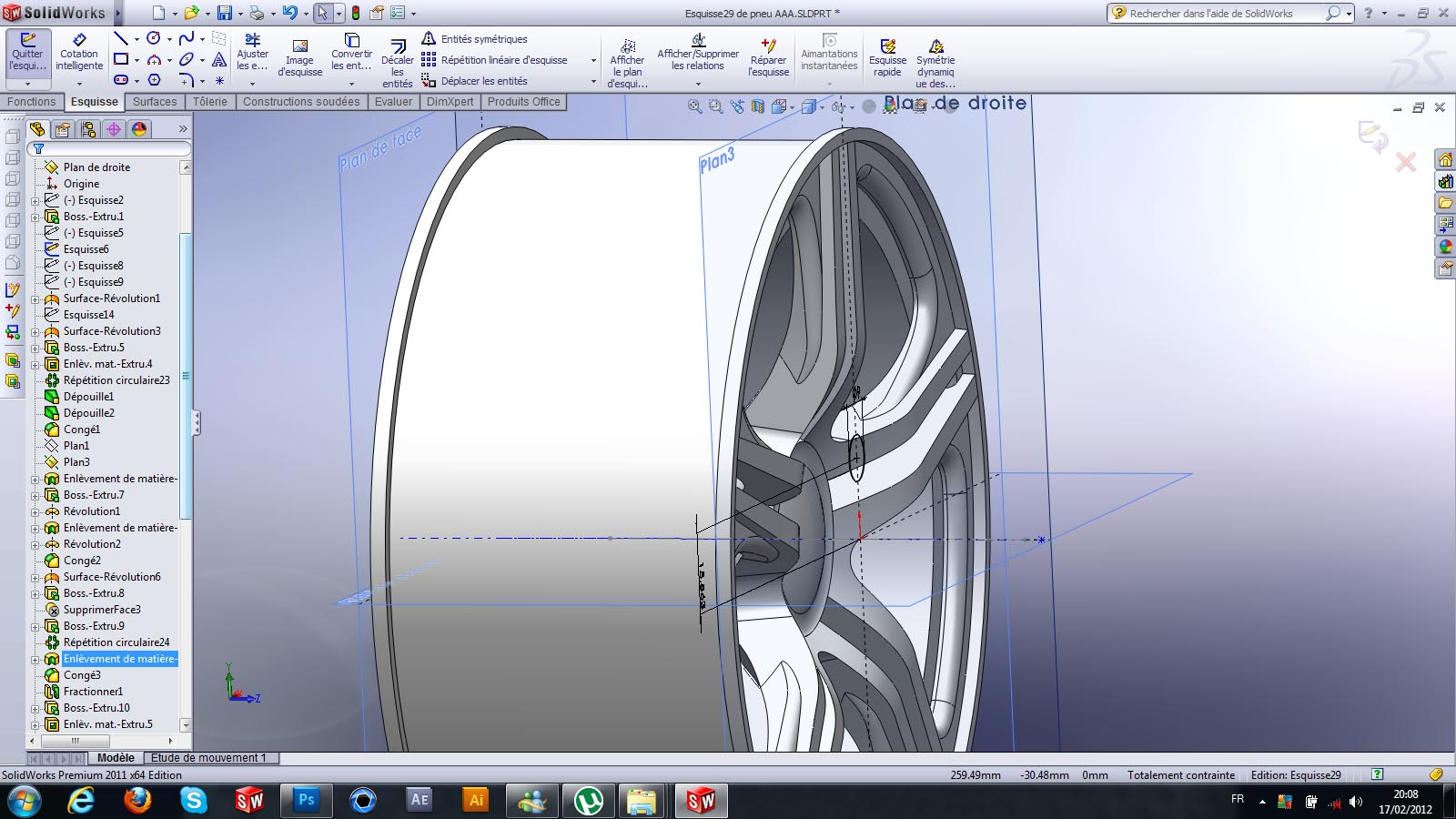45. ### Step 45:

.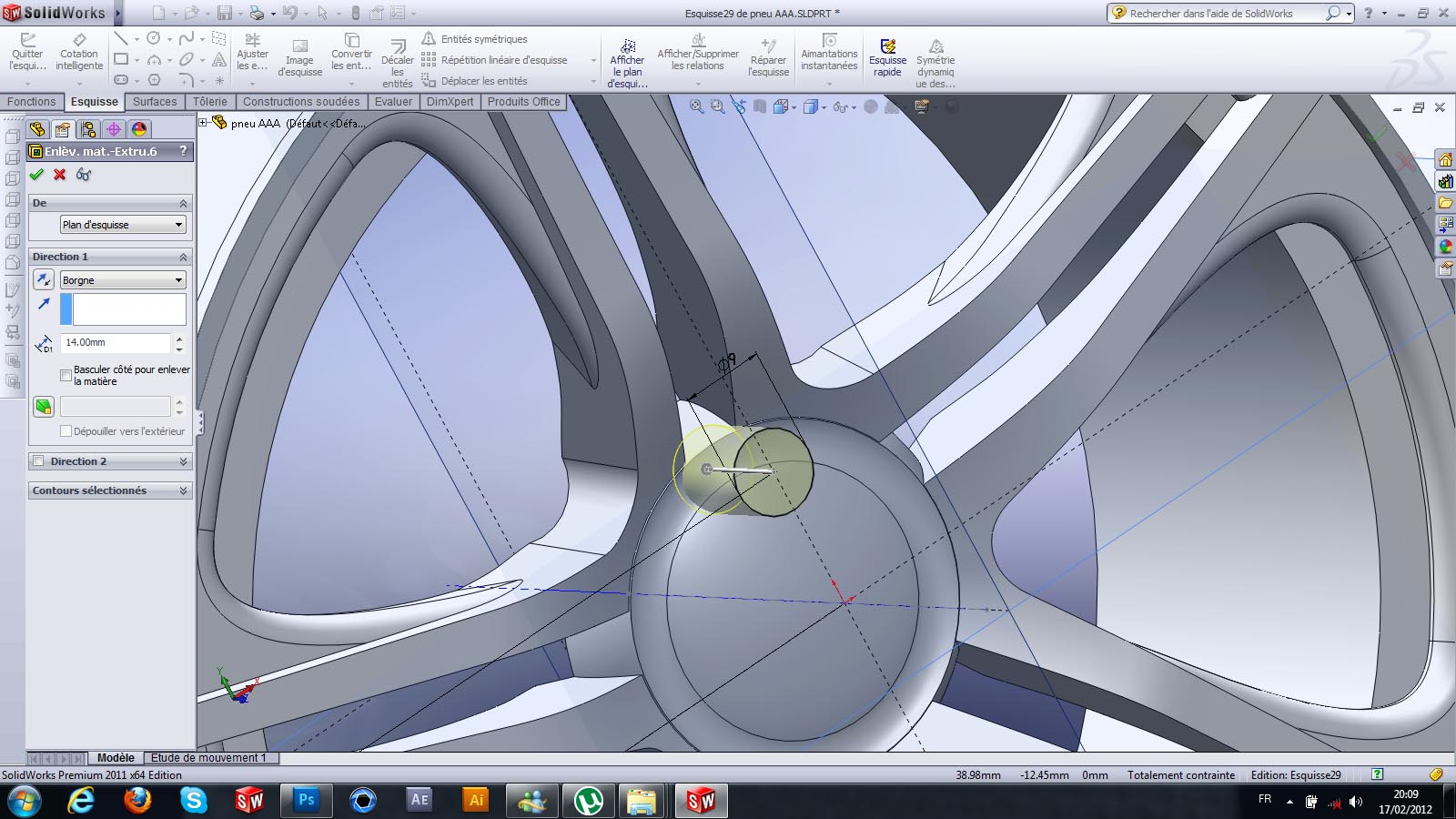46. ### Step 46:

.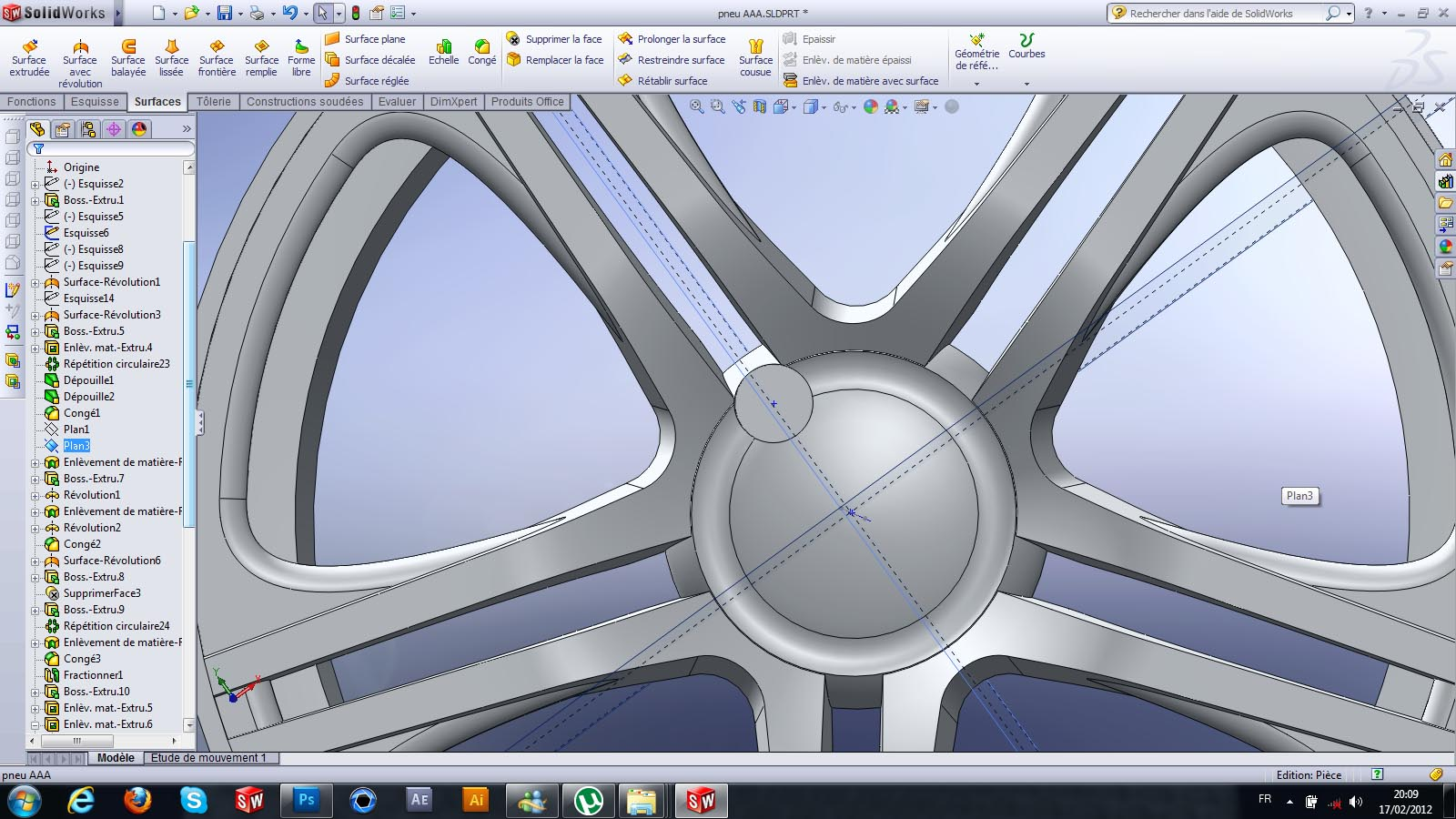47. ### Step 47:

.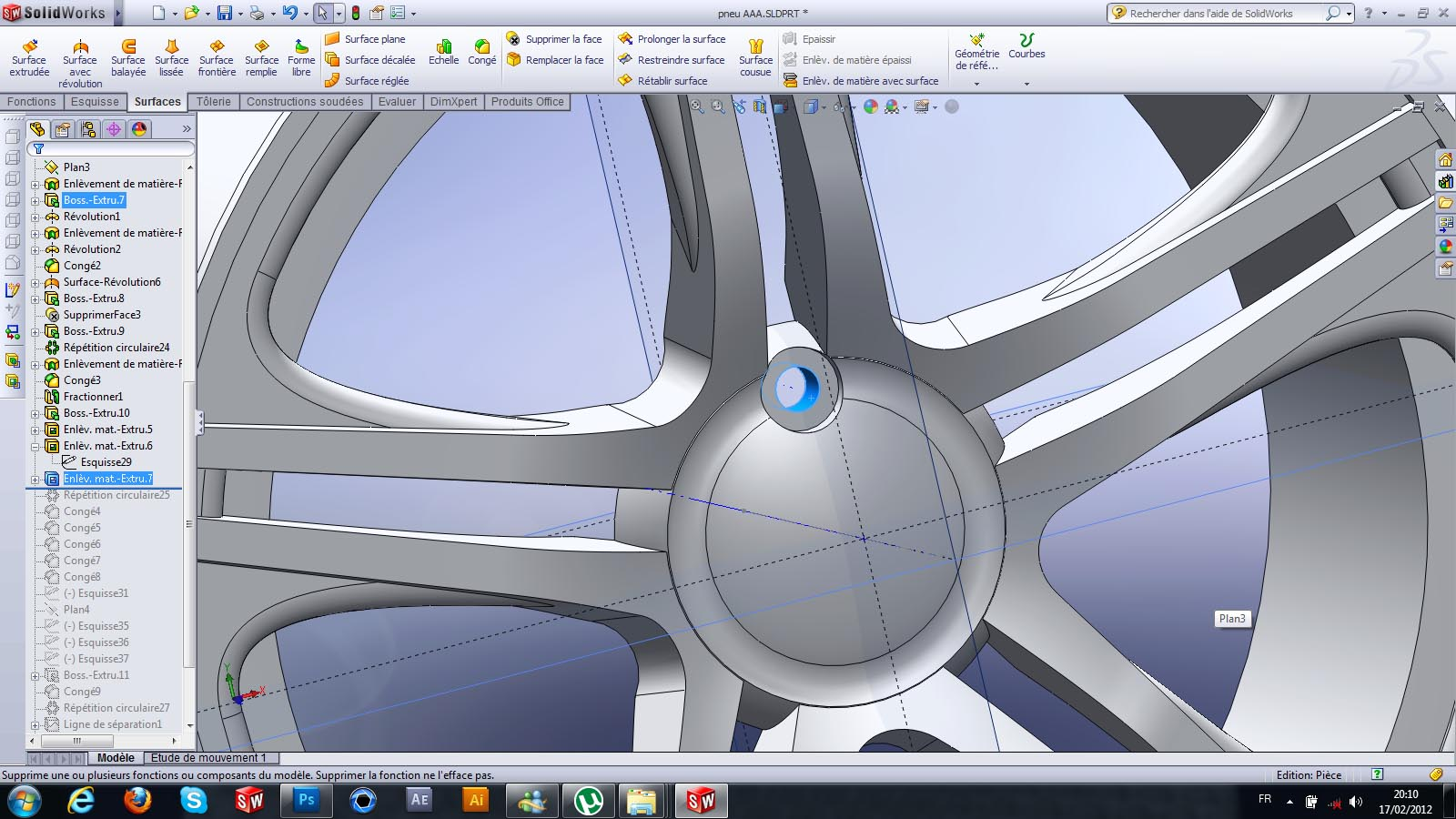48. ### Step 48:

.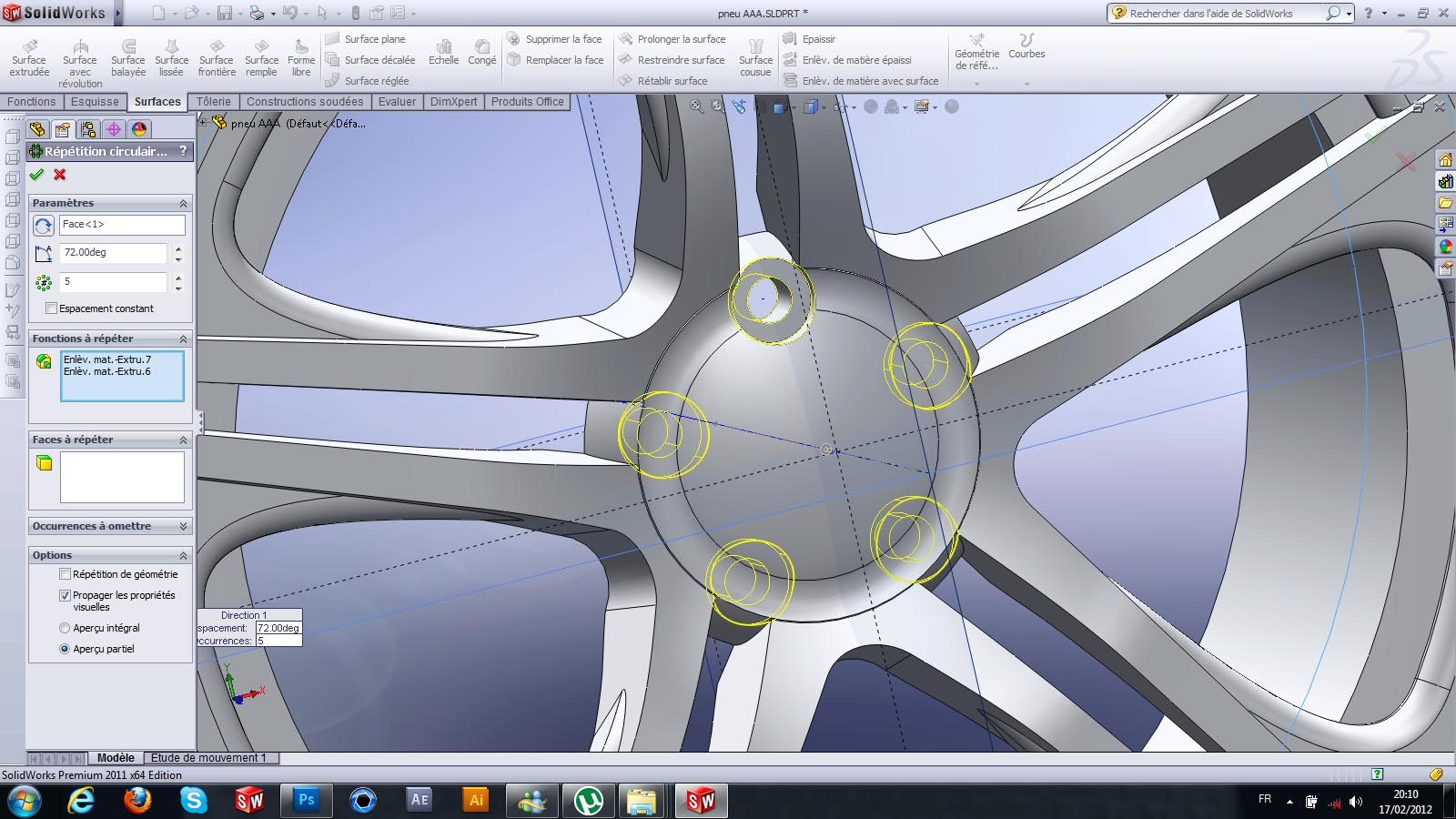49. ### Step 49:

.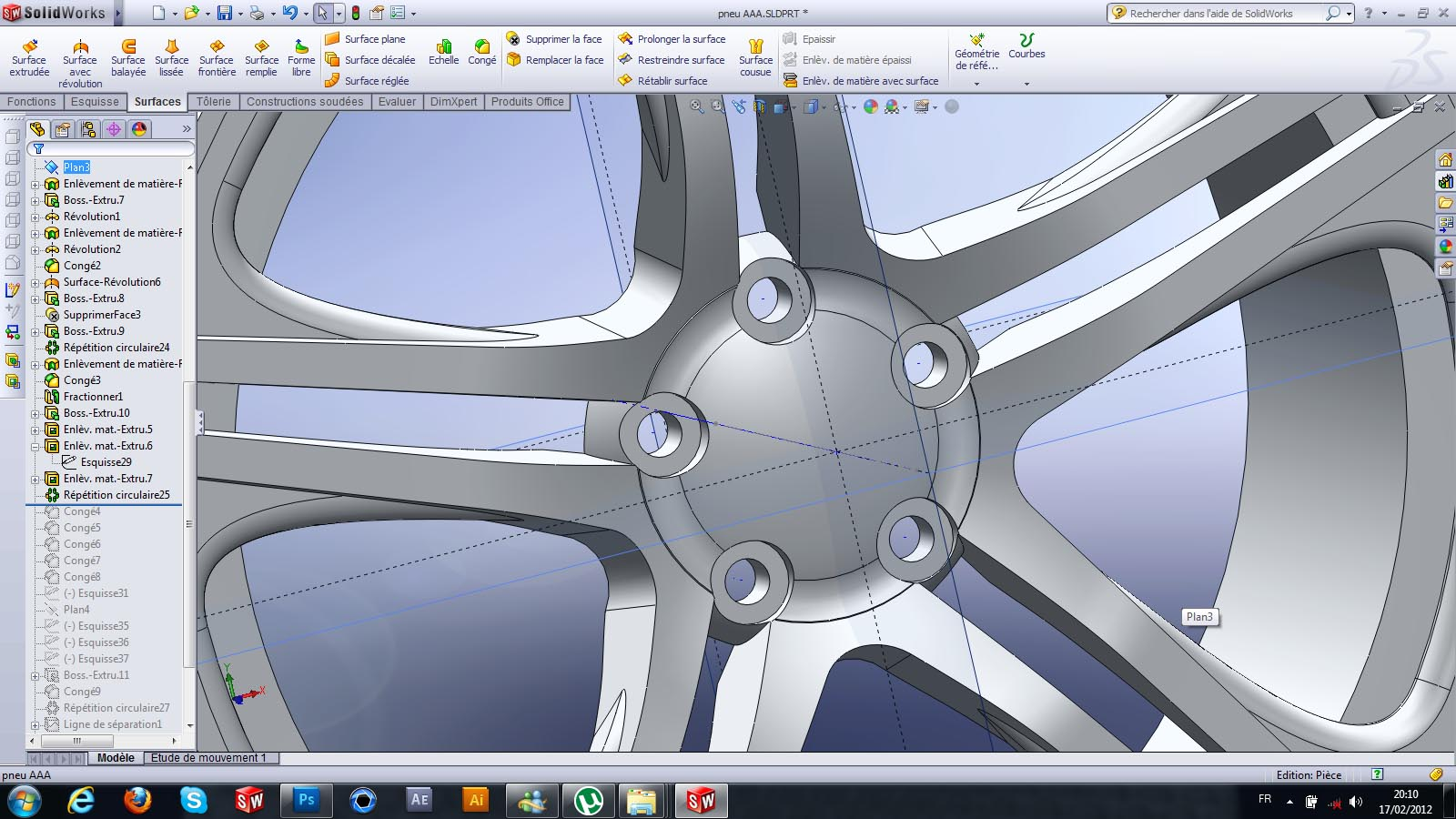50. ### Step 50:

.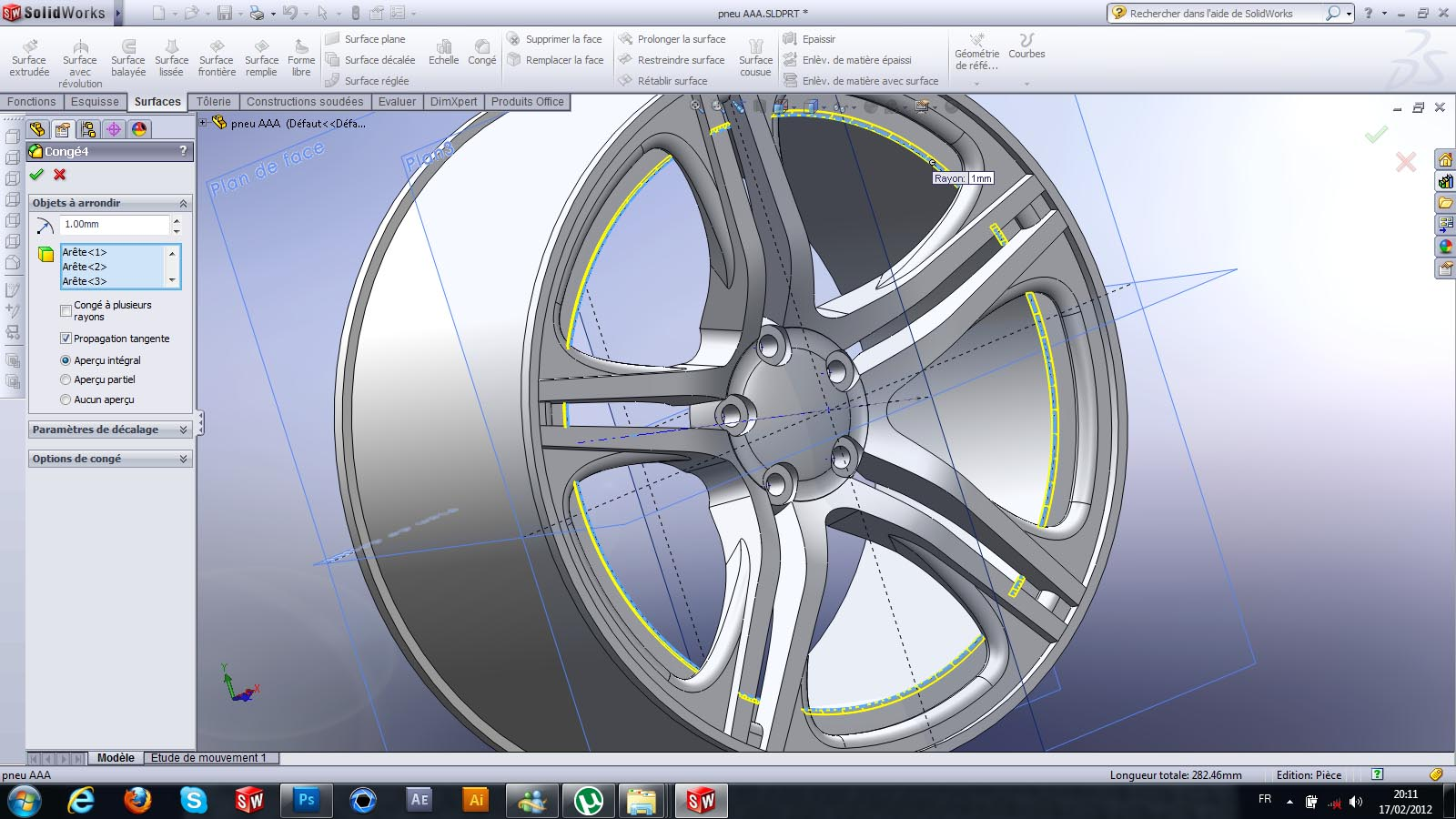51. ### Step 51:

.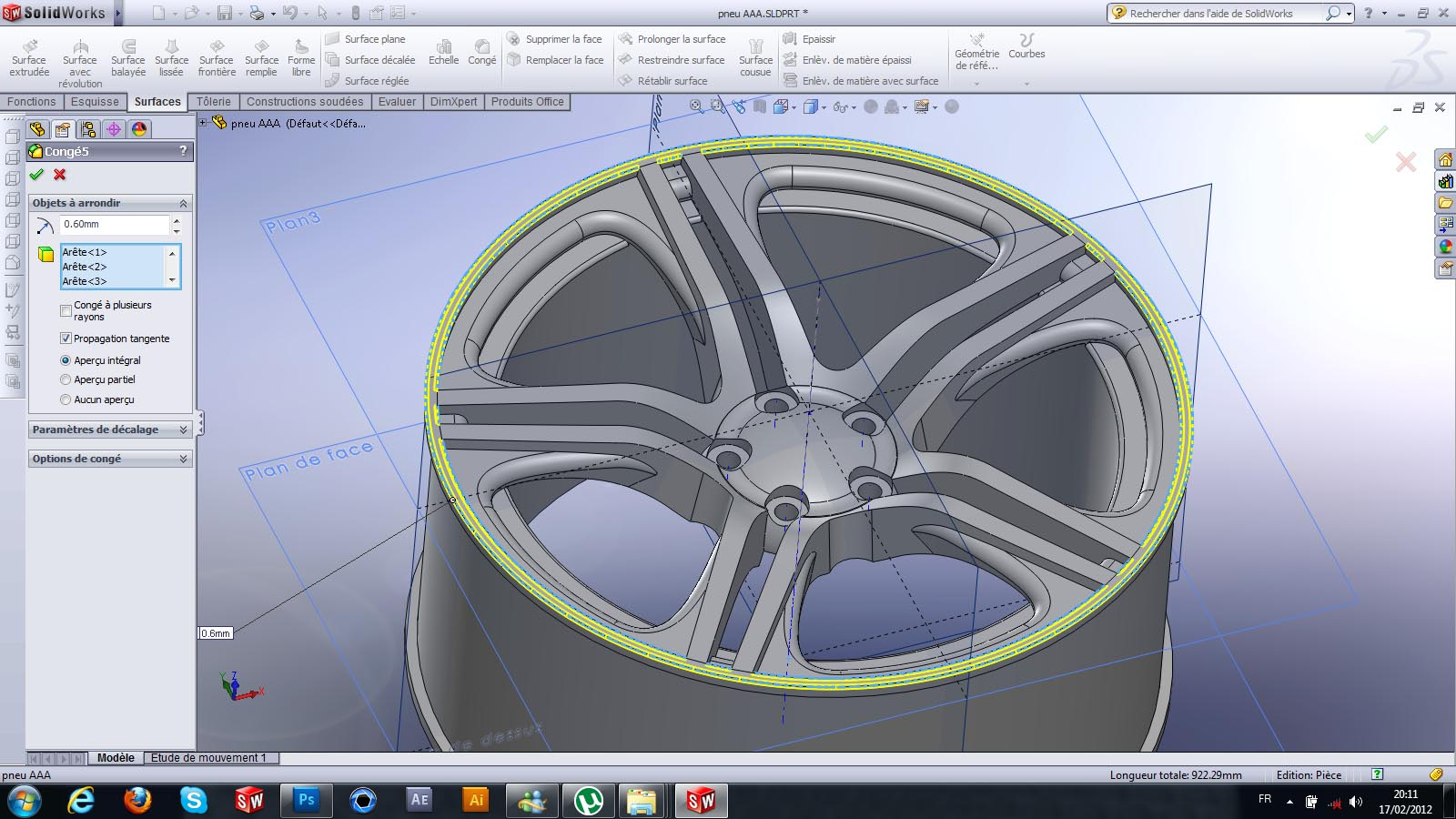52. ### Step 52:

.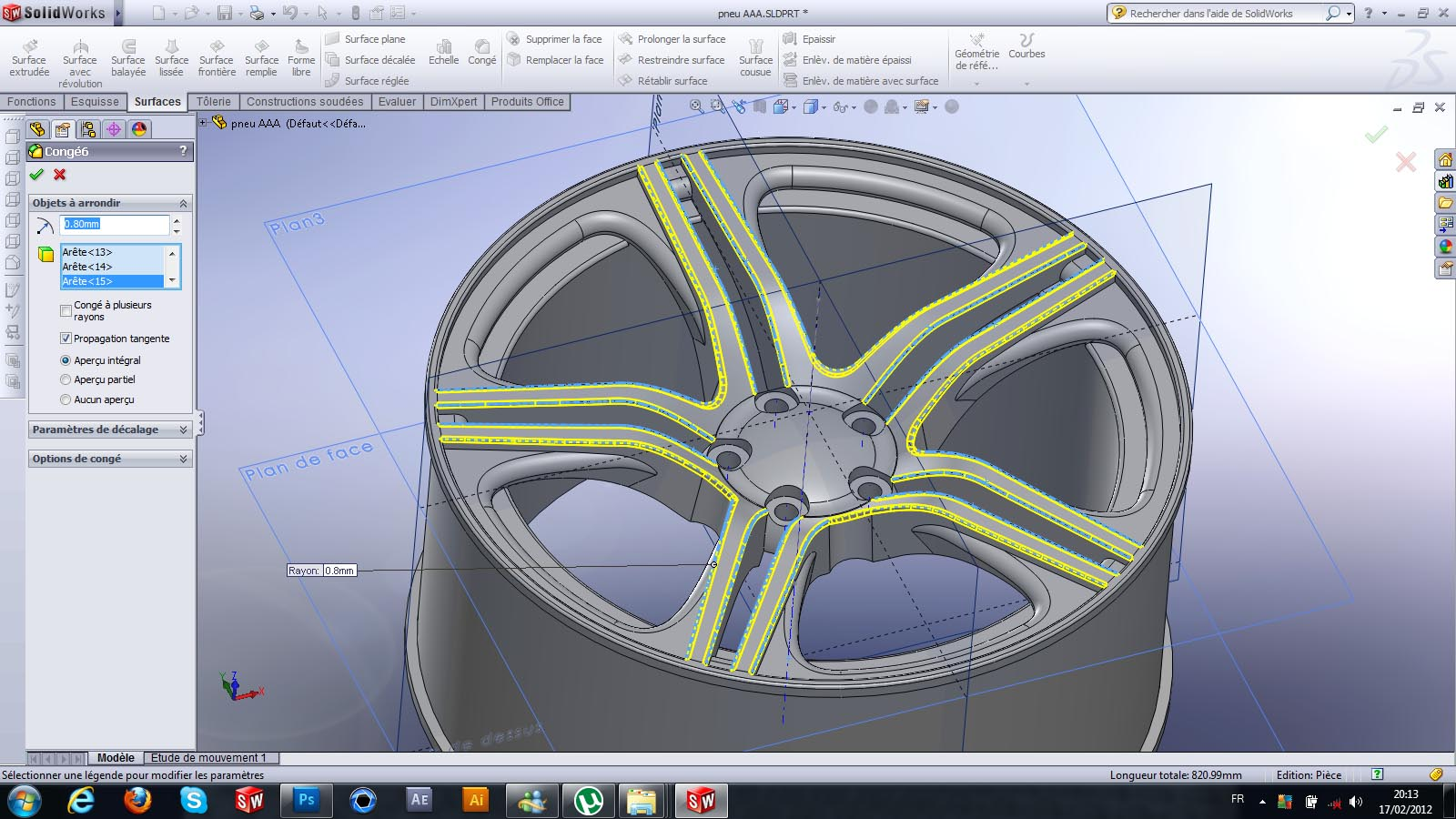53. ### Step 53:

.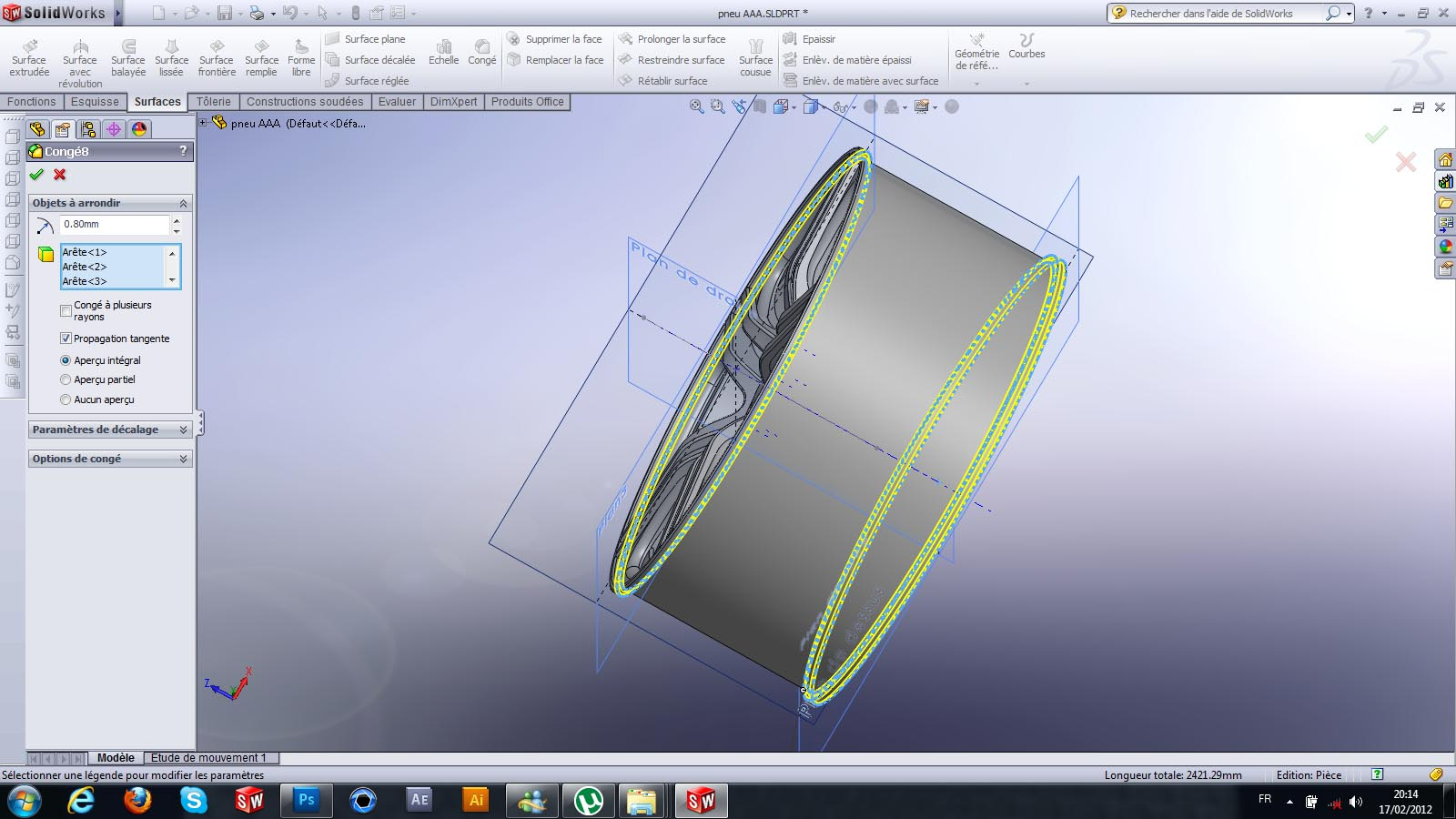54. ### Step 54:

.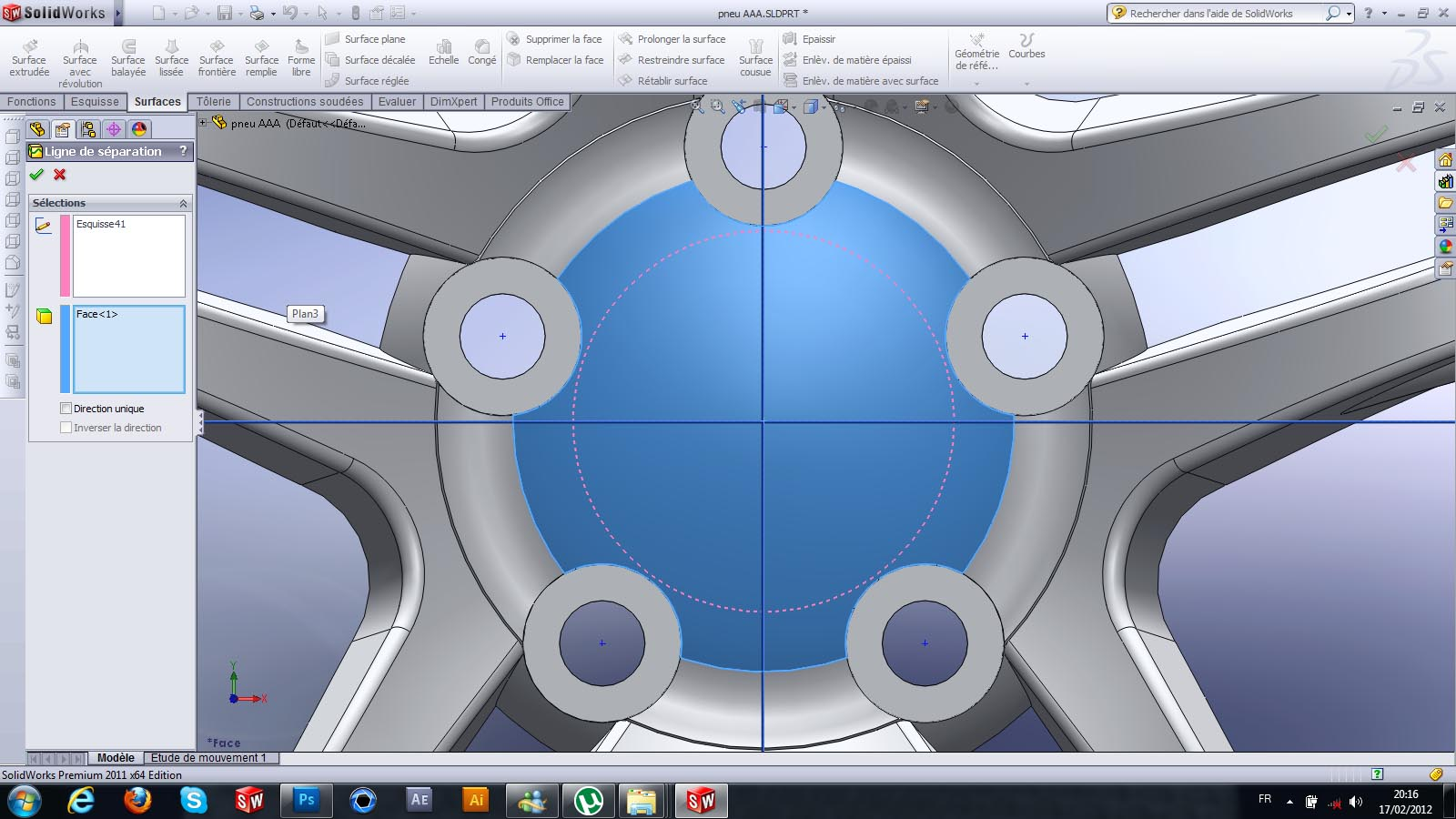55. ### Step 55:

.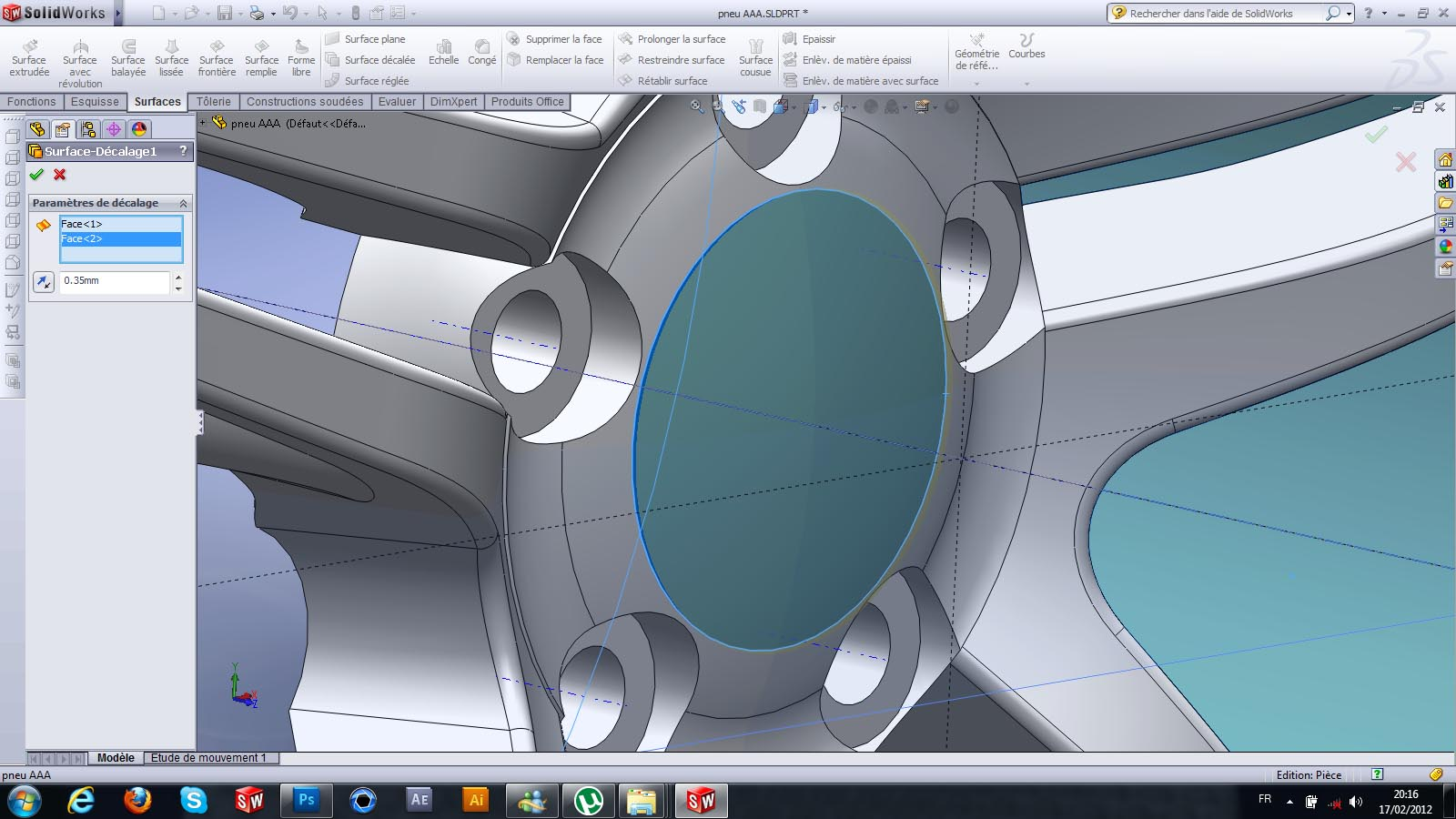56. ### Step 56:

.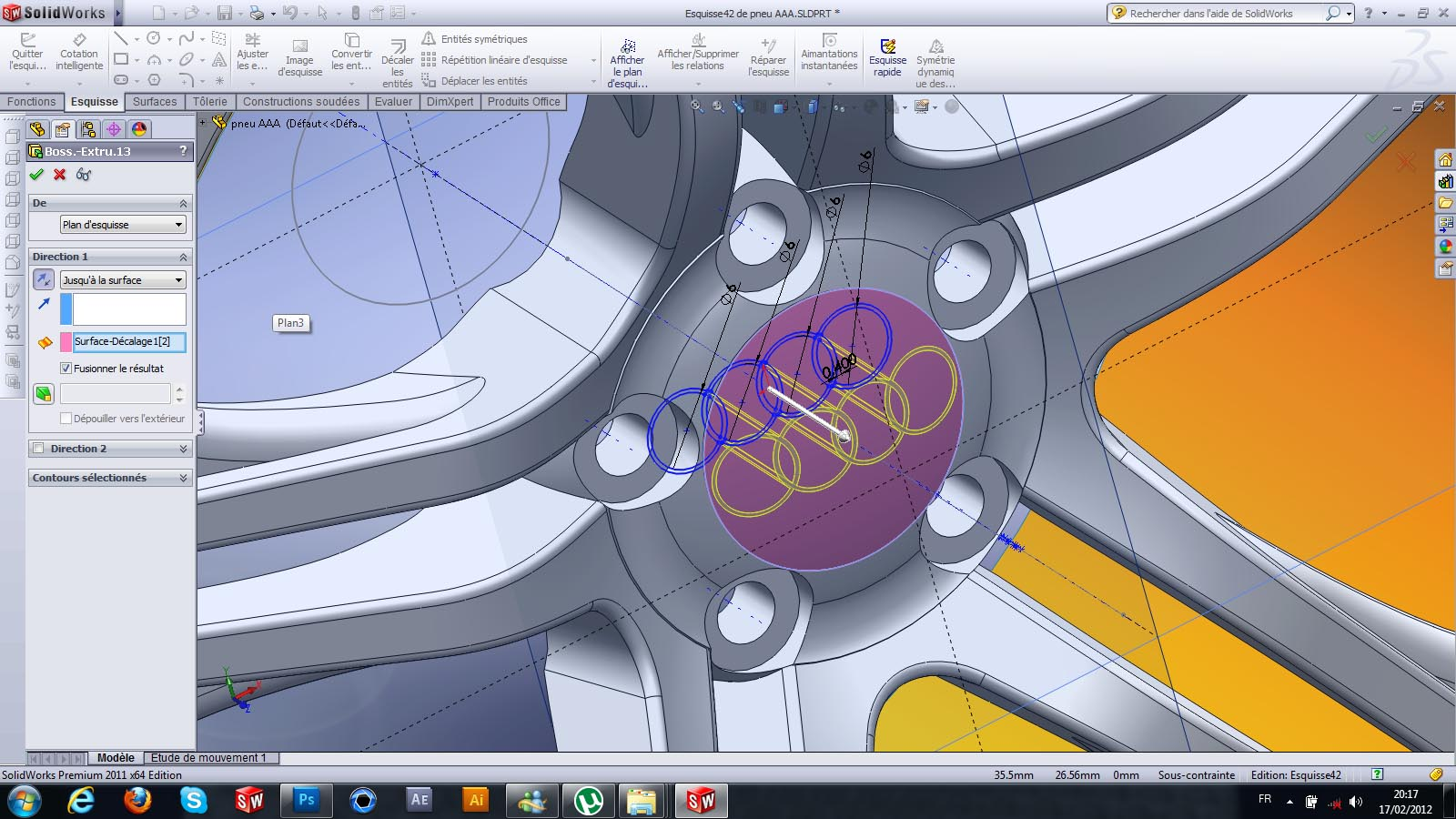57. ### Step 57:

.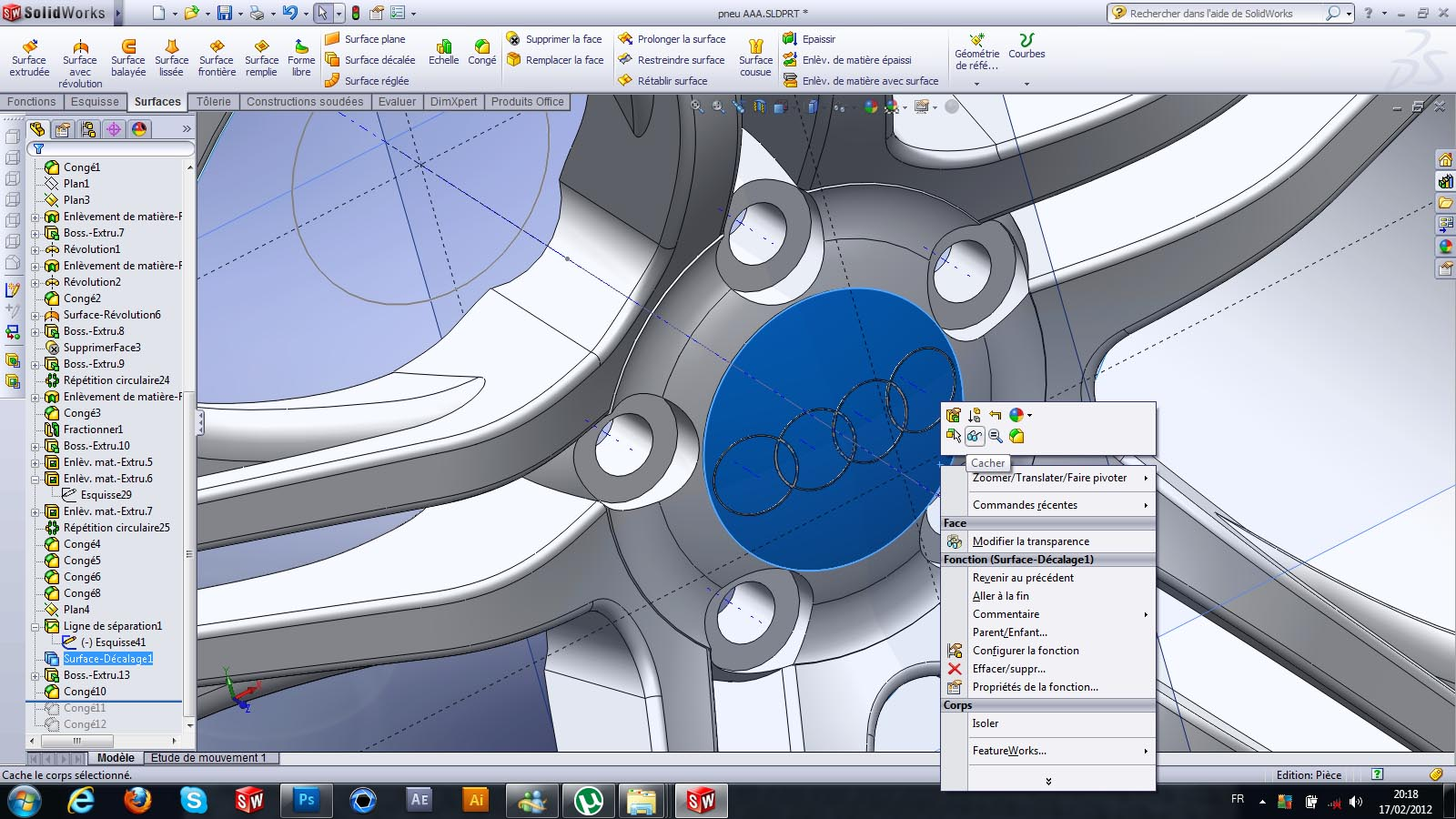58. ### Step 58:

.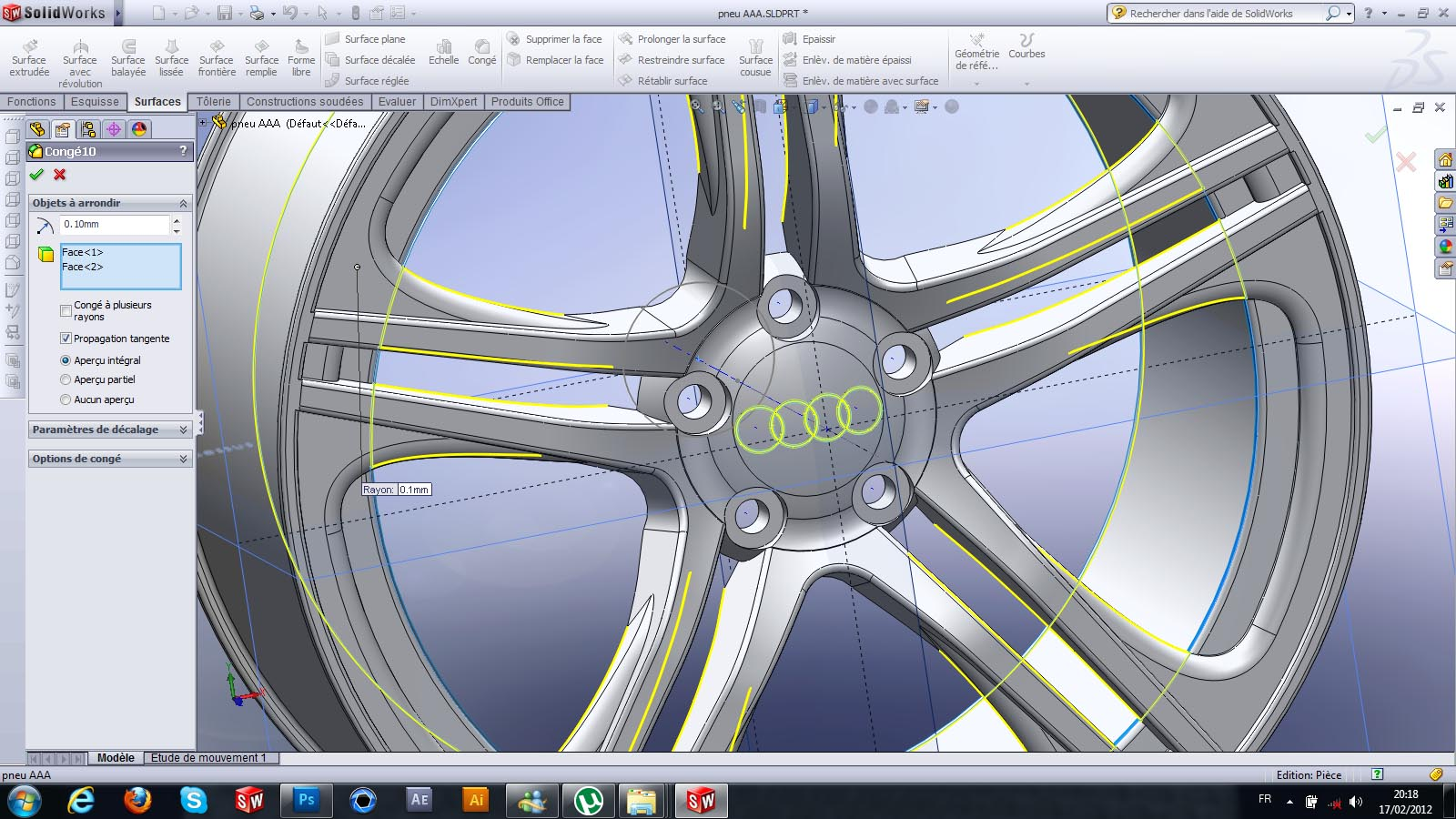59. ### Step 59:

.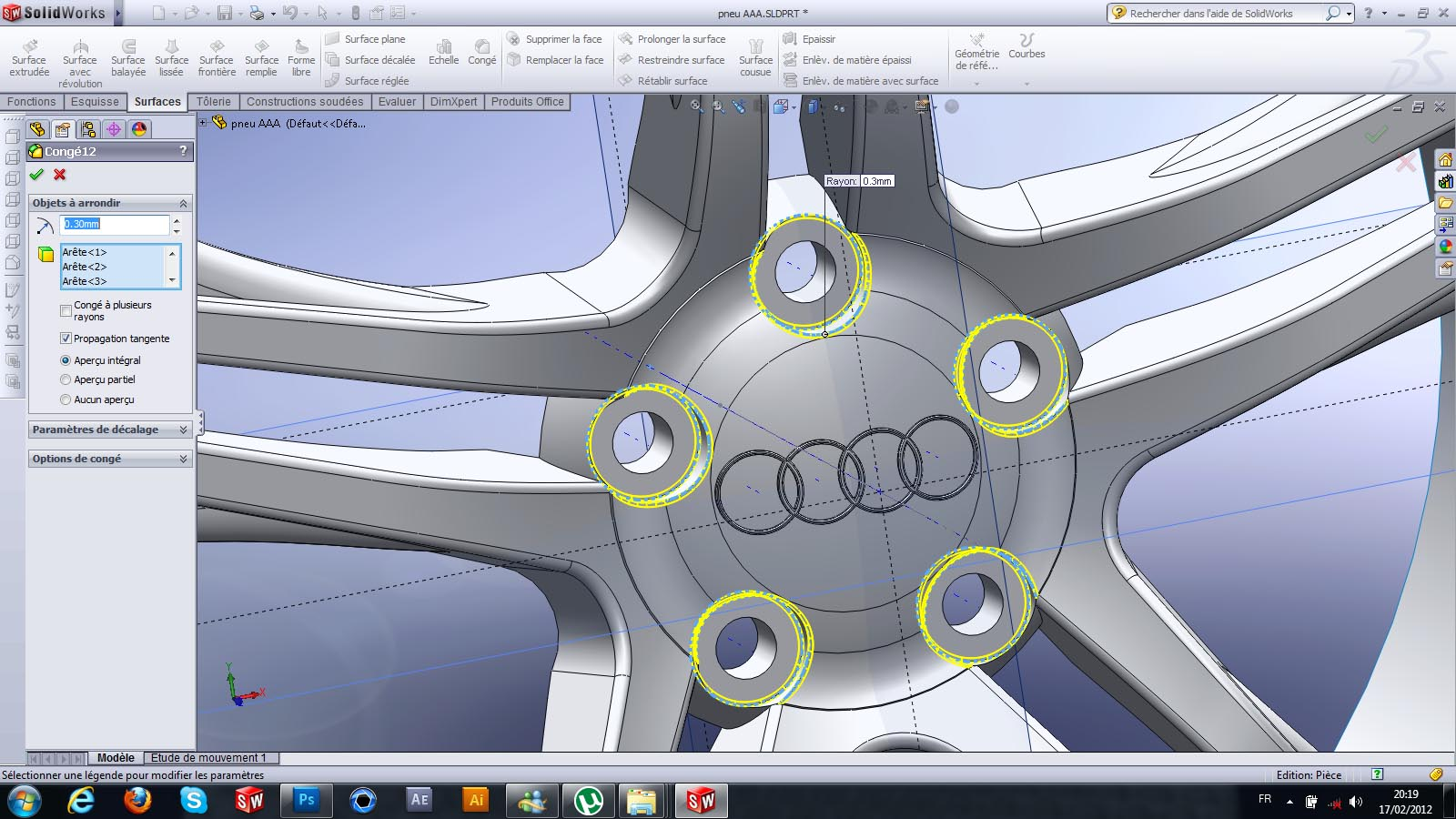60. ### Step 60:

.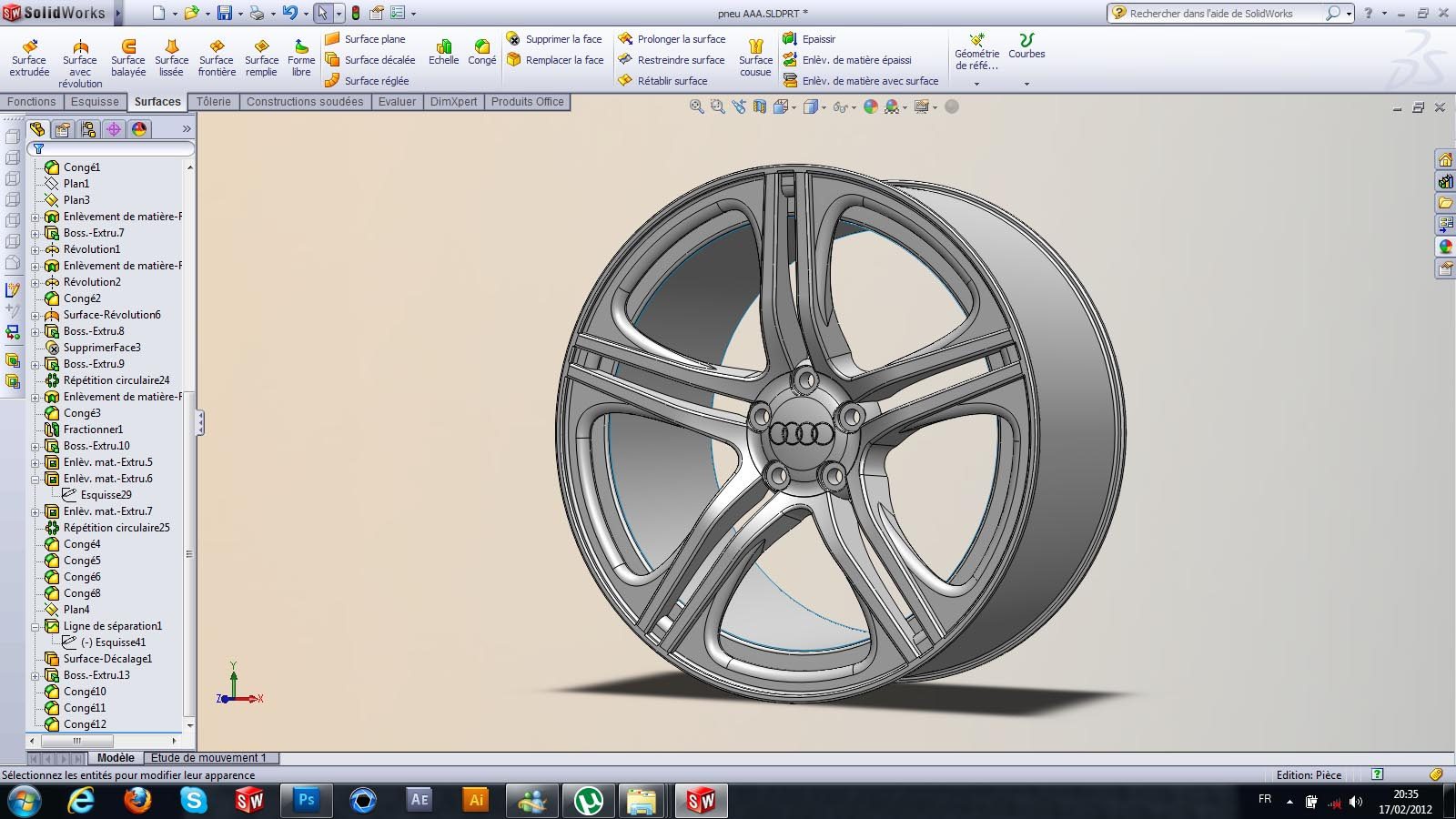61. ### Step 61:

e end of our rim I import the model to KeyShot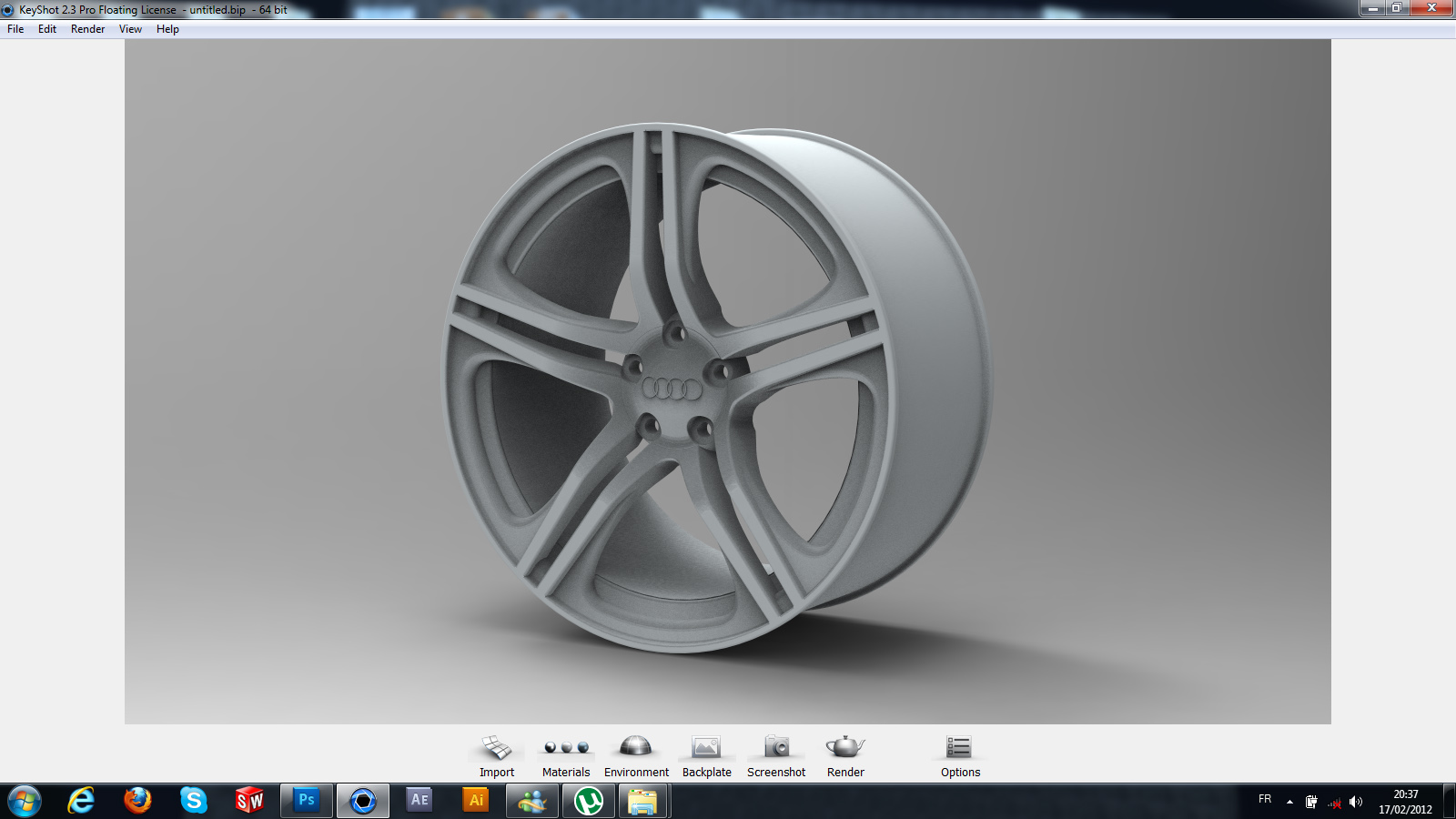62. ### Step 62:

and I do a rotation as seen in the picture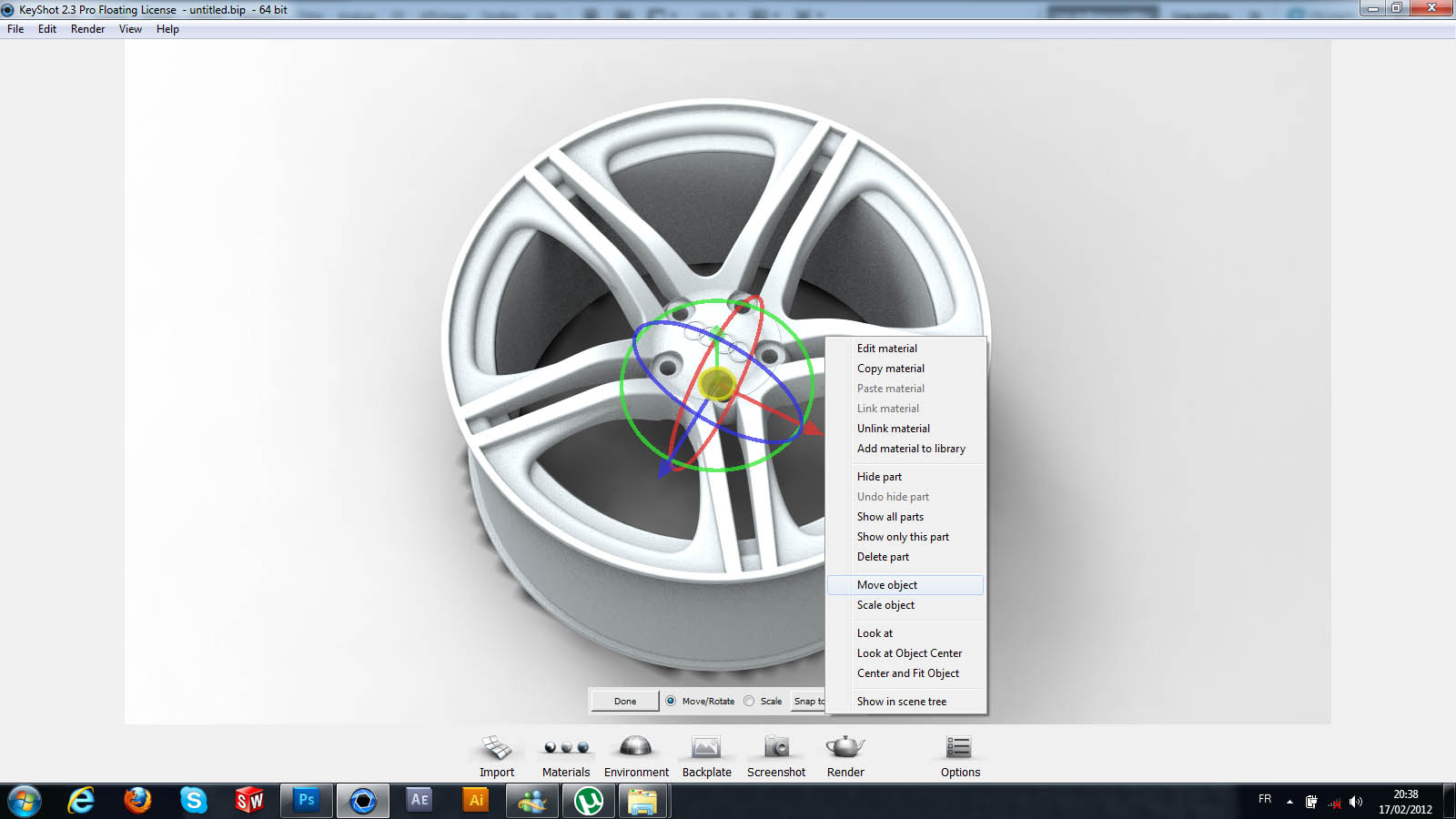63. ### Step 63:

and I apply a material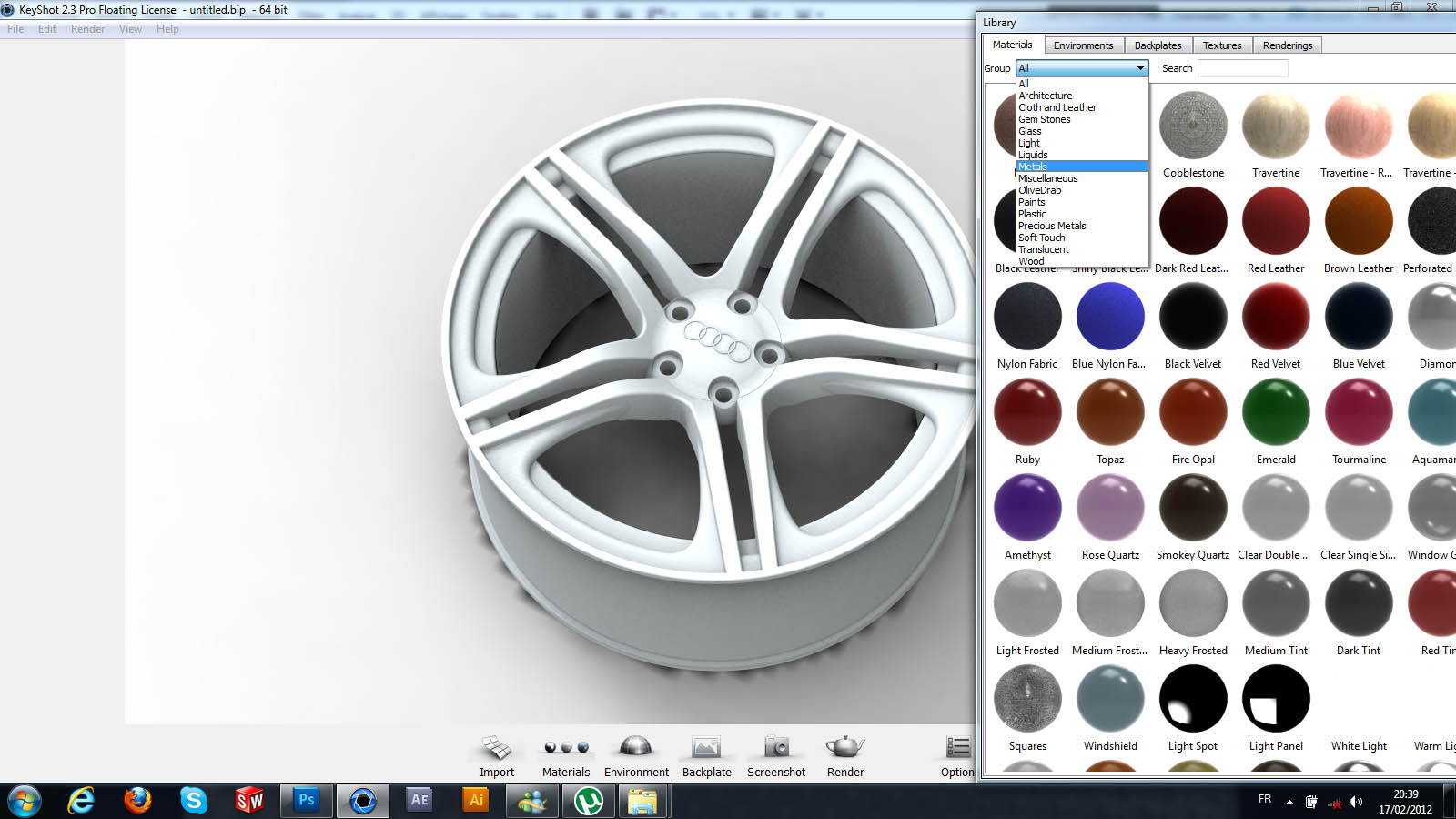64. ### Step 64:

here is the result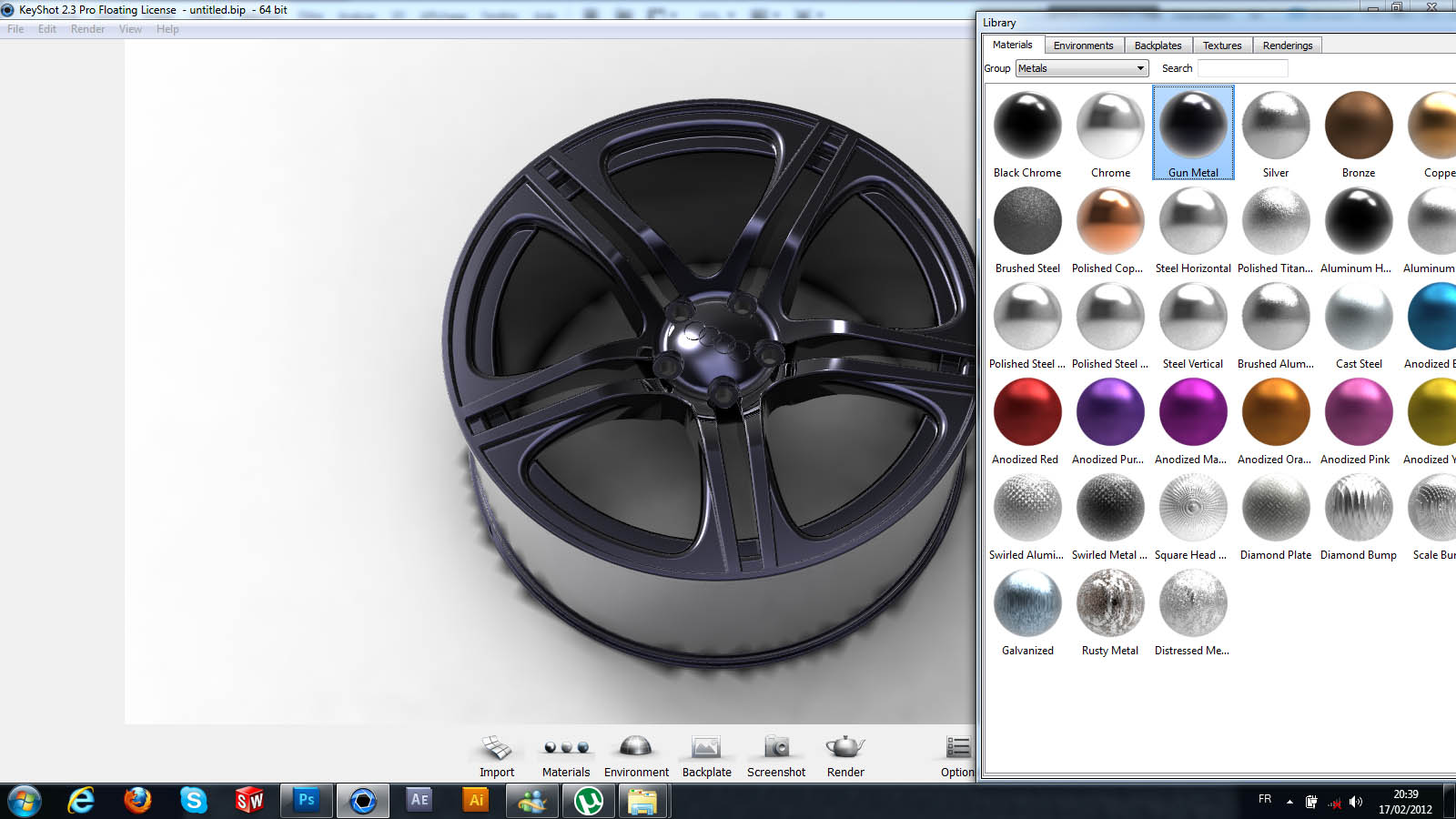65. ### Step 65:

.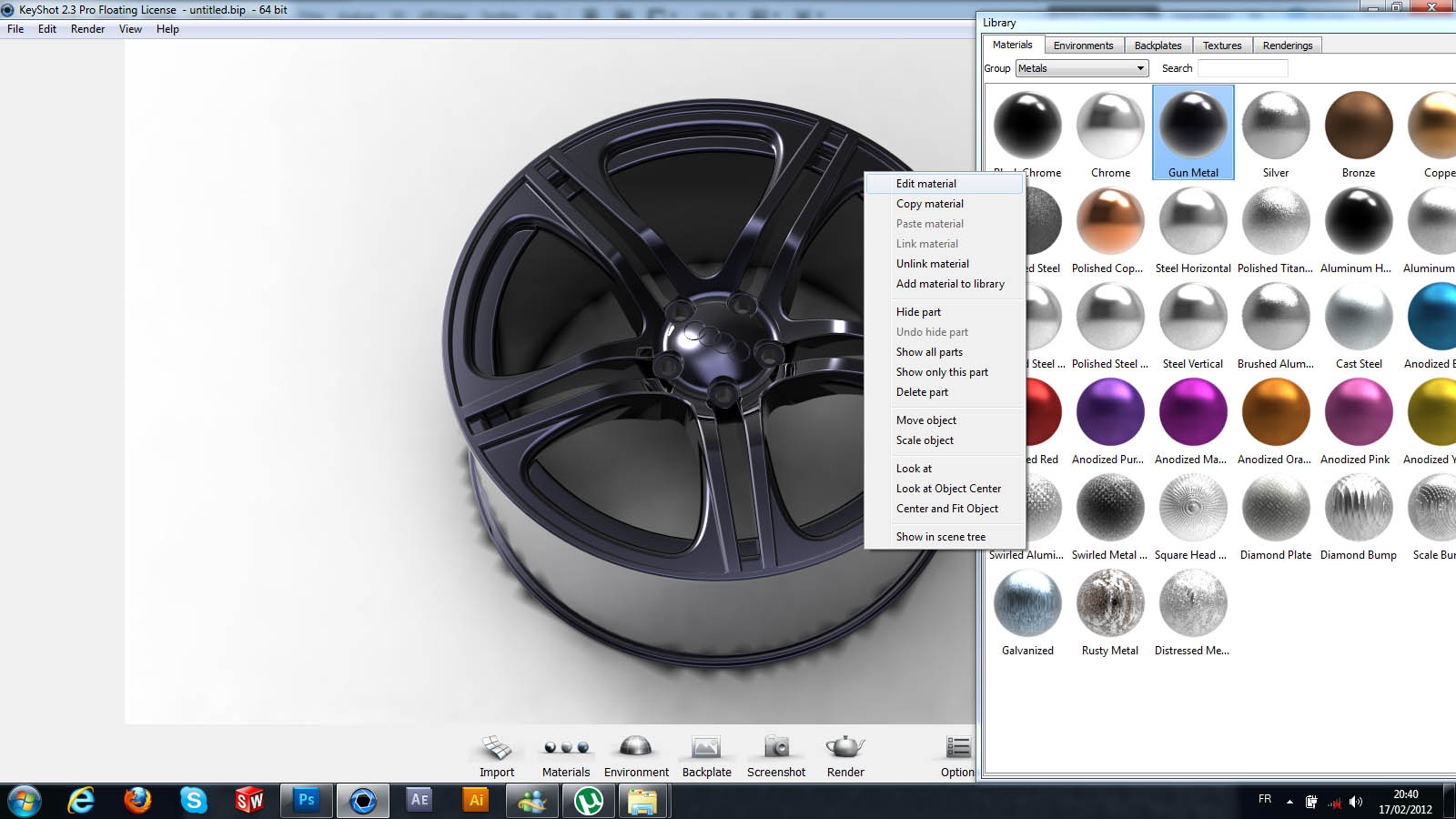66. ### Step 66:

.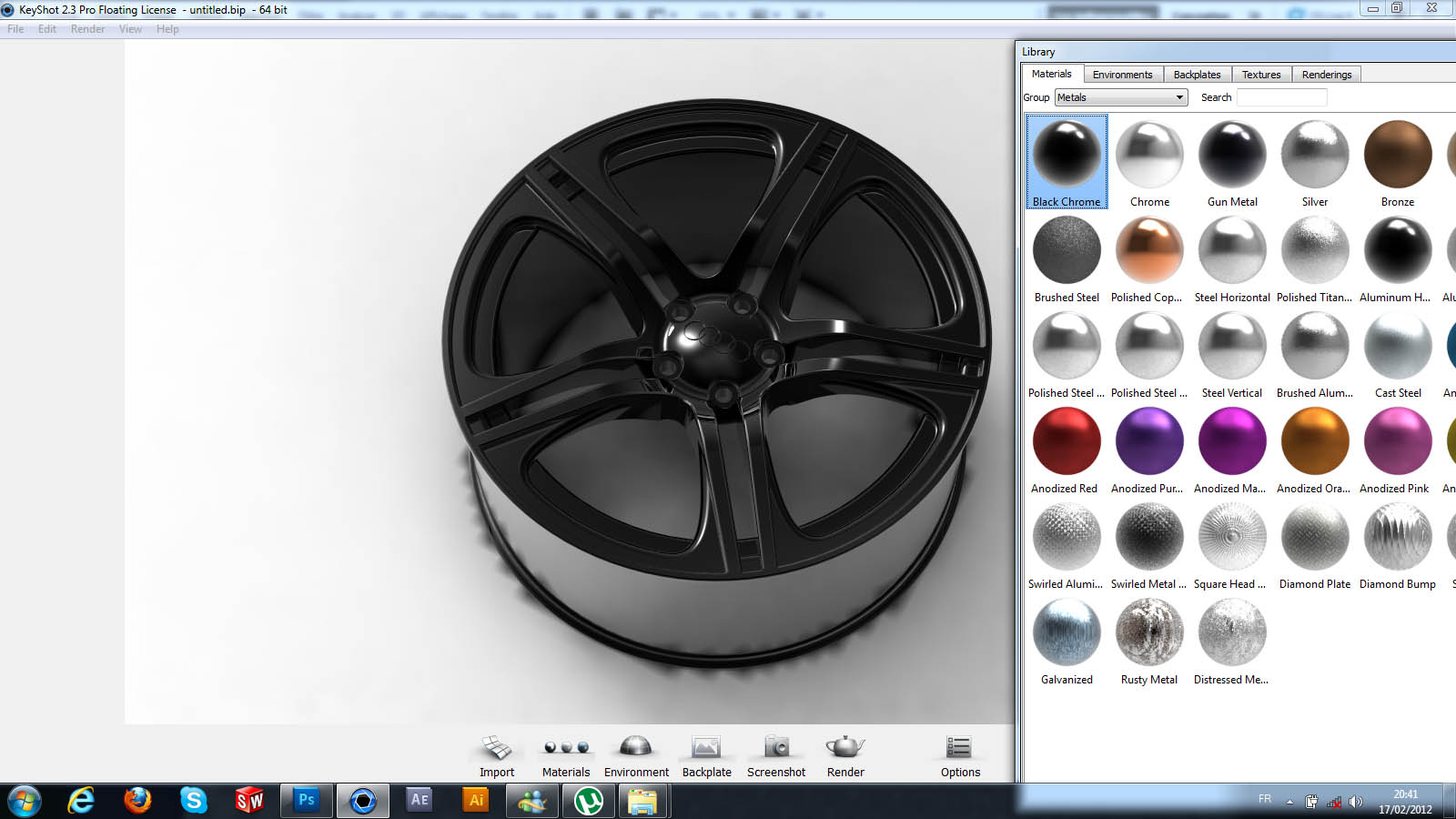67. ### Step 67:

.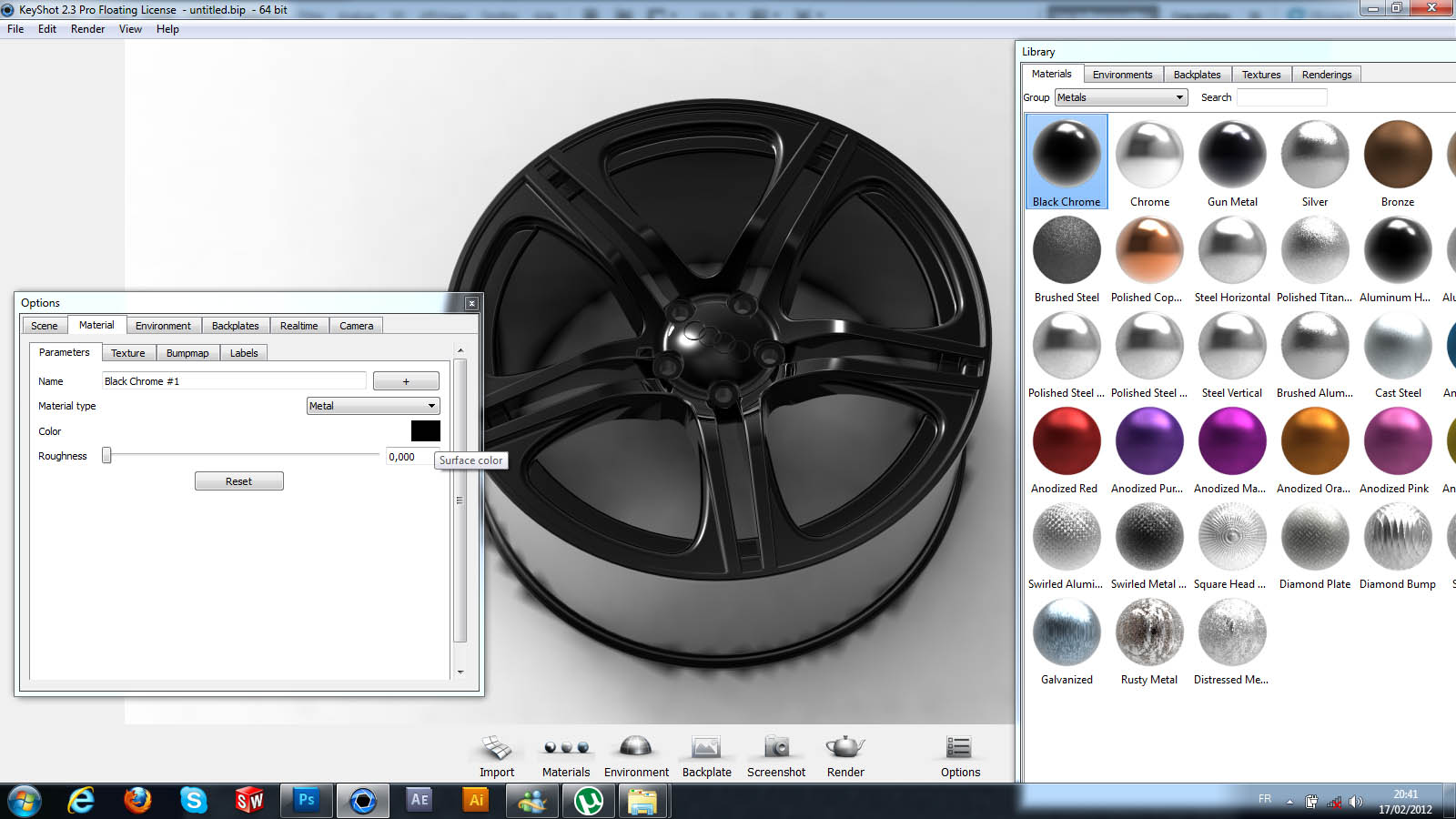68. ### Step 68:

.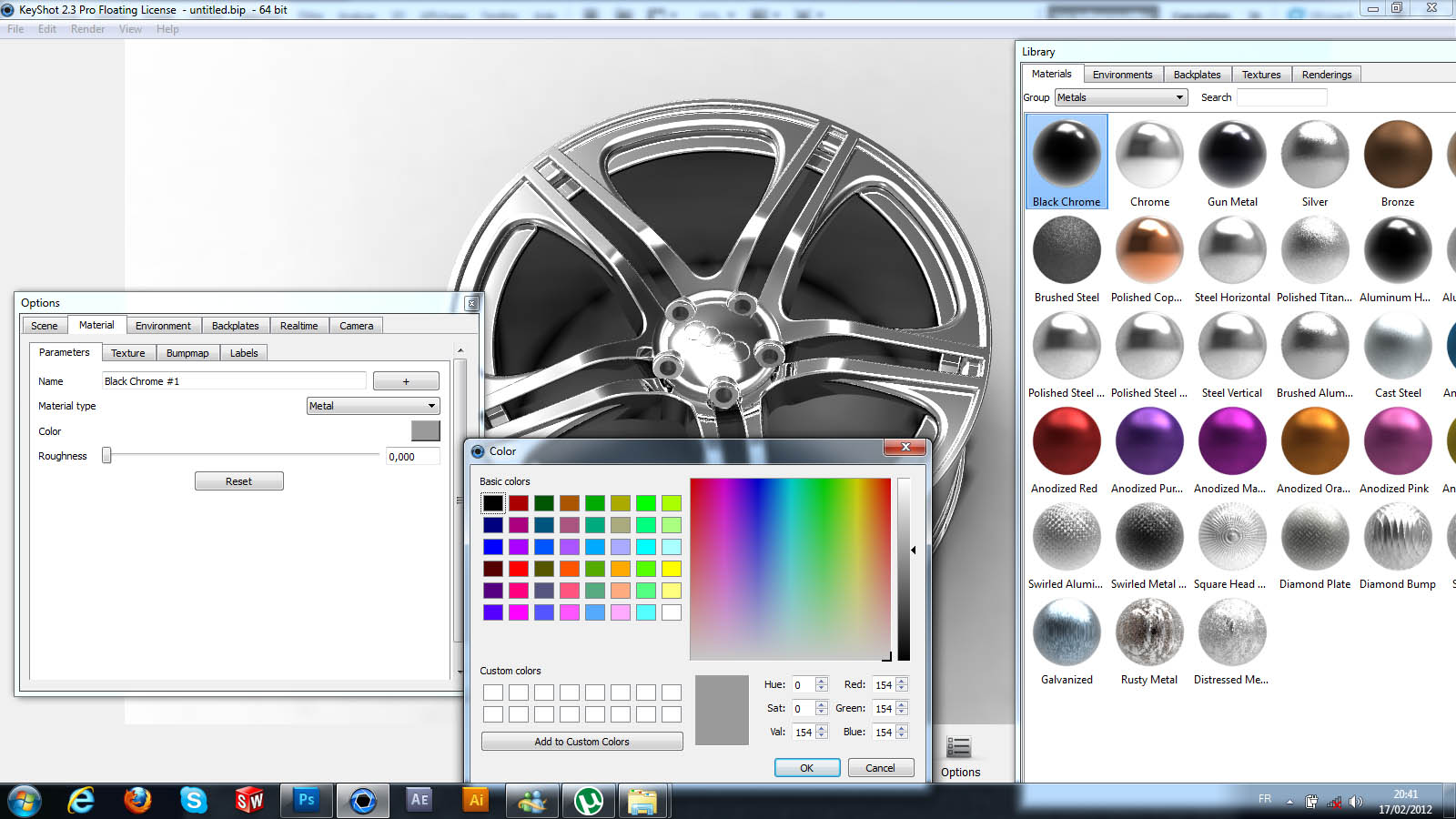69. ### Step 69:

.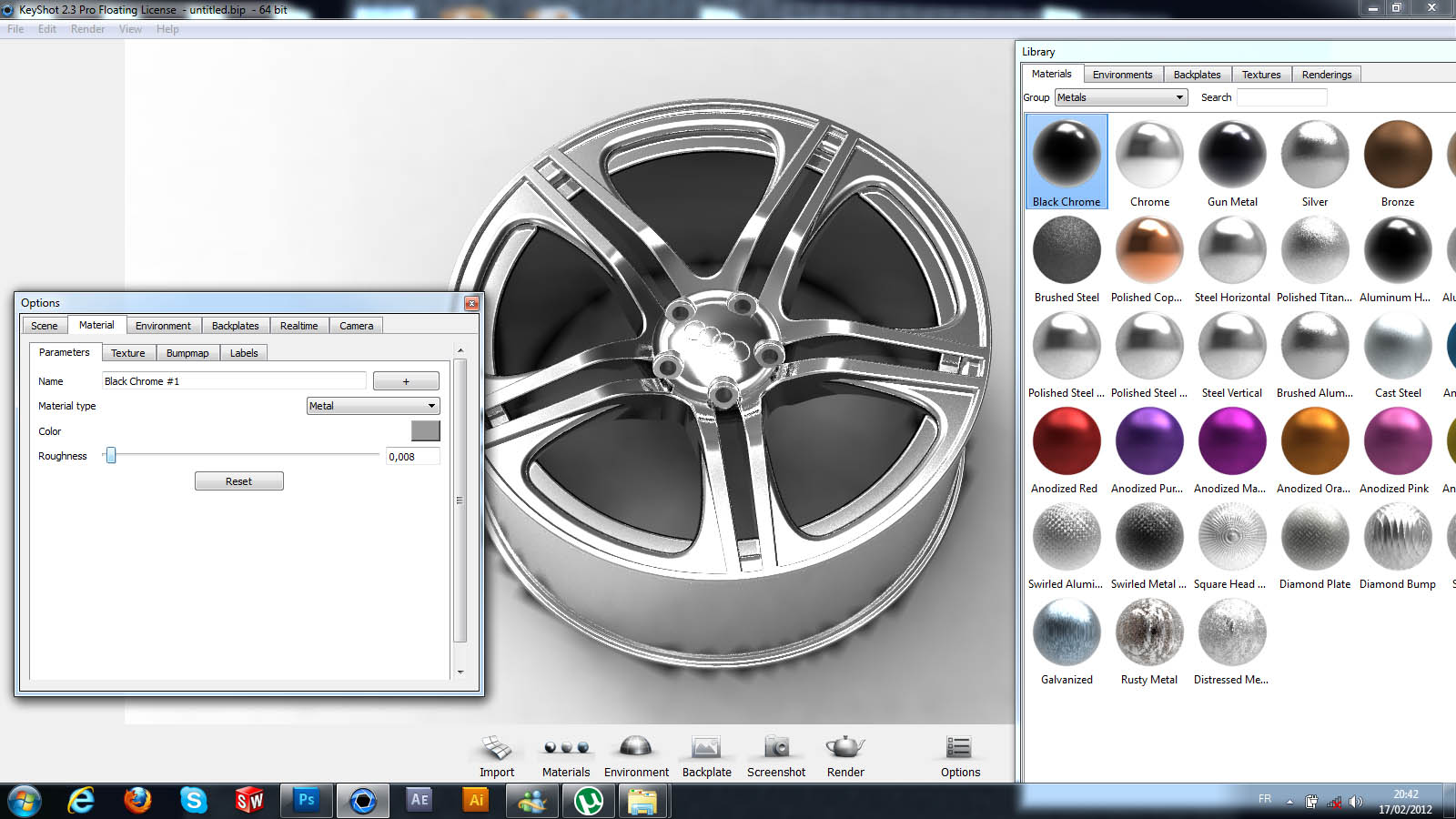70. ### Step 70:

.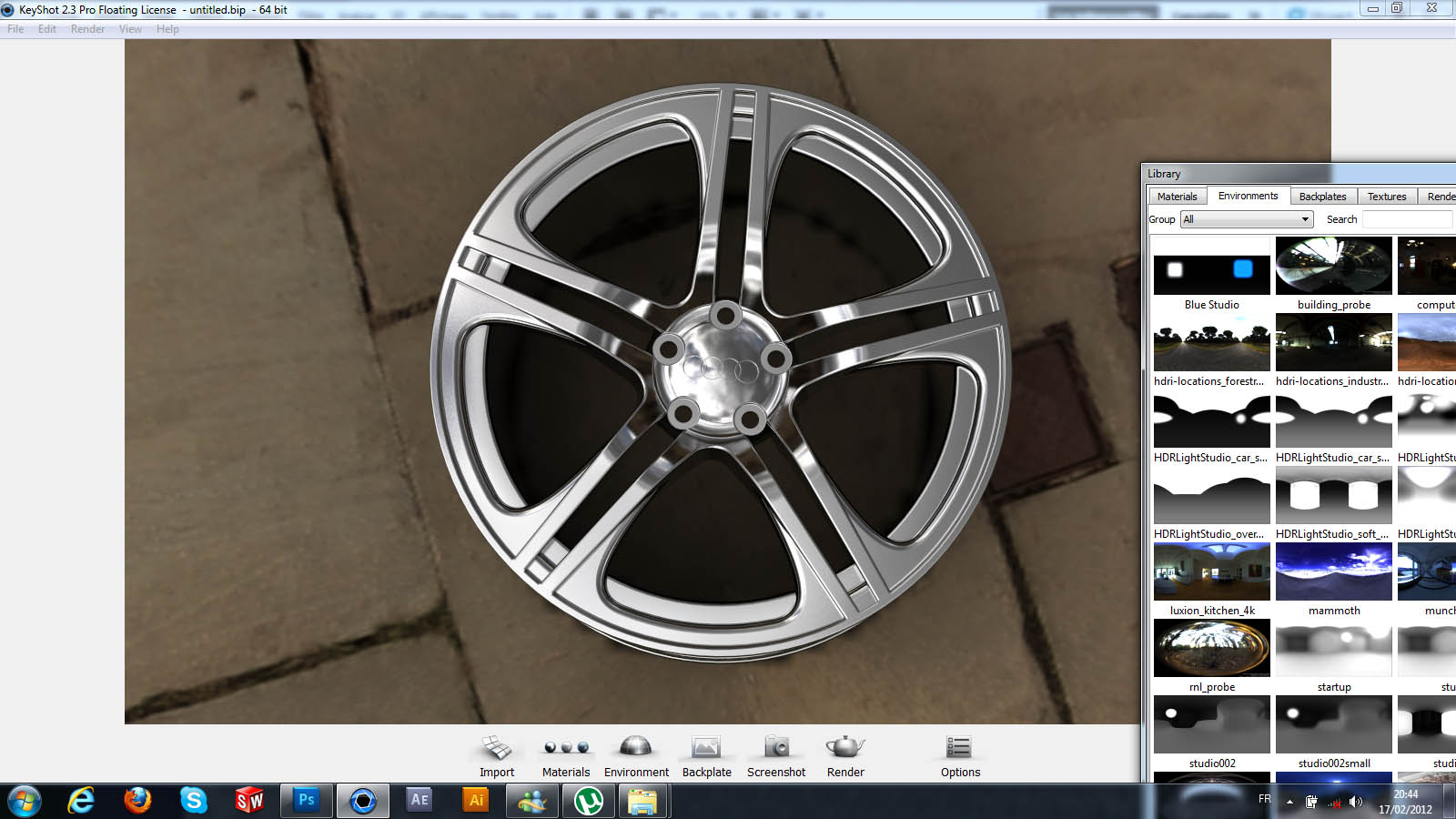71. ### Step 71:

.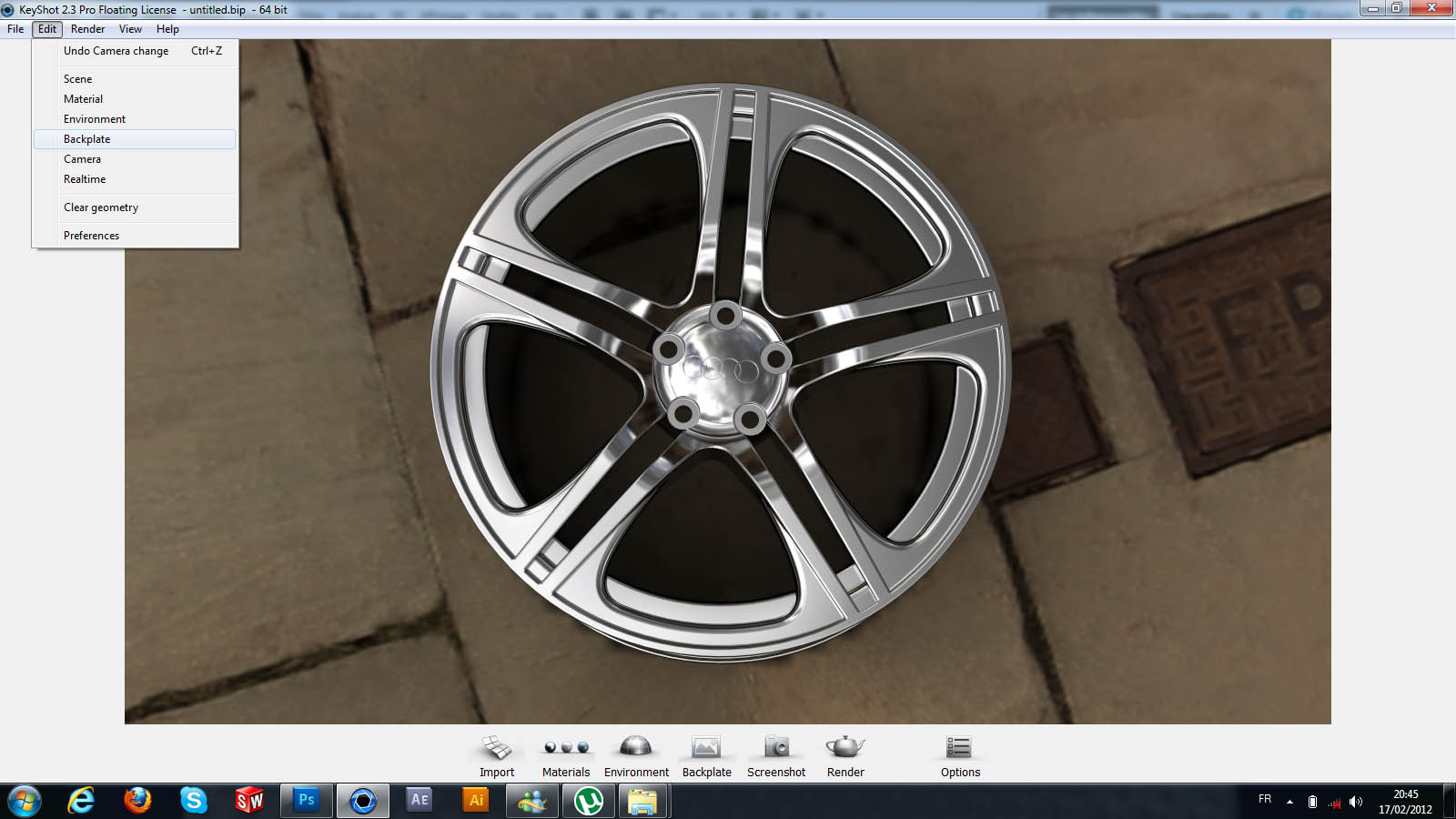72. ### Step 72:

.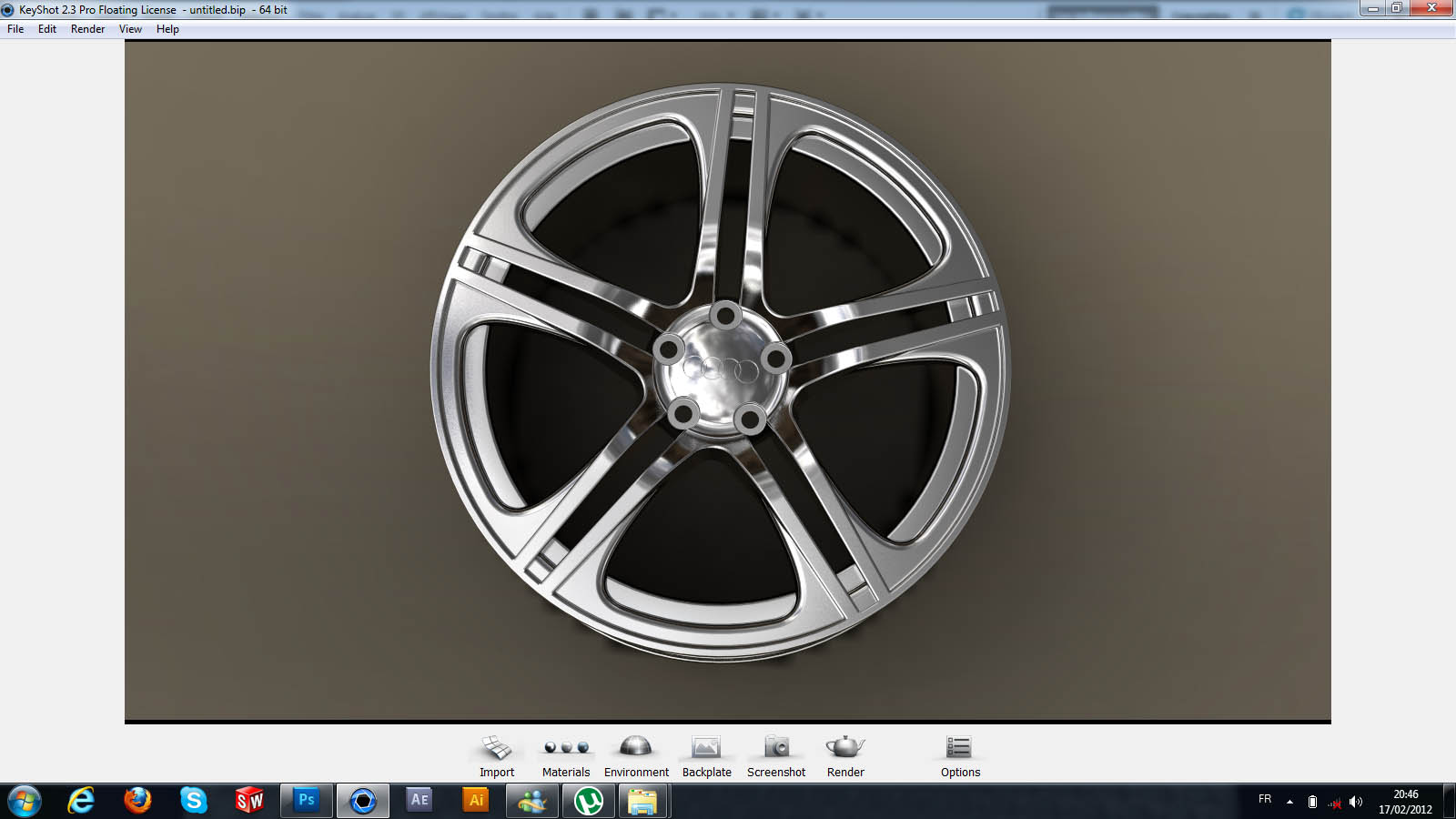73. ### Step 73:

.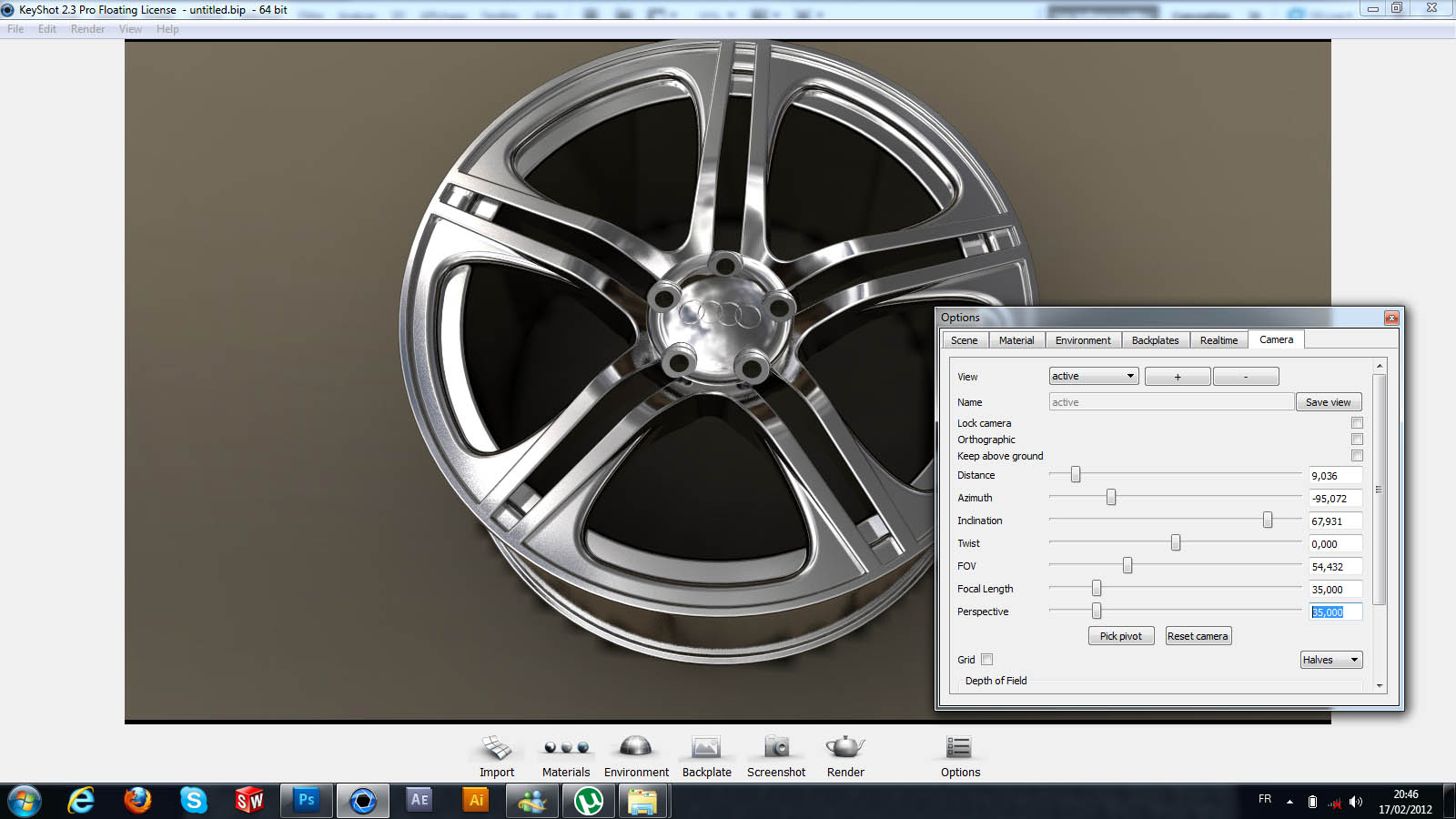74. ### Step 74:

.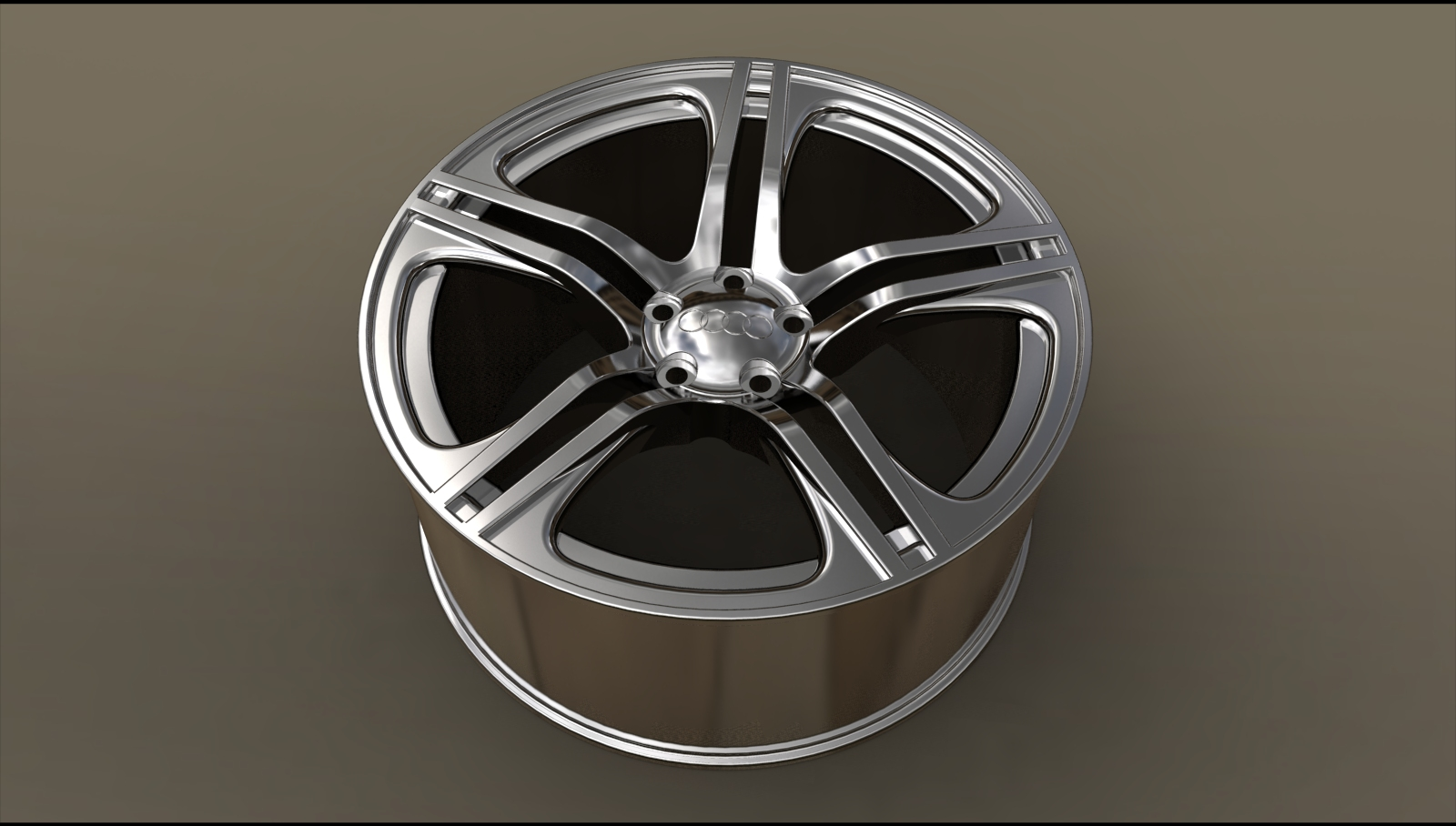.